# The Spin-Foam Approach to Quantum Gravity

## Abstract

This article reviews the present status of the spin-foam approach to the quantization of gravity. Special attention is payed to the pedagogical presentation of the recently-introduced new models for four-dimensional quantum gravity. The models are motivated by a suitable implementation of the path integral quantization of the Plebanski formulation of gravity on a simplicial regularization. The article also includes a self-contained treatment of 2+1 gravity. The simple nature of the latter provides the basis and a perspective for the analysis of both conceptual and technical issues that remain open in four dimensions.

## Quantum Gravity: A Unifying Framework for Fundamental Physics

The revolution brought about by Einstein’s theory of gravity lies more in the discovery of the principle of general covariance than in the form of the dynamical equations of general relativity. General covariance brings the relational character of nature into our description of physics as an essential ingredient for the understanding of the gravitational force. In general relativity, the gravitational field is encoded in the dynamical geometry of spacetime, implying a strong form of universality that precludes the existence of any non-dynamical reference system — or non-dynamical background — on top of which things occur. This leaves no room for the old view, where fields evolve on a rigid pre-established spacetime geometry (e.g., Minkowski spacetime): to understand gravity one must describe the dynamics of fields with respect to one another, and independent of any background structure.

General relativity realizes the requirements of general covariance as a classical theory, i.e., for h = 0. Einstein’s theory is, in this sense, incomplete as a fundamental description of nature. A clear indication of such incompleteness is the generic prediction of spacetime singularities in the context of gravitational collapse. Near spacetime singularities, the spacetime curvature and energy density become so large that any classical description turns inconsistent. This is reminiscent of the foundational examples of quantum mechanics — such as the UV catastrophe of black-body radiation or the instability of the classical model of the hydrogen atom — where similar singularities appear if quantum effects are not appropriately taken into account. General relativity must be replaced by a more fundamental description that appropriately includes the quantum degrees of freedom of gravity.

At first sight the candidate would be a suitable generalization of the formalism of quantum field theory (QFT). However, the standard QFTs used to describe other fundamental forces are not appropriate to tackle the problem of quantum gravity. Firstly, because standard QFTs are not generally covariant, as they can only be defined if a non-dynamical spacetime geometry is provided: the notion of particle, Fourier modes, vacuum and Poincaré invariance are essential tools that can only be constructed on a given spacetime geometry. This is a strong limitation when it comes to quantum gravity, since the very notion of spacetime geometry is most likely not defined in the deep quantum regime. Secondly, quantum field theory is plagued by singularities too (UV divergences) coming from the contribution of arbitrary high-energy quantum processes. This limitation of standard QFTs is expected to disappear once the quantum fluctuations of the gravitational field, involving the dynamic treatment of spacetime geometry, are appropriately taken into account. But because of its intrinsically-background-dependent definition, standard QFT cannot be used to shed light on this issue. A general covariant approach to the quantization of gravity is needed.

This is obviously not an easy challenge, as in the construction of a general covariant QFT one must abandon from the starting point most of the concepts that are essential in the description of ‘non-gravitational’ physics. One has to learn to formulate a quantum theory in the absence of preferred reference systems or pre-existent notion of space and time. Loop quantum gravity (LQG) is a framework to address this task. The degrees of freedom of gravity are quantized in accordance to the principles of general covariance. At the present stage there are some indications that both the singularity problems of classical general relativity as well as the UV problem of standard QFTs might vanish in the framework.

However, these indications are not conclusive, mainly because the systematic description of dynamical processes remains an open problem at the present stage. In this article we review the status of the construction of a promising approach for handling the difficult dynamical question in LQG: the spin-foam formulation.

### Why non-perturbative quantum gravity?

Let us make some observations about the problems of standard perturbative quantum gravity. In doing so we will revisit the general discussion above, in a special situation. In standard perturbative approaches to quantum gravity one attempts to describe the gravitational interaction using the same techniques applied to the definition of the standard model. As these techniques require a notion of a non-dynamical background, one (arbitrarily) separates the degrees of freedom of the gravitational field in terms of a background geometry η ab for a, b = 1 ⋯ 4 — fixed once and for all — and dynamical metric fluctuations h ab . Explicitly, one writes the spacetime metric as

$${g_{ab}} = {\eta _{ab}} + {h_{ab}}{.}$$
(1)

Notice that the previous separation of degrees of freedom has no intrinsic meaning in general relativity. In other words, for a generic spacetime metric g ab we can write

$${g_{ab}} = {\eta _{ab}} + {h_{ab}} = {\tilde \eta _{ab}} + {\tilde h_{ab}},$$
(2)

where η ab and ab can lead to different background light-cone structures of the underlying spacetime (M, g ab ); equally natural choices of flat background metrics lead to different Minkowski metrics in this sense. This is quite dangerous if we want to give any physical meaning to the background, e.g., the light-cone structures of the two ‘natural’ backgrounds will be generally different providing different notions of causality! Eq. (1) is used in the classical theory in very special situations when one considers perturbations of a given background η ab . In quantum gravity one has to deal with arbitrary superpositions of spacetimes; the above splitting can at best be meaningful for very special semi-classical states ‘peaked’, so to say, around the classical geometry η ab with small fluctuations, i.e., it might only make sense a posteriori in the framework of a quantum gravity theory defined independent of any background geometry. Consequently, even when perturbations remain useful to approximate special situations, the splitting in Eq. (1) is inconsistent when considering general states with their arbitrary quantum excitations at all scales.

This is especially so in gravity, due to the dual role of the gravitational field that simultaneously describes the geometry and its own dynamical degrees of freedom. More explicitly, in the standard background-dependent quantization, the existence of a fixed-background geometry is fundamental to the definition of the theory. In the usual treatment one imposes fields at space-like separated points to commute alluding to standard causality considerations. Even when this is certainly justified for matter fields (at least in the range of applicability of the standard model) it makes no sense in the case of gravity: one would be using the causal structure provided by the unphysical background η ab . This difficulty has been raised several times (see, for instance, ).

Therefore, treating the gravitational field according to the splitting given in Eq. (1) is inconsistent with the fundamental nature of the gravitational degrees of freedom. Treating h ab with the standard perturbative techniques is equivalent to viewing h ab as another matter field with no special properties. As mentioned above, gravitons would propagate respecting the causal structure of the unphysical background to all orders in perturbation theory!Footnote 1 Radiative corrections do not affect causality. Even though such a thing is quite sensible when dealing with matter fields in the regime where gravity is neglected, it would be clearly wrong for the gravitational interaction.

### Non-renormalizability of general relativity

If we ignore all these issues and try to set up a naive perturbative quantization of gravity, we find that the theory is non-renormalizable. This can be expected from dimensional analysis as the quantity playing the role of the coupling constant turns out to be the Planck length p . The non-renormalizability of perturbative gravity is often explained through an analogy with the (non-renormalizable) Fermi’s four-fermion effective description of the weak interaction. Fermi’s four fermions theory is known to be an effective description of the (renormalizable) Weinberg—Salam theory. The non-renormalizable UV behavior of Fermi’s four fermion interaction is a consequence of neglecting the degrees of freedom of the exchanged massive gauge bosons, which are otherwise disguised as the dimension-full coupling $${\Lambda _{{\rm{Fermi}}}} \approx 1/m_W^2$$ at momentum transfer much lower than the mass of the W particle $${q^2} \ll m_W^2$$. A similar view is applied to gravity to promote the search of a more fundamental theory, which is renormalizable or finite (in the perturbative sense) and reduces to general relativity at low energies. From this perspective it is argued that the quantization of general relativity is a hopeless attempt to quantize a theory that does not contain the fundamental degrees of freedom.

These arguments, based on background-dependent concepts, seem, at the very least, questionable in the case of gravity. Although one should expect the notion of a background geometry to be useful in certain semi-classical situations, the assumption that such structure exists all the way down to the Planck scale is inconsistent with what we know about gravity and quantum mechanics. General considerations indicate that standard notions of space and time are expected to fail near the Planck scale p .Footnote 2 In the field of LQG, people usually tend to interpret the severe divergences of perturbative quantum gravity as an indication of the inconsistency of the separation of degrees of freedom in Eq. (1). According to concrete results in LQG the nature of spacetime is very different from the classical notion in quantum gravity. The treatment that uses Eq. (1) as the starting point is assuming a well-defined notion of background geometry at all scales, which directly contradicts these results.

It is possible that new degrees of freedom would become important at more fundamental scales. It is also possible that including these degrees of freedom might be very important for the consistency of the theory of quantum gravity. However, there is a constraint that seems hardly avoidable: if we want to get a quantum theory that reproduces gravity in the semi-classical limit, we should have a background-independent formalism. In LQG one stresses this viewpoint. The hope is that learning how to define quantum field theory in the absence of a background is a key ingredient in a recipe for quantum gravity.

One of the achievements of the background independent formulation of LQG is the discovered intrinsic discreteness of quantum geometry. Geometric operators have discrete spectra yielding a physical mechanism for the cut-off of UV degrees of freedom around the Planck scale. In this way LQG is free of UV divergences. Is this a complete answer to the non-renormalizability problem of gravity mentioned above? Unfortunately, the answer to this question is in the negative at this stage. The reason is that the true answer to the question of renormalizabity or not renormalizability is not in the presence of divergences but in the degree of intrinsic ambiguity of the quantization recipe applied to a field theory.

In standard background dependent quantum field theories, in order to avoid UV divergences one has to provide a regularization prescription (e.g., an UV cutoff, dimensional regularization, point splitting, etc.). Removing the regulator is a subtle task involving the tuning of certain terms in the Lagrangian (counter-terms) that ensure finite results when the regulator is removed. In fact by taking special care in the mathematical definition of the products of distributions at the same point, in good cases, one can provide a definition of the quantum theory, which is completely free of UV divergences  (see also [343, 224, 225]). However, any of these regularization procedures is intrinsically ambiguous. The dimension of the parameter space of ambiguities depends on the structure of the theory. The right theory must be fixed by comparing predictions with observations (by the renormalization conditions). In loop quantum gravity there is strong indications that the mathematical framework of the theory provides a regularization of divergences. It remains to settle the crucial issue of how to fix the associated ambiguities.

According to the previous discussion, ambiguities associated with the UV regularization allow for the classification of theories as renormalizable or non-renormalizable quantum field theories. In a renormalizable theory such as QED there are finitely many ambiguities, which can be fixed by a finite number of renormalization conditions, i.e., one selects the suitable theory by appropriate tuning of the ambiguity parameters in order to match observations. In a non-renormalizable theory (e.g., perturbative quantum gravity) the situation is similar except for the fact that there are infinitely many parameters to be fixed by renormalization conditions. As the latter must be specified by observations, a non-renormalizable theory has very limited predictive power. In the case of gravity there is evidence indicating that the theory might be non-perturbatively renormalizable coming from the investigations of non trivial fix points of the renormalization group flow in truncated models (see  and references therein).

Removing UV divergences by a regularization procedure and ambiguities are two sides of the same coin quantum field theory. Although this can happen in different ways in particular formulations, the problem is intrinsic to the formalism of quantum field theory. In this respect, it is illustrative to analyze the non-perturbative treatment of gauge theories in the context of lattice gauge theory (where the true theory is studied by means of a regulated theory defined on a spacetime discretization or lattice). It is well known that here too the regulating procedure leads to ambiguities; the relevance of the example resides in the fact that these ambiguities resemble in nature those appearing in LQG. More precisely, let us take for concreteness SU(2) Yang-Mills theory, which can be analyzed non-perturbatively using the standard (lattice) Wilson action

$${S_{{\rm{LYM}}}} = {1 \over {{g^2}}}\sum\limits_p {\,\left({1 - {1 \over 4}{\rm{Tr}}[{U_p} + U_p^\dagger ]} \right)}{.}$$
(3)

In the previous equation, U p SU(2) is the holonomy around plaquettes p, and the sum is over all plaquettes of a regulating (hyper-cubic) lattice. It is easy to check that the previous action approximates the Yang-Mills action when the lattice is shrunk to zero for a fixed smooth field configuration. This property is referred to as the naive continuum limit. Moreover, the quantum theory associated with the previous action is free of any UV problem due to the UV cut-off provided by the underlying lattice.

Is this procedure unique? As is well known, the answer is no: one can regulate Yang-Mills theory equally well using the following action instead of Eq. (3):

$$S_{{\rm{LYM}}}^{(m)} \propto {1 \over {{g^2}}}\sum\limits_p {\,\left({1 - {1 \over {2(2m + 1)}}{\rm{T}}{{\rm{r}}^{(m)}}\left[ {{\Pi ^{(m)}}({U_p}) + {\Pi ^{(m)}}(U_p^\dagger)} \right]} \right)},$$
(4)

where Π(m) (U p ) denotes the SU(2) unitary irreducible representation matrix (of spin m) evaluated on the plaquette holonomy U p . Or, more generally, one can consider suitable linear combinations

$${S_{{\rm{LYM}}}} = \sum\limits_m {{a_m}S_{{\rm{LYM}}}^{(m)}}{.}$$
(5)

From the view point of the classical continuum theory, all these actions are equally good as they all satisfy the naive continuum limit. Do these theories approximate in a suitable sense the continuum quantum field theory as well? And are these ambiguities unimportant in describing the physics of quantum Yang-Mills theory? The answer to both of these questions is yes and the crucial property that leads to this is the renormalizability of Yang-Mills theory. Different choices of actions lead to different discrete theories. However, in the low-energy effective action, the differences appear only in local operators of dimension five or higher. This simple dimensional argument leads to the expectation that in the continuum limit (i.e., when the regulating lattice dependence is removed by shrinking it to zero) all the above theories should produce the same predictions in the sense that can safely ignore non-renormalizable contributions. This is indeed usually the case, and thus the ambiguities at the ‘microscopic level’ do not have any effect at low energies where one recovers quantum Yang-Mills theory.

Similar ambiguities are present in LQG; they appear in the regularization of the constraints in the canonical formulation . These ambiguities are also present in the spin-foam formulation, which is the central subject of this review. The simple dimensional argument given for Yang-Mills theory is no longer valid in the case of gravity, and a full dynamical investigation of their effect in physical predictions must be investigated. The spin-foam approach is best suited for getting the necessary insights into this important question.

## Why Spin Foams?

We now provide the basic motivation for the study and definition of spin foams. We present this motivation from the perspective of the canonical quantization approach known as loop quantum gravity (see [321, 359, 35]). In such context, spin foams appear as the natural tool for studying the dynamics of the canonically-defined quantum theory from a covariant perspective. Therefore, in order to introduce the spin-foam approach, it is convenient to start with a short introduction of the quantum canonical formulation.

### Loop quantum gravity

Loop quantum gravity is an attempt to define a quantization of gravity paying special attention to the conceptual lessons of general relativity. The theory is explicitly formulated in a background-independent, and therefore, non-perturbative fashion. The theory is based on the Hamiltonian (or canonical) quantization of general relativity in terms of variables that are different from the standard metric variables. In terms of these variables, general relativity is cast into the form of a background independent SU(2) gauge theory whose phase space structure is similar in various ways to that of SU(2) Yang-Mills theory (the key difference being the absence of any background metric structure). The main prediction of LQG is the discreteness  of the spectrum of geometrical operators such as area and volume. The discreteness becomes important at the Planck scale, while the spectrum of geometric operators crowds very rapidly at ‘low energy scales’ (large geometries). This property of the spectrum of geometric operators is consistent with the smooth spacetime picture of classical general relativity.

Thus, from the perspective of LQG, it is not surprising that perturbative approaches would lead to inconsistencies. In splitting the gravitational field degrees of freedom as in Eq. (1), one is assuming the existence of a background geometry, which is smooth all the way down to the Planck scale. As we consider contributions from ‘higher energies’, this assumption is increasingly inconsistent with the fundamental structure discovered in the non-perturbative treatment. However, despite the many achievements of LQG, there remain important issues to be addressed. At the heart of the completion of the definition of the theory, the clear-cut definition of quantum dynamics remains open. The spin-foam approach is one of the main avenues to exploring this problem.

The background independence of general relativity implies that the canonical formulation of the field theory is that of a gauge theory with diffeomorphism as part of the gauge group. LQG is constructed by quantizing a phase-space formulation of general relativity in terms of SU(2) connection variables. This introduces an extra SU(2) gauge symmetry group. The presence of gauge symmetries implies the existence of relations among phase space variables — defined on a spacelike initial-value hypersurface — known as constraints. These constraints define the Poisson algebra of infinitesimal generators of gauge transformations. There are three local constraints Gi — the Gauss constraints — generating SU(2) gauge transformations, three local constraints V a — the vector constraints — generating three dimensional diffeomorphisms of the initial spacelike hypersurface, and finally a scalar local constraint S related to the remaining gauge symmetry related to the four-diffeomorphism symmetry of the Lagrangian formulation.

The canonical quantization of systems with gauge symmetries is often called the Dirac program. The Dirac program [143, 223] applied to the quantization of general relativity in connection variables leads to the LQG approach.

The first step in the recipe consists in finding a representation of the phase-space variables of the theory as operators in a kinematical Hilbert space $${\mathcal H_{{\rm{kin}}}}$$ satisfying the standard commutation relations, i.e., {,} → −i/ħ[,]. This step has been successfully completed in LQG. One chooses the polarization where the SU(2) connection is the configuration variable. Unconstrained phase-space variables are replaced by the holonomy-flux algebra, which is represented by associated operators in a kinematical Hilbert space of suitable functionals of the generalized-connection ψ[A], which are square integrable with respect to the Ashtekar-Lewandowski  (gauge invariant and diffeomorphism invariant) measure AL[A]. A key input is the use of the holonomy-flux algebra as a starting point for quantization. Many peculiar properties of LQG follow from this choice, motivated by having an observable algebra leading to simple (diffeomorphism covariant) Poisson brackets.Footnote 3

The kinematical inner product is given by

$$\langle {\psi, \phi} \rangle = {\mu _{{\rm{AL}}}}[\bar \psi \phi ] = \int{d{\mu _{{\rm{AL}}}}[A]\,\bar \psi [A]\phi [A]}{.}$$
(6)

The representation of the basic unconstrained phase-space variables as suitable operators in $${\mathcal H_{{\rm{kin}}}}$$ used in LQG — which has the additional key property of containing a special state that is diffeomorphism invariant — has been shown to be unique .

The next step is to promote the constraints to (self-adjoint) operators in $${\mathcal H_{{\rm{kin}}}}$$ in such a way that the classical Poisson algebra is respected by the appropriate quantum commutator algebra (if this last part is achieved the quantization is said to be non-anomalous). In the case of gravity one must quantize the seven constraints G i , V a , and S. Both the Gauss constraint and (finite) diffeomorphism transformations have a natural (unitary) action on states on $${\mathcal H_{{\rm{kin}}}}$$ For that reason the quantization (and subsequent solution) is rather direct. The quantization of the scalar constraint presents difficulties. Concrete quantizations producing well-defined operators are available: no UV divergences are encountered by these proposals; the fundamental discreteness of quantum geometry in LQG plays the role of physical regulator at the Planck scale . Despite this partial success, problems partly related to special features of the constraint algebra (field-dependent structure constant) and partly related to the non-existence of an infinitesimal generator of diffeomorphism at the quantum level make the issue of whether any of the proposed quantizations of the constraints are anomaly-free a difficult open question.

In the third step one needs to characterize the space of solutions of the constraints and define the corresponding inner product that defines a notion of physical probability. This defines the physical Hilbert space $${\mathcal H_{{\rm{phys}}}}$$. In LQG, physical states are those which satisfy the quantum constraints that, in this sense, could be called Quantum Einstein’s equations. More precisely $$\Psi \in {{\mathcal H}_{{\rm{phys}}}}$$ if

$$\begin{array}{*{20}c} {{{\hat G}_i}(A,E){\vert}\Psi > = 0,} \\ {{{\hat V}_a}(A,E){\vert}\Psi > = 0,} \\ {\hat S(A,E){\vert}\Psi > = 0{.}\,\,} \\ \end{array}$$
(7)

The space of solutions of the first six equations is well understood. The space of solutions of quantum scalar constraint remains open. For some mathematically-consistent definitions of Ŝ, the characterization of the solutions is well understood . The definition of the physical inner product is still an open issue. The completion of this step is intimately dependent on the previously-mentioned consistency of the quantum constraint algebra.

Finally, physical interpretation necessitates the definition of a (complete) set of gauge-invariant observables, i.e., operators commuting with the constraints. They represent the questions that are well posed in a generally covariant quantum theory. Already in classical gravity the construction of gauge-independent quantities is a subtle issue. At the present stage of the approach, physical observables are explicitly known only in some special cases. However, understanding the set of physical observables is intimately related to the problem of characterizing the solutions of the scalar constraint described before.

The spin-foam approach was constructed as a means to tackle the difficult question of dynamics and the definition of observable quantities in LQG. It is an attempt to address the difficulties associated with the last three steps of the list given above by rethinking the problem of dynamics from a path-integral covariant perspective. So we first need to briefly discuss the special features of the path integral formulation in the case of generally-covariant systems.

### The path integral for generally covariant systems

LQG is based on the canonical (Hamiltonian) quantization of general relativity, whose gauge symmetry is diffeomorphism invariance. In the Hamiltonian formulation the presence of gauge symmetries  gives rise to the constraints mentioned in Section 2.1. In the present discussion we will schematically represent the latter by the equation C(p, q) = 0 for (p, q) Γ. The constraints restrict the set of possible states of the theory by requiring them to lay on the constraint hyper-surface. In addition, through the Poisson bracket, the constraints generate motion associated to gauge transformations on the constraint surface (see Figure 2). The set of physical states (the reduced phase space Γred) is isomorphic to the space of orbits, i.e., two points on the same gauge orbit represent the same state in Γred described in different gauges (Figure 2).

In general relativity the absence of a preferred notion of time implies that the Hamiltonian of gravity is a linear combination of constraints. This means that Hamilton equations cannot be interpreted as time evolution and rather correspond to motion along gauge orbits of general relativity. In generally-covariant systems, conventional time evolution is pure gauge: from an initial data satisfying the constraints one recovers a spacetime by selecting a particular one-parameter family of gauge-transformations (in the standard ADM context this amounts to choosing a particular lapse field N(x, t) and shift Na(x, t)).

From this perspective the notion of spacetime becomes secondary and the dynamical interpretation of the theory seems problematic (in the quantum theory this is referred to as the problem of time). A reason for this is the central role played by the spacetime representation of classical gravity solutions, which is useful only if it can be interpreted via the notion of test observers (or more generally test fields).Footnote 4 Due to the fact that this idealization is a good approximation to the (classical) process of observation, the notion of spacetime is a useful concept only in classical gravity.

As emphasized by Einstein with his hole argument (see  for a modern explanation) only the information in relational statements (independent of any spacetime representation) have physical meaning. In classical gravity it remains useful to have a spacetime representation when dealing with idealized test observers. For instance, to solve the geodesic equation and then ask diff-invariant-questions such as: what is the proper time elapsed on particle 1 between two successive crossings with particle 2? However, already in the classical theory the advantage of the spacetime picture becomes, by far, less clear if the test particles are replaced by real objects coupling to the gravitational field.Footnote 5

The possibility of using the notion of test observers and test fields to construct our interpretative framework is no longer available in quantum gravity. At the Planck scale ( p ) the quantum fluctuations of the gravitational field become so important that there is no way (not even in principleFootnote 6) to make observations without affecting the gravitational field. The notion of test fields and test observer is lost and with it the usual spacetime representation. In this context there cannot be any, a priori, notion of time and hence no notion of spacetime is possible at the fundamental level. A spacetime picture would only arise in the semi-classical regime with the identification of some subsystems that approximate the notion of test observers.

Then what is the meaning of the path integral in such a background-independent context? The previous discussion rules out the conventional interpretation of the path integral. There is no meaningful notion of transition amplitude between states at different times t1 > t0 or equivalently a notion of “unitary time evolution” represented by an operator U(t1 − t0). Nevertheless, a path integral representation of generally covariant systems exists and arises as a tool for implementing the constraints in the quantum theory as we argue below.

Due to the difficulty associated with the explicit description of the reduced phase space Γred, in LQG one follows Dirac’s prescription. One starts by quantizing unconstrained phase space Γ, representing the canonical variables as self-adjoint operators in a kinematical Hilbert space $${\mathcal H_{{\rm{kin}}}}$$. Poisson brackets are replaced by commutators in the standard way, and the constraints are promoted to self-adjoint operators (see Figure 2). If there are no anomalies the Poisson algebra of classical constraints is represented by the commutator algebra of the associated quantum constraints. In this way the quantum constraints become the infinitesimal generators of gauge transformations in $${\mathcal H_{{\rm{kin}}}}$$. The physical Hilbert space $${\mathcal H_{{\rm{phys}}}}$$ is defined as the kernel of the constraints, and hence to gauge invariant states. Assuming for simplicity that there is only one constraint we have

$$\psi \in {{\mathcal H}_{{\rm{phys}}}}\quad {\rm{iff}}\quad \exp [iN\hat C]{\vert}\psi \rangle = {\vert}\psi \rangle \quad \forall \quad N \in {\mathbb R},$$

where U(N) = exp[iNĈ] is the unitary operator associated to the gauge transformation generated by the constraint C with parameter N. One can characterize the set of gauge-invariant states, and hence construct $${\mathcal H_{{\rm{phys}}}}$$, by appropriately defining a notion of ‘averaging’ along the orbits generated by the constraints in $${\mathcal H_{{\rm{kin}}}}$$. For instance, if one can make sense of the projector

$$P:{{\mathcal H}_{{\rm{kin}}}} \rightarrow {{\mathcal H}_{{\rm{phys}}}}\quad {\rm{where}}\quad P: = \int {dN} \,\,U(N){.}$$
(8)

It is apparent from the definition that for any $$\psi \in {{\mathcal H}_{{\rm{kin}}}}$$ kin then $$P\psi \in {{\mathcal H}_{{\rm{phys}}}}$$. The path integral representation arises in the representation of the unitary operator U(N) as a sum over gauge-histories in a way that is technically analogous to the standard path integral in quantum mechanics. However, the physical interpretation is quite different. The spin-foam representation arises naturally as the path-integral representation of the field theoretical analog of P in the context of LQG. Needless to say, many mathematical subtleties appear when one applies the above formal construction to concrete examples (see  and references therein). In the second part of this review we will illustrate in complete detail all the features of the path integral approach for generally covariant systems, formally described here, in the 2+1 pure gravity solvable model. In four dimensions, the spin-foam approach is a program in progress, where various issues still remain open. We will describe the various models in the first part of this article.

### A brief history of spin foams in four dimensions

In this section, we briefly describe the various spin-foam models for quantum gravity in the literature (for previous reviews on spin foams, see [293, 39, 302, 301, 248, 20]).

#### The Reisenberger model

According to Plebanski , the action of self-dual Riemannian gravity can be written as a constrained SU(2) BF theory

$$S(B,A) = \int {{\rm Tr}[B \wedge F(A)] - {\psi _{ij}}\left[ {{B^i} \wedge {B^j} - {1 \over 3}{\delta ^{ij}}{B^k} \wedge {B_k}} \right],}$$
(9)

where variations with respect to the symmetric (Lagrange multiplier) tensor ψ ij imposes the constraints

$${\Omega ^{ij}} = {B^i} \wedge {B^j} - {1 \over 3}{\delta ^i}^j{B^k} \wedge {B_k} = 0{.}$$
(10)

When B is non-degenerate the constraints are satisfied if and only if $${B^i} = \pm ({{e^0} \wedge {e^i} + {1 \over 2}{\epsilon ^i}_{jk}{e^i} \wedge {e^k}})$$, which reduces the previous action to that of self-dual general relativity. Reisenberger studied the simplicial discretization of this action in  as a preliminary step toward the definition of the corresponding spin-foam model. The consistency of the simplicial action is argued by showing that the simplicial theory converges to the continuum formulation when the triangulation is refined: both the action and its variations (equations of motion) converge to those of the continuum theory.

In , Reisenberger constructs a spin-foam model for this simplicial theory by imposing the constraints Ωij directly on the SU(2) BF amplitudes. The spin-foam path integral for BF theory is obtained as in Section 14. The constraints are imposed by promoting the Bi to operators χi (combinations of left/right invariant vector fields) acting on the discrete connection.Footnote 7 The model is defined as

$${Z_{GR}} = \underbrace {\int {\prod\limits_{e \in {{\mathcal J}_\Delta}} {d{g_e}}}}_{\int {{\mathcal D}[A]}}\overbrace {\delta ({{\hat \Omega}^{ij}})}^{\int {{\mathcal D}[\psi ]} \,\,{e^{i{\psi _{ij}}{\Omega ^{ij}}}}}\underbrace {\sum\limits_{{\mathcal C}:\{j\} \rightarrow \{f\}} {\prod\limits_{f \in {{\mathcal J}_\Delta}} {{\Delta _{{j_f}}}\,{\rm{Tr}}\,[{j_f}(g_e^1 \ldots g_e^N)],}}}_{\int {{\mathcal D}[B]\,\,{e^{i\int {\rm{Tr}}[B \wedge F(A)]}}}}$$
(12)

where $$\hat \Omega = {{\mathcal J}^i} \wedge {{\mathcal J}^j} - {1 \over 3}{\delta ^{ij}}{{\mathcal J}^k} \wedge {{\mathcal J}_k}$$ and we have indicated the correspondence of the different terms with the continuum formulation. The preceding equation is rather formal; for the rigorous implementation see . Reisenberger uses locality so that constraints are implemented on a single 4-simplex amplitude. However, there is a difficulty with this procedure: the algebra of operators $${\hat \Omega ^{ij}}$$ do not close so that imposing the constraints sharply becomes a too strong condition on the BF configurations.Footnote 8. In order to avoid this, Reisenberger defines a one-parameter family of models by inserting the operator

$${e^{- {1 \over {2{z^2}}}{{\hat \Omega}^2}}}$$
(13)

instead of the delta function above. In the limit z → ∞ the constraints are sharply imposed. This introduces an extra parameter to the model. The properties of the kernel of $$\hat \Omega$$ have not been studied in detail.

#### The Freidel-Krasnov prescription

Freidel and Krasnov  define a general framework to construct spin-foam models corresponding to theories, whose action has the general form

$$S(B,A) = \int {{\rm{Tr}}[B \wedge F(A)] + \Phi (B),}$$
(14)

where the first term is the BF action, while Φ(B) is a certain polynomial function of the B field. The formulation is constructed for compact internal groups. The definition is based on the formal equation

$$\int {{\mathcal D}[B]{\mathcal D}[A]} \,{e^{i\int {\rm{Tr}}[B \wedge F(A)] + \Phi (B)}}: = {\left. {{e^{i\int \Phi \left({{\delta \over {\delta J}}} \right)}}Z[J]} \right\vert_{J = 0}},$$
(15)

where the generating functional Z[J] is defined as

$$Z[J]: = \int {{\mathcal D}[B]{\mathcal D}[A]} \,{e^{i\int {{\rm{Tr}}} [B \wedge F(A)] + {\rm{Tr}}[B \wedge J]}},$$
(16)

where J is an algebra-valued 2-form field. They provide a rigorous definition of the generating functional by introducing a discretization of $${\mathcal M}$$ in the same spirit of the other spin-foam models discussed here. Their formulation can be used to describe various theories of interest such as BF theories with cosmological terms, Yang-Mills theories (in 2 dimensions) and Riemannian self-dual gravity. In the case of self-dual gravity B and A are valued in su(2), while

$$\Phi (B) = \int {{\psi _{ij}}} \left[ {{B^i} \wedge {B^j} - {1 \over 3}{\delta ^{ij}}{B^k} \wedge {B_k}} \right],$$
(17)

according to Eq. (9). The model obtained in this way is very similar to Reisenberger’s one. However, there are some technical differences. One of the more obvious is that the non-commutative invariant vector fields $${{\mathcal J}^i}$$ representing Bi are replaced here by the commutative functional derivatives δ/δJi.

The idea of using such generating functional techniques has regained interest in the context of the McDowell-Mansouri formulation of general relativity . The interest of the latter formulation is that it provides an action of gravity that is given by a BF term plus a genuine potential term instead of constraints. The implementation of the spin-foam quantization of such a formulation is investigated in [349, 346, 189]; see also  for an important remark on the approach. More recently similar techniques have been used in .

#### The Iwasaki model

Iwasaki defines a spin-foam model of self-dual Riemannian gravityFootnote 9 by a direct lattice discretization of the continuous Ashtekar formulation of general relativity. The action is

$$S(e,A) = \int {d{x^4}} {\epsilon ^{\mu \nu \lambda \sigma}}\left[ {2\,e_{\left[\mu\right.}^0{e_{\nu \left]i\right.}} + {\epsilon ^0}_{ijk}e_\mu ^je_\nu ^k} \right]\,\left[ {2\,{\partial _{\left[\lambda\right.}}A_{\sigma \left]\right.}^i + {\epsilon ^{0i}}_{lm}A_\lambda ^lA_\sigma ^m} \right],$$
(18)

where $$A_a^i$$ is an SU(2) connection. The fundamental observation of  is that one can write the discrete action in a very compact form if we encode part of the degrees of freedom of the tetrad in an SU(2) group element. More precisely, if we take $${g_{\mu \mu}} = e_\mu ^ie_\mu ^j{\delta _{ij}} = 1$$, we can define $${{\rm{e}}_\mu}: = e_\mu ^0 + i{\sigma _i}e_\mu ^i \in SU\,(2)$$ where σ i are the Pauli matrices. In this parameterization of the ‘angular’ components of the tetrad and using a hypercubic lattice the discrete action becomes

$${S_\Delta} = - \beta \sum\limits_{v \in \Delta} {{\epsilon ^{\mu \nu \lambda \sigma}}{r_\mu}{r_\nu}\,\,{\rm{Tr}}[{\bf{e}}_\mu ^\dagger {{\bf{e}}_\nu}{U_{\lambda \sigma}}],}$$
(19)

where $${r_\mu}: = {({\beta ^{1/2}}{\ell _p})^{- 1}}\epsilon \sqrt {{g_{\mu \mu}},}$$ U μν is the holonomy around the μν-plaquette, ϵ is the lattice constant and β is a cutoff for r μ used as a regulator (r μ β1/2 p ϵ−1). The lattice path integral is defined by using the Haar measure both for the connection and the ‘spherical’ part of the tetrad e’s and the radial part $$d{r_\mu}: = d{r_\mu}r_\mu ^3$$. The key formula to obtain an expression involving spin foams is

$${e^{ix{\rm{Tr}}[U]}} = \sum\limits_j {(2j + 1){{{J_{2j + 1}}(2x)} \over x}} {\chi _j}(U){.}$$
(20)

Iwasaki writes down an expression for the spin-foam amplitudes in which the integration over the connection and the e’s can be computed explicitly. Unfortunately, the integration over the radial variables r involves products of Bessel functions and its behavior is not analyzed in detail. In 3 dimensions the radial integration can be done and the corresponding amplitudes coincide with the results of Section 14.5.

#### The Barrett-Crane model

The appealing feature of the previous models is the clear connection to LQG, since they are defined directly using the self-dual formulation of gravity (boundary states are SU(2)-spin networks). The drawback is the lack of closed simple expressions for the amplitudes, which complicates their analysis. However, there is a simple model that can be obtained as a systematic quantization of the simplicial SO(4) Plebanski’s action. This model was introduced by Barrett and Crane in  and further motivated by Baez in . The basic idea behind the definition was that of the quantum tetrahedron introduced by Barbieri in  and generalized to 4D in . The beauty of the model resides in its remarkable simplicity. This has stimulated a great deal of explorations and produced many interesting results.

#### Markopoulou-Smolin causal spin networks

Using the kinematical setting of LQG with the assumption of the existence of a micro-local (in the sense of Planck scale) causal structure, Markopoulou and Smolin define a general class of (causal) spin-foam models for gravity [267, 264] (see also ). The elementary transition amplitude $${A_{{s_I} \rightarrow {s_{I + 1}}}}$$ from an initial spin network s I to another spin network sI+1 is defined by a set of simple combinatorial rules based on a definition of causal propagation of the information at nodes. The rules and amplitudes have to satisfy certain causal restrictions (motivated by the standard concepts in classical Lorentzian physics). These rules generate surface-like excitations of the same kind we encounter in the more standard spin-foam model, but endow the foam with a notion of causality. Spin foams $${\mathcal F}_{{s_i} \rightarrow {s_f}}^N$$ are labeled by the number of times these elementary transitions take place. Transition amplitudes are defined as

$$\langle {{s_i},{s_f}} \rangle = \sum\limits_N {\prod\limits_{I = 0}^{N - 1} {{A_{{s_I} \rightarrow {s_{I + 1}}}}}}{.}$$
(21)

The models are not related to any continuum action. The only guiding principles are the restrictions imposed by causality, simplicity and the requirement of the existence of a non-trivial critical behavior that would reproduce general relativity at large scales. Some indirect evidence of a possible non-trivial continuum limit has been obtained in some versions of the model in 1+1 dimensions.

#### Gambini-Pullin model

Gambini and Pullin  introduced a very simple model obtained by modification of the BF theory skein relations. As we argued in Section 14, skein relations defining the physical Hilbert space of BF theory are implied by the spin-foam transition amplitudes. These relations reduce the large kinematical Hilbert space of BF theory (analogous to that of quantum gravity) to a physical Hilbert space corresponding to the quantization of a finite number of degrees of freedom. Gambini and Pullin define a model by modifying these amplitudes so that some of the skein relations are now forbidden. This simple modification frees local excitations of a field theory. A remarkable feature is that the corresponding physical states are (in a certain sense) solutions to various regularizations of the scalar constraint for (Riemannian) LQG. The fact that physical states of BF theory solve the scalar constraint is well known , since roughly F(A) = 0 implies EEF(A) = 0. The situation here is of a similar nature, and — as the authors argue — one should interpret this result as an indication that some ‘degenerate’ sector of quantum gravity might be represented by this model. The definition of this spin-foam model is not explicit since the theory is directly defined by the physical skein relations.

#### Capovilla-Dell-Jacobson theory on the lattice

The main technical difficulty that we gain in going from 3-dimensional general relativity to the 4-dimensional one is that the integration over the e’s becomes intricate. In the Capovilla-Dell-Jacobson [110, 109] formulation of general relativity this ‘integration’ is partially performed at the continuum level. The action is

$$S(\eta, A) = \int {\eta {\rm{Tr}}[\epsilon \,\cdot\, F(A) \wedge F(A)\epsilon \,\cdot\, F(A) \wedge F(A)],}$$
(22)

where e · FF:= ϵabcd F ab F cd . Integration over η can be formally performed in the path integral and we obtain

$$Z = \int {\prod\limits_x {\delta ({\rm{Tr}}[\epsilon \, \cdot \,F(A) \wedge F(A)\epsilon \cdot F(A) \wedge F(A)])} {.}}$$
(23)

One serious problem of this formulation is that it corresponds to a sector of gravity where the Weyl tensor satisfies certain algebraic requirements. In particular, flat geometries are not contained in this sector.

#### The Engle-Pereira-Rovelli-Livine (EPRL)

A modification of the Barrett-Crane model was recently introduced in [168, 169] and extended for arbitrary Immirzi parameter in . The basic idea was to relax the imposition of the Plebanski constraints that reduce BF theory to general relativity in the quantum theory. The anomalous commutation relations of the BFootnote 10 field in the quantum theory imply that the commutation of the Plebanski constraints does not define a closed algebra. Imposing the constraints strongly, as in the Barrett-Crane model, implies the imposition of additional conditions that are not present in the classical theory. There is a natural way to relax the constraints and this leads to a simple model that has a more clear relationship with the canonical picture provided by LQG. The detailed description of this model will be the main subject of the following discussion of spin-foam models for general relativity in 4 dimensions.

#### Freidel-Krasnov (FK)

A very similar set of models were independently introduced by Freidel and Krasnov in . Indeed, these models are arguably the same as the EPRL model for a suitable range of the Immirzi parameter (the vertex amplitudes coincide for γ < 1 as we will discuss in the sequel). However, the logic used in the derivation is different. The idea is to express the BF path integral in terms of the coherent state representation, in order to impose the Plebanski constraints semiclassically in terms of expectation values. The coherent intertwiner basis relevant for the definition of spin foams and the 4D quantum gravity amplitude was also derived by Livine and Speziale in . Freidel and Krasnov introducedFootnote 11 the linear version of the Plebanski constraints that later used in order to provide a simpler derivation and generalization of the EPRL model to arbitrary Immirzi parameter.

The last two models are going to be the subject of most of our discussions in what follows. They are the most developed candidates in four dimensions and we will review their properties in detail in the following part.

## Preliminaries: LQG and the canonical quantization of four-dimensional gravity

In this part, we briefly review the basic results of the LQG approach to the canonical quantization of gravity. This part is relevant for the interpretation of the new spin-foam models presented in Part III. The reader interested in the canonical formulation of general relativity in terms of connection variables is referred to [321, 359, 24] and . For a pedagogical introduction, see .

In the following Section 3, we review the canonical analysis of general relativity formulated as a constrained BF theory, i.e., in the Plebanski formulation . The study is done in terms of the variables that, on the one hand, allow for the closest comparison with the discrete variables used in the spin-foam approach, while, on the other hand, allow for the introduction of the basic elements of the canonical quantization program of LQG. The latter being, in my view, the clearest possible setting in which spin foams are to be interpreted.

In Section 4 we present the basic elements of LQG. In Section 5 we give a short description of the spin-foam representation of the path integral of gravity. Concrete realizations of this will be reviewed in 4D in Part III and in 3D in Part IV.

## Classical General Relativity in Connection Variables

The Hamiltonian analysis of general relativity is the basic starting point for canonical quantization. LQG and spin foams are based on the possibility of formulating Hamiltonian general relativity in terms of Yang-Mills-like connection variables. The primitive ancestor of these formulations is Ashtekar’s self-dual complex connection formulation of general relativity . Modern LQG and spin foams are based on a certain relative of these variables, that is often referred to as Ashtekar-Barbero variables [56, 227]. These variables can be introduced directly by means of a canonical transformation of the phase space of the Palatini formulation of general relativity  or can be derived directly from the canonical analysis of the Holst action for general relativity . More simply, the new variables also follow from the addition of the Nieh-Yan topological invariant to the Palatini action . The Ashtekar-Barbero connection parametrization of the phase space of general relativity also arises naturally from the consideration of the most general diffeomorphism-invariant action principle that can be written for the field content of the Palatini first-order formulation  (i.e., a Lorentz connection $$\omega _a^{IJ} = - \omega _a^{IJ}$$ and a co-tetrad $$e_a^I$$ with a spacetime indeces and I, J = 0, 1, 2, 3 internal Lorentz indeces).

The new spin-foam models are based on Plebanski’s realization that one can obtain classical general relativity by suitably constraining the variations of the B field in the simple kind of topological theory called BF theory . For that reason, the best suited action principle of gravity for the study of the new spin-foam models corresponds to one of the Plebanski type. However, the details of such a treatment are only partially presented in the literature (see, for instance, , ). The material of the following Section 3.1 is meant to fill this void, largely inspired by [276, 365] for the canonical formulation of constrained BF theory (see also ). As we show below, the Hamiltonian analysis of such a formulation of gravity is at the heart of the replacement of the Plebanski quadratic constraints by linear ones that has been so useful in the definition of the new vertex amplitudes .

### Gravity as constrained BF theory

In order to keep the presentation simple (and not to bother with the ± signs appearing in dealing with the raising and lowering of Lorentz indices), we present here the Hamiltonian formulation of Riemannian gravity. This is enough to illustrate the algebraic structures that are necessary in the present context.Footnote 12

Our starting point is Plebanski’s Riemannian action for general relativity, which can be thought of as Spin(4) BF theory plus constraints on the B-field. It depends on an so(4) connection ω, a Lie-algebra-valued 2-form B and Lagrange multiplier fields λ and μ. Writing the Lie-algebra indices explicitly, the action is given by

$$S[B,\omega, \lambda ] = {1 \over \kappa}\int\nolimits_{\mathscr M} \left[ {(^{\ast}{B^{IJ}} + {1 \over \gamma}{B^{IJ}}) \wedge {F_{IJ}}(\omega) + {\lambda _{IJKL}}\,{B^{IJ}} \wedge {B^{KL}}} \right],$$
(24)

where μ is a 4-form and λ IJKL = −λ JIKL = −λ IJLK = λ KLIJ is a tensor in the internal space satisfying ϵIJKLλ IJKL = 0, and γ is the Immirzi parameter. The Lagrange multiplier tensor λ IJKL then has 20 independent components. The previous action is closely related to the one introduced in [111, 365, 277] (there is a simple analog of the previous action in 3D ). Variation with respect to λ imposes 20 algebraic equations on the 36 components of B. They are

$${\epsilon ^{\mu \nu \rho \sigma}}B_{\mu \nu}^{IJ}B_{\rho \sigma}^{KL} = e\,{\epsilon ^{IJKL}}$$
(25)

where $$e = {1 \over {4!}}{{\epsilon}_{OPQR}}B_{\mu \nu}^{OP}B_{\rho \sigma}^{QR}{{\epsilon}^{\mu \nu \rho \sigma}}$$ . The solutions to these equations are

$$B = \pm ^{\ast}(e \wedge e),\,\,{\rm{and}}\,\,B = \pm e \wedge e,$$
(26)

in terms of the 16 remaining degrees of freedom of the tetrad field $$e_a^I$$. If one substitutes the first solution into the original action one obtains Holst’s formulation of general relativity 

$$S[e,\omega ] = {1 \over \kappa}\int\nolimits_{{\mathscr M}} Tr\left[ {(^{\ast}e \wedge e + {1 \over \gamma}e \wedge e) \wedge F(A)} \right]{.}$$
(27)

This property is the key to the definition of the spin-foam model for gravity of the next Section 3.2.

### Canonical analysis

Now one performs the usual 3+1 decomposition by assuming the existence of a global time function t, whose level hypersurfaces Σ t define a foliation of the spacetime manifold ℳ. The previous action takes the following form

$$S[\Pi, H, \omega, \lambda ] = \int{dt} \int\nolimits_{{\Sigma _t}} {\rm{Tr}}[\Pi \wedge \dot \omega + {\omega _0}\,{D_\omega} \wedge \Pi + H \wedge F + \lambda{\prime}\,(\gamma ^{\ast}H - H) \wedge (\gamma ^{\ast}\Pi - \Pi)],$$
(28)

where

$$\begin{array}{*{20}c} {\Pi _{ab}^{IJ} =\, ^{\ast}B_{ab}^{IJ} + {1 \over \gamma}B_{ab}^{IJ}} \\ {H_a^{IJ} =\, ^{\ast}B_{0a}^{IJ} + {1 \over \gamma}B_{0a}^{IJ},} \\ \end{array}$$
(29)

for a, b = 1, 2, 3 are Σ t -cotangent-space abstract indices, λ = γ2/(1 − γ2)2λ, and the trace is the obvious contraction of internal indices. By choosing an internal direction nI (and in the gauge where nI = (1, 0, 0, 0)) we can now introduce a convenient reparametrization of the unconstrained phase space by writing the 18 canonical pairs $$\Pi _{ab}^{IJ},\omega _c^{KL}$$ by ($$\mathbb{P}(\gamma; \pm)_{ab}^i,{}^ \pm \mathbb{A}_c^j$$) where

$${\mathbb P}(\gamma; \pm)_{ab}^i \equiv {1 \over 4}{\epsilon ^i}_{jk}\Pi _{ab}^{jk} \pm {1 \over {2\gamma}}\Pi _{ab}^{0i},\quad \quad {}^ \pm {\mathbb A}_c^j = {1 \over 2}{\epsilon ^j}_{mn}\omega _c^{mn} \pm \gamma \,\omega _c^{j0}{.}$$
(30)

For notational convenience we will also introduce

$${\mathbb H}(\gamma; \pm)_a^i \equiv {1 \over 4}{\epsilon ^i}_{jk}H_a^{jk} \pm {1 \over {2\gamma}}H_a^{0i}{.}$$

We can rewrite the previous action as

$$\begin{array}{*{20}c} {S[{\mathbb P}(\gamma; \pm),\,{}^ \pm {{\mathbb {A}}},\lambda ] = \int{dt} \int\nolimits_\Sigma {\mathbb {P}}{{(\gamma; +)}_i} \wedge {}^+ {{{\dot{\mathbb A}}}^i} + {\mathbb P}{{(\gamma; -)}_i} \wedge {}^ - {{{\dot{\mathbb {A}}}}^i} + {N_i}{{\mathbb G}_i} + {\eta _i}{{\mathbb {B}}^i}}\\ {+ H \wedge F + \lambda{\prime}(\gamma ^{\ast}H - H) \wedge (\gamma ^{\ast}\Pi - \Pi),} \\ \end{array}$$
(31)

where Ni, ηi, and λ are Lagrange multipliers imposing the constraints:

$$\begin{array}{*{20}c} {{{\mathbb B}^i} = ({D_{{{{(^ +}{\mathbb A}) + {(^ -}{\mathbb A})} \over 2}}} \wedge {\mathbb P}{{(\gamma ; +)}^i}) \approx 0,\quad \quad \quad \quad \quad \quad \quad \quad \quad \quad \quad \quad \quad \quad \quad \quad \quad \quad \quad \,} \\ {{{\mathbb G}^i} = {1 \over {2\gamma}}{\epsilon ^{ijk}}{{{(^ +}{\mathbb A}{- ^ -}{\mathbb A})}_j} \wedge {\mathbb P}{{(\gamma ; +)}_k} \approx 0,\quad \quad \quad \quad \quad \quad \quad \quad \quad \quad \quad \quad \quad \quad \quad \quad \,\,} \\ {\left. {\begin{array}{*{20}c} {\left({{\gamma \over 2}{\epsilon ^i}{}_{jk}H{}^{jk} - {H^{0i}}} \right) \wedge \left({{\gamma \over 2}{\epsilon ^i}{}_{jk}\Pi {}^{jk} - {\Pi ^{0i}}} \right) + (i \leftrightarrow j) \approx 0} \\ {{1 \over 4}\epsilon _{ij}^{\left(p \right.}\left({{\gamma \over 2}\epsilon _{\,\,k}^{ij}{H^{0k}} - {H^{ij}}} \right) \wedge \epsilon _{\,\,\,lm}^{\left. q \right)}\left({{\gamma \over 2}\epsilon _{\,\,\,s}^{lm}{\Pi ^{0s}} - {\Pi ^{lm}}} \right) \approx 0} \\ {\left({{\gamma \over 2}{\epsilon ^i}_{jk}{H^{jk}} - {H^{0i}}} \right) \wedge \left({{\gamma \over 2}\epsilon _{\,\,\,s}^{lm}{\Pi ^{0s}} - {\Pi ^{lm}}} \right) + \quad \quad \quad \quad \quad} \\ {+ \left({{\gamma \over 2}\epsilon _{\,\,\,k}^{lm}{H^{0k}} - {H^{lm}}} \right) \wedge \left({{\gamma \over 2}{\epsilon ^i}_{jk}{\Pi ^{jk}} - {\Pi ^{0i}}} \right) - \upsilon {\epsilon ^{0ilm}} \approx 0} \\ \end{array}} \right\}{\epsilon ^{\mu \nu \,\rho \sigma}}B_{\mu \nu}^{I\,J}B_{\rho \sigma}^{K\,L} - e\,{\epsilon ^{I\,J\,K\,L}} \approx 0.} \\ \end{array}$$

In the previous equation we explicitly recall that the last three equations are a rewriting of the simplicity constraints (25). We can rewrite them in terms of $$\mathbb{P}(\gamma; -)$$ and $$\mathbb{P}({1 \over \gamma}; -)$$ as

$$\begin{array}{*{20}c} {{{\mathbb B}^i} = {D_{{{{(^ +}{\mathbb A}) + {(^ -}{\mathbb A})} \over 2}}} \wedge {\mathbb P}{{(\gamma ; +)}^i} \approx 0,\quad \quad \quad \quad \quad \quad \quad \quad \quad \quad \quad \quad \quad \quad} \\ {{{\mathbb G}^i} = {1 \over {2\gamma}}{\epsilon ^{ijk}}{{{(^ +}{\mathbb A}{- ^ -}{\mathbb A})}_j} \wedge {\mathbb P}{{(\gamma ; +)}_k} \approx 0,\,\,\quad \quad \quad \quad \quad \quad \quad \quad \quad \quad} \\ {{{\mathbb I}^{ij}} = {\mathbb H}{{(\gamma ; -)}^{\left(j \right.}} \wedge {\mathbb P}{{(\gamma ; -)}^{\left. i \right)}} \approx 0,\quad \quad \quad \quad \quad \quad \quad \quad \quad \quad \quad \quad \quad \quad} \\ {{\mathbb I}{{\mathbb I}^{ij}} = {\mathbb H}{{({1 \over \gamma}; -)}^{\left(i \right.}} \wedge {\mathbb P}{{({1 \over \gamma}; -)}^{\left. j \right)}} \approx 0,\quad \quad \quad \quad \quad \quad \quad \quad \quad \quad \quad \quad \quad \quad} \\ {{\mathbb I}{\mathbb I}{{\mathbb I}^{ij}} = {\mathbb H}{{(\gamma ; -)}^i} \wedge {\mathbb P}{{({1 \over \gamma}; -)}^j} + {\mathbb H}{{({1 \over \gamma}; -)}^i} \wedge {\mathbb P}{{(\gamma ; -)}^j}\quad \quad \quad \quad \quad \quad \quad \quad} \\ {\quad - {1 \over 3}[{\mathbb H}{{(\gamma ; -)}_k} \wedge {\mathbb P}{{({1 \over \gamma}; -)}^k} + {\mathbb H}{{({1 \over \gamma}; -)}_k} \wedge {\mathbb P}{{(\gamma ; -)}^k}]{\delta ^{ij}} \approx 0.} \\ \end{array}$$
(32)

The 20 constraints $$\mathbb{I}^{ij}$$, $$\mathbb{I}\mathbb{I}^{ij}$$, and $$\mathbb{I}\mathbb{I}\mathbb{I}^{ij}$$ are referred to as the simplicity constraints. The general solution of the simplicity constraints (26) requires, in the 3+1 setting, the introduction of four new fields: the lapse N and the shift vectors Na. The solution is

$$\begin{array}{*{20}c} {{\mathbb P}(\gamma; -)_{ab}^i = 0,\quad \quad \quad \quad \quad \quad \quad \quad \quad \quad \quad \quad \quad \quad} \\ {{\mathbb P}({1 \over \gamma}; -)_{ab}^i = {{(1 - {\gamma ^2})} \over 2}{\mathbb P}(\gamma; +)_{ab}^i,\quad \quad \quad \quad \quad \quad \quad \quad} \\ {{\mathbb H}(\gamma; -)_a^i = - N{{(1 - {\gamma ^2})} \over {2{\gamma ^2}}}e_a^i({\mathbb P}(\gamma; +)),\quad \quad \quad \quad \quad} \\ {{\mathbb H}({1 \over \gamma}; -)_a^i = {{(1 - {\gamma ^2})} \over 2}{N^c}{\mathbb P}(\gamma; +)_{ca}^i,\quad \quad \quad \quad \quad \quad \,\,} \\ {{\rm{with}}\quad \quad \quad \quad \quad \quad \quad} \\ {e_a^i({\mathbb P}(\gamma; +)) = {{{\gamma ^{1/2}}} \over 8}{{{\epsilon _{abc}}\epsilon _{jk}^i{\epsilon ^{bde}}{\epsilon ^{bfg}}{\mathbb P}(\gamma; +)_{de}^j{\mathbb P}(\gamma; +)_{fg}^k} \over {\sqrt {\det ({\mathbb P}(\gamma; +))}}}{.}} \\ \end{array}$$
(33)

This solution breaks the internal Lorentz gauge as the first condition above does not commute with the boost constraint $$\mathbb{B}^{i}$$: as will become clear below it amounts to choosing the time gauge. The above equations parametrize the solutions of the Plebanski constraints in terms of the 9 components of $$\mathbb{P}(\gamma; +)_{ab}^i$$ plus 4 extra parameters given by a scalar N and a space tangent vector NaT(Σ) and the 3 parameters in the choice of an internal direction nI (here implicitly taken as nI = (1, 0, 0, 0)). These are exactly the 16 parameters in the co-tetrad $$e_\mu ^I$$ in (26).

The components of H that are necessary to write the term HF in the action (40) are

$$\begin{array}{*{20}c} {H_a^{0i} = \gamma {\mathbb P}(\gamma; +)_{ca}^i{N^c} + {N \over \gamma}e_a^i,\,\,\,\,\,} \\ {{1 \over 2}{\epsilon ^k}_{ij}H_a^{ij} = {\mathbb P}(\gamma; +)_{ca}^k{N^c} + Ne_a^i{.}} \\ \end{array}$$

The conservation in time of the set of constraints above lead to 6 secondary constraints. As shown in  these follow from

$${{\mathbb C}^{ij}} = {e^{\left(j \right.}} \wedge \dot {\mathbb P}{(\gamma; -)^{\left. i \right)}} \propto N{e^{\left(j \right.}} \wedge \left[ {d{e^{\left. i \right)}} + {1 \over 2}{\epsilon ^{\left. i \right)}}_{lm}{{({}^ + {\mathbb A} + {}^ - {\mathbb A})}^l} \wedge {e^m}} \right] \approx 0{.}$$
(34)

Notice now that the set of 9 constraints $$\mathbb{B}^i \approx 0$$ and $$\mathbb{C}^{(ij)} \approx 0$$ are indeed equivalent to the following simple condition

$${\mathbb I}{\mathbb V}_a^i = {1 \over 2}({}^ + {\mathbb A} + {}^ - {\mathbb A})_a^i - \Gamma _a^i(e) \approx 0,$$
(35)

where $$\Gamma _a^i$$ is the torsion-free spin connection compatible with the triad $$e_a^i$$, i.e., the unique solution of Cartan’s structure equations

$${\partial _{\left[ a \right.}}e_{\left. b \right]}^i + {\epsilon ^i}_{jk}\Gamma _{\left[ a \right.}^je_{\left. b \right]}^k = 0{.}$$
(36)

Remark: The primary constraint $$\mathbb{P}(\gamma; -) \approx 0$$ (first line in Eq. (33)) will have central importance in the definition of the new spin-foam models. Explicitly, from (30), one has

$${\mathbb P}(\gamma; -)_{ab}^i \equiv {1 \over 4}{\epsilon ^i}_{jk}\Pi _{ab}^{jk} - {1 \over {2\gamma}}\Pi _{ab}^{0i} \approx 0{.}$$
(37)

This is exactly the linear simplicity constraints that we will rediscover in the simplicial setting of Sections 7 and 8, and which will be imposed in a suitable sense at all tetrahedra of the cellular decomposition in order to satisfy the Plebanski constraints and produce a state sum model for gravity out of that of BF theory. The consistency requirement (34) are expected to hold in the discrete setting from the fact that one is imposing the linear simplicity constraint for all tetrahedra, thus $$\mathbb{P}(\gamma; -) \approx 0$$ is valid for all times.

Now, from the fact that

$$\{{\mathbb I}{\mathbb V}_a^i(x),{\mathbb P}(\gamma ; -)_{bc}^j(y)\} = {1 \over 2}{\epsilon _{abc}}{\delta ^{ij}}\delta (x,y),$$
(38)

we conclude that the given pair are second class. We can substitute their solution in the action. In particular, from Eqs. (30) and (35), $$^ + \mathbb{A}_a^i$$ becomes the Ashtekar-Barbero connection

$${}^ + {\mathbb A}_a^i = \Gamma _a^i + \gamma K_a^i,$$
(39)

where we have defined $$K_a^i = {(^ +}\mathbb{A}_a^i{- ^ -}\mathbb{A}_a^i)/2$$. With all this

$$\begin{array}{*{20}c} {S[{\mathbb P}(\gamma; +),{}^ + {\mathbb A},N,\vec N,{N_i}] = {1 \over \kappa}\int{dt} \int\nolimits_{\Sigma} {\mathbb P}{{(\gamma; +)}_i} \wedge + {{\dot {\mathbb A}}^i}\quad \quad \quad \quad \quad \quad \quad \quad \quad \quad \quad} \\ {+ {N_i}\,{D_{+ {\mathbb A}}} \wedge {\mathbb P}{{(\gamma; +)}^i} + N{\lrcorner}{\mathbb P}{{(\gamma; +)}^k} \wedge (\gamma {F_{0i}} + {F^{jk}}{\epsilon _{ijk}}) + N{e^i} \wedge ({{{F_{0i}}} \over \gamma} + {F^{jk}}{\epsilon _{ijk}})}{.}\\ \end{array}$$
(40)

The previous action corresponds to the standard Hamiltonian formulation of general relativity in terms of SU(2) connection variables. This becomes more transparent if we now change to a more standard notation by introducing the densitized triad

$$E_i^a = \gamma {\epsilon ^{abc}}{\mathbb P}(\gamma; +)_{bc}^i$$
(41)

in terms of the last line of Eq. (33) becomes

$$e_a^i = {1 \over 2}{{{\epsilon _{abc}}{\epsilon ^{ijk}}E_j^bE_k^c} \over {\sqrt {{\vert}\det (E){\vert}}}}\,\,{\rm{and}}\,\,e_i^a = {{{{\rm sgn}} (\det (E))\,E_i^a} \over {\sqrt {{\vert}\det (E){\vert}}}}{.}$$
(42)

From now on we denote the Ashtekar-Barbero  connection $$A_a^i$$ by

$$A_a^i = {}^ + {\mathbb A}_a^i = \Gamma _a^i + \gamma K_a^i{.}$$
(43)

The Poisson brackets of the new variables are

$$\{E_j^a(x),A_b^i(y)\} = \kappa \gamma \delta _b^a\delta _j^i\delta (x,y),\quad \quad \{E_j^a(x),E_i^b(y)\} = \{A_a^j(x),A_b^i(y)\} = 0{.}$$
(44)

All the previous equations follow explicitly from (40) except for $$\{A_a^j(x),A_b^i(y)\} = 0$$, which follows from the special property of $$\Gamma _a^i$$ in three dimensions that

$$\Gamma _a^i = {{\delta W[E]} \over {\delta E_i^a}},$$
(45)

where W[E] is the generating functional for the spin connection.Footnote 13 The action (40) becomes

$$\begin{array}{*{20}c} {S[P,A,N,\vec N,{N_i}] = \quad \quad \quad \quad \quad \quad \quad \quad \quad \quad \quad \quad \quad \quad \quad \quad \quad \quad \quad \,\,} \\ {{1 \over {\gamma \kappa}}\int{dt} \int\nolimits_{\Sigma} d{x^3}\left[ {E_i^a\dot A_i^a - {N^b}{V_b}(E_j^a,A_a^j) - NS(E_j^a,A_a^j) - {N^i}{G_i}(E_j^a,A_a^j)} \right],} \\ \end{array}$$
(46)

where the constraints are explicitly given by:

$${{V_b}(E_j^a,A_a^j) = E_j^a{F_{ab}} - (1 + {\gamma ^2})K_a^i{G_i},}$$
(47)
$${S(E_j^a,A_a^j) = {{E_i^aE_j^b} \over {\sqrt {\det (E)}}}\left({{\epsilon ^{ij}}_kF_{ab}^k - 2(1 + {\gamma ^2})K_{\left[ a \right.}^iK_{\left. b \right]}^j} \right),}$$
(48)
$${{G_i}(E_j^a,A_a^j) = {D_a}E_i^a,}$$
(49)

where $${F_{ab}} = {\partial _a}A_b^i + {\partial _b}A_a^i + \epsilon _{jk}^iA_a^jA_b^k$$ is the curvature of the connection $$A_a^i$$ and $${D_a}E_i^a = {\partial _a}E_i^a + {\epsilon _{ij}}^kA_a^jE_k^a$$ is the covariant divergence of the densitized triad. We have seven (first class) constraints for the 18 phase-space variables ($$A_a^i, E_j^b$$). In addition to imposing conditions among the canonical variables, first-class constraints are generating functionals of (infinitesimal) gauge transformations. From the 18-dimensional phase space of general relativity we end up with 11 fields necessary to coordinatize the constraint surface on which the above seven conditions hold. On that 11-dimensional constraint surface, the above constraint generates a seven-parameter-family of gauge transformations. The reduced phase space is four dimensional and therefore the resulting number of physical degrees of freedom is two, as expected.

The constraint (49) coincides with the standard Gauss law of Yang-Mills theory (e.g., $$\overrightarrow \nabla \cdot \overrightarrow E = 0$$ in electromagnetism). In fact, if we ignore (47) and (48), the phase space variables ($$A_a^i,E_j^b$$) together with the Gauss law (49) characterize the physical phase space of an SU(2)Footnote 14 Yang-Mills (YM) theory. The gauge field is given by the connection $$A_a^i$$ and its conjugate momentum is the electric field $$E_j^b$$. Yang-Mills theory is a theory defined on a background spacetime geometry. Dynamics in such a theory is described by a non-vanishing Hamiltonian — the Hamiltonian density of YM theory being $${\mathcal H} = E_a^iE_i^a + B_a^iB_i^a$$. General relativity is a generally covariant theory and coordinate time plays no physical role. The Hamiltonian is a linear combination of constraints.Footnote 15 Dynamics is encoded in the constraint equations (47), (48), and (49). In this sense we can regard general relativity in the new variables as a background independent relative of SU(2) Yang-Mills theory. We will see in the sequel that the close similarity between these theories will allow for the implementation of techniques that are very natural in the context of YM theory.

To conclude this section, let us point out that the real connection formulation of general relativity presented here is a peculiar property of four dimensions due to the special property (45). Nevertheless, there are means to obtaining real connection formulations for gravity and supergravity in higher dimensions as recently shown by Thiemann and collaborators [83, 81, 84, 85, 86, 87, 88, 82].

#### Constraints algebra

Here we simply present the structure of the constraint algebra of general relativity in the new variables.

$$\{G(\alpha),G(\beta)\} = G([\alpha, \beta ]),$$
(50)

where α = αiτ i su(2), β = βiτ i su(2) and [α, β] is the commutator in su(2).

$$\{G(\alpha),V({N^a})\} = - G({{\mathscr L}_N}\alpha){.}$$
(51)
$$\{G(\alpha),S(N)\} = 0{.}$$
(52)
$$\{V({N^a}),V({M^a})\} = V({[N,M]^a}),$$
(53)

where [N, M]a = Nb b Ma − Mb b Na is the vector field commutator, and ℒN denotes the Lie derivative along the vector field Na. The previous constraints define the subalgebra of spacial diffeomorphisms and SU(2) internal gauge transformations. This property allows one to implement them in the quantum theory separately from the scalar constraint. If we include the scalar constraint, the remaining Poisson brackets are

$$\{S(N),V({N^a})\} = - S({{\mathscr L}_N}N),$$
(54)

and finally

$$\{S(N),\,S(M)\} = V({S^a}) + \,\,\,{\rm{terms}}\,{\rm{proportional}}\,{\rm{to}}\,{\rm{the}}\,{\rm{Gauss}}\,{\rm{constraint}},$$
(55)

where, for simplicity, we are ignoring the terms proportional to the Gauss law (the complete expression can be found in ) and

$${S^a} = {{E_i^aE_j^b{\delta ^{ij}}} \over {{\vert}\det E{\vert}}}(N{\partial _b}M - M{\partial _b}N){.}$$
(56)

Notice that instead of structure constants, the r.h.s. of (55) is written in terms of field-dependent structure functions. For this reason it is said that the constraint algebra does not close.

### Geometric interpretation of the new variables

The geometric interpretation of the connection $$A_a^i$$, defined in (43), is standard. The connection provides a definition of parallel transport of SU(2) spinors on the space manifold Σ. The natural object is the SU(2) element defining parallel transport along a path e ⊂ Σ also called holonomy denoted h e [A], or more explicitly

$${h_e}[A] = P\exp - \int\limits_e A,$$
(57)

where P denotes a path-order-exponential.

The densitized triad — or electric field — $$E_i^a$$ also has a simple geometrical meaning. As it follows from (42), $$E_i^a$$ encodes the full background-independent Riemannian geometry of Σ. Therefore, any geometrical quantity in space can be written as a functional of $$E_i^a$$. One of the simplest is the area $${A_S}[E_i^a]$$ of a surface S ⊂ Σ whose expression we derive in what follows. Given a two-dimensional surface in S ⊂ Σ — with normal

$${n_a} = {{\partial {x^b}} \over {\partial {\sigma ^1}}}{{\partial {x^c}} \over {\partial {\sigma ^2}}}{\epsilon _{abc}},$$
(58)

where σ1 and σ2 are local coordinates on S — its area is given by

$${A_S}[{q^{ab}}] = \int\nolimits_S \sqrt h \,d{\sigma ^1}d{\sigma ^2},$$
(59)

where h = det(h ab ) is the determinant of the metric h ab = q ab − n2n a n b induced on S by qab. From Eq. (42) it follows that $$q{q^{ab}} = E_i^a{E^{bi}}$$ so that $$\det ({q^{ab}}) = \det (E_i^a)$$. Contracting the previous equality with n a n b , namely

$$q{q^{ab}}{n_a}{n_b} = E_i^aE_j^b{\delta ^{ij}}{n_a}{n_b}{.}$$
(60)

Now observe that qnn = qabn a n b is the nn-matrix element of the inverse of q ab . Through the well-known formula for components of the inverse matrix, we have that

$${q^{nn}} = {{\det ({q_{{\rm{ab}}}} - {n^{- 2}}{n_a}{n_b})} \over {\det ({q_{{\rm{ab}}}})}} = {h \over q}{.}$$
(61)

But q ab n−2n a n b is precisely the induced metric h ab . Replacing qnn back into (60) we conclude that

$$h = E_i^aE_j^b{\delta ^{ij}}{n_a}{n_b}{.}$$
(62)

Finally, we can write the area of S as an explicit functional of $$E_i^a$$:

$${A_S}[E_i^a] = \int\nolimits_S \sqrt {E_i^aE_j^b{\delta ^{ij}}{n_a}{n_b}} \,d{\sigma ^1}d{\sigma ^2}{.}$$
(63)

This simple expression for the area of a surface will be very important in the quantum theory.

## Loop Quantum Gravity and Quantum Geometry in a Nutshell

LQG is a proposal for the implementation of the quantization program established in the 1960s by Dirac, Wheeler, and DeWitt, among others (for recent reviews see [35, 356, 323]). The technical difficulties of Wheeler’s ‘geometrodynamics’ are circumvented by the use of connection variables instead of metrics [24, 23, 56]. At the kinematical level, the formulation is similar to that of standard gauge theories. However, the fundamental difference is the absence of any non-dynamical background field in the theory.

The configuration variable is an SU(2)-connection $$A_a^i$$ on a 3-manifold Σ representing space. The canonical momenta are given by the densitized triad $$E_i^a$$. The latter encode the (fully dynamical) Riemannian geometry of Σ and are the analog of the ‘electric fields’ of Yang-Mills theory.

In addition to diffeomorphisms, there is the local SU(2) gauge freedom that rotates the triad and transforms the connection in the usual way. According to Dirac, gauge freedoms result in constraints among the phase-space variables, which conversely are the generating functionals of infinitesimal gauge transformations. In terms of connection variables, the constraints are

$${{\mathcal G}_i} = {{\mathcal D}_a}E_i^a = 0,\quad \quad {{\mathcal C}_a} = E_k^bF_{ba}^k = 0,\quad \quad {\mathcal S} = {\epsilon ^{ijk}}E_i^aE_j^b{F_{ab\,k}} + \cdots = 0,$$
(64)

where $${{\mathcal D}_a}$$ is the covariant derivative and F ba is the curvature of $$A_a^i$$. $${{\mathcal G}_i}$$ is the familiar Gauss constraint — analogous to the Gauss law of electromagnetism — generating infinitesimal SU(2) gauge transformations, $${{\mathcal C}_a}$$ is the vector constraint generating space-diffeomorphism, and $${\mathcal S}$$ is the scalar constraint generating ‘time’ reparameterization (there is an additional term that we have omitted here for simplicity — see Eqs. (47) to (49), and Section 3.2.1 for the precise form of the constraints and their relationship with the gauge symmetry groups).

LQG is defined using Dirac quantization. One first represents (64) as operators in an auxiliary Hilbert space $${\mathcal H}$$ and then solves the constraint equations

$${\hat {\mathcal G}_i}\Psi = 0,\quad \quad {\hat {\mathcal C}_a}\Psi = 0,\quad \quad \hat {\mathcal S}\Psi = 0{.}$$
(65)

The Hilbert space of solutions is called the physical Hilbert space $${\mathcal H_{{\rm{phys}}}}$$. In a generally covariant system quantum dynamics is fully governed by constraint equations. In the case of LQG, they represent quantum Einstein’s equations.

States in the auxiliary Hilbert space are represented by wave functionals of the connection Ψ(A), which are square integrable with respect to a natural diffeomorphism-invariant measure, the Ashtekar-Lewandowski measure  (we denote it ℒ2$${\mathcal A}$$, where $${\mathcal A}$$ is the space of (generalized) connections). Roughly speaking,Footnote 16 this space can be decomposed into a direct sum of orthogonal subspaces $${\mathcal H} = {\oplus _\gamma}{{\mathcal H}_\gamma}$$ labeled by a graph γ in Σ. The fundamental excitations are given by the holonomy h (A) ∈ SU(2) along a path in Σ:

$$h_\ell(A)={\mathcal P}\,\,\exp\int\nolimits_\ell A{.}$$
(66)

Elements of $${{\mathcal H}_\gamma}$$ are given by functions

$$\Psi_{f,\gamma}(A)=f(h_{\ell_1}(A),h_{\ell_2}(A),\ldots,h_{\ell_n}(A)),$$
(67)

where h is the holonomy along the links ℓ ∈ γ and $$f:SU{(2)^n} \rightarrow \mathbb{C}$$ is (Haar measure) square integrable. They are called cylindrical functions and represent a dense set in $${{\mathcal H}}$$ denoted Cyl. The “momenta” conjugate to the holonomies are given by the flux operators

$$E(S,\alpha) = \int\nolimits_S {\alpha ^i}{E_i}$$
(68)

across a 2-surface s ⊂ Σ and labeled by a smearing field α ∈ su(2). It has been shown that the associated (Poisson) holonomy-flux algebra admits a unique quantization in a Hilbert space with diffeomorphism-invariant states [176, 245]. The Hilbert space $${{\mathcal H}}$$ mentioned above is precisely that unique representation.

Gauge transformations generated by the Gauss constraint act non-trivially at the endpoints of the holonomy, i.e., at nodes of graphs. The Gauss constraint (in (64)) is solved by looking at SU(2) gauge-invariant functionals of the connection ($${{\mathscr L}^2}[{\mathcal A}]/{\mathcal G}$$). The fundamental gauge invariant quantity is given by the holonomy around closed loops. An orthonormal basis of the kernel of the Gauss constraint is defined by the spin-network states $${\Psi _{\gamma, \{{j_\ell}\}, \{{\iota _n}\}}}(A)$$ [310, 330, 37]. Spin networksFootnote 17 are defined by a graph γ in Σ, a collection of spins {j } — unitary irreducible representations of SU(2) associated with links γ and a collection of SU(2) intertwiners {ι n } associated to nodes n ∈ γ (see Figure 3). The spin-network gauge-invariant wave functional $${\Psi _{\gamma, \{{j_\ell}\}, \{{\iota _n}\}}}(A)$$ is constructed by first associating an SU(2) matrix in the j -representation to the holonomies h (A) corresponding to the link , and then contracting the representation matrices at nodes with the corresponding intertwiners ι n , namely

$$\Psi_{\gamma,\{j_\ell\},\{\iota_n\}}(A)=\prod\limits_{n\in\gamma}\,\,\iota_n\prod\limits_{\ell\in\gamma}\,\,j_\ell[h_\ell(A)],$$
(69)

where j [h (A)] denotes the corresponding j -representation matrix evaluated at the corresponding link holonomy and the matrix index contraction is left implicit.

The solution of the vector constraint is more subtle . One uses group-averaging techniques together with the diffeomorphism invariance of the kinematical inner product in $${{\mathcal H}}$$. The diffeomorphism constraint does not exist in the quantum theory. Only finite diffeomorphisms can be defined. As a result, solutions (diffeomorphism-invariant states) correspond to generalized states. These are not in $${{\mathcal H}}$$ but are elements of the topological dual Cyl*.Footnote 18 However, the intuitive idea is quite simple: solutions to the vector constraint are given by equivalence classes of spin-network states up to diffeomorphism. Two spin-network states are considered equivalent if their underlying graphs can be deformed into each other by the action of a diffeomorphism.

This can be regarded as an indication that the smooth spin-network category could be replaced by something that is more combinatorial in nature so that diffeomorphism invariance becomes a derived property of the classical limit. LQG has been modified along these lines by replacing the smooth manifold structure of the standard theory by the weaker concept of piecewise linear manifold . In this context, graphs defining spin-network states can be completely characterized using the combinatorics of cellular decompositions of space. Only a discrete analog of the diffeomorphism symmetry survives, which can be dealt with in a fully combinatorial manner. We will take this point of view when we introduce the notion of spin foam in Section 5.

### Quantum geometry

The generalized states described above solve all of the constraints (64) but the scalar constraint. They are regarded as quantum states of the Riemannian geometry on Σ. They define the kinematical sector of the theory known as quantum geometry.

Geometric operators acting on spin-network states can be defined in terms of the fundamental triad operators $${\rm{\hat E}}_i^a$$. The simplest of such operators is the area of a surface S classically given by

$${A_S}(E) = \int\nolimits_S d{x^2}\sqrt {{\rm{Tr}}[{n_a}{n_b}{E^a}{E^b}]},$$
(70)

where n is a co-normal. The geometric operator Â S (E) can be rigorously defined by its action on spin-network states [344, 329, 33]. The area operator gives a clear geometrical interpretation to spin-network states: the fundamental 1-dimensional excitations defining a spin-network state can be thought of as quantized ‘flux lines’ of area. More precisely, if the surface S ⊂ Σ is punctured by a spin-network link carrying a spin j, this state is an eigenstate of Â S (E) with eigenvalue proportional to $$\ell _P^2\sqrt {j(j + 1)}$$. In the generic sector — where no node lies on the surface — the spectrum takes the simple form

$${a_S}(\{j\}) = 8\pi \gamma \ell _P^2\sum\limits_i {\sqrt {{j_i}({j_i} + 1)}},$$
(71)

where i labels punctures and ι is the Imirzi parameter .Footnote 19 a S ({j}) is the sum of single puncture contributions. The general form of the spectrum including the cases where nodes lie on S has been computed in closed form .

The spectrum of the volume operator is also discrete [344, 329, 258, 34]. If we define the volume operator $${\hat V_\sigma}(E)$$ of a 3-dimensional region σ ⊂ Σ, then non-vanishing eigenstates are given by spin-networks containing n-valent nodes in σ for n > 3. Volume is concentrated in nodes. For new results on the volume see [72, 71]. Other geometric quantities have been considered in the quantization; in particular, in studies of coupling LQG to matter , the introduction of a metric operator is necessary. For another proposal of the length operator see .

### Quantum dynamics

In contrast to the Gauss and vector constraints, the scalar constraint does not have a simple geometrical meaning. This makes its quantization more involved. Regularization choices have to be made and the result is not unique. After Thiemann’s first rigorous quantization , other well-defined possibilities have been found [201, 135, 136]. This ambiguity affects dynamics governed by

$$\hat{{\mathcal S}}\Phi=0{.}$$
(72)

The difficulty in dealing with the scalar constraint is not surprising. The vector constraint — generating space diffeomorphisms — and the scalar constraint — generating time reparameterizations — arise from the underlying 4-diffeomorphism invariance of gravity. In the canonical formulation, the 3+1 splitting breaks the manifest 4-dimensional symmetry. The price paid is the complexity of the time re-parameterization constraint $${{\mathcal S}}$$. The situation is somewhat reminiscent of that in standard quantum field theory, where manifest Lorentz invariance is lost in the Hamiltonian formulation.Footnote 20

From this perspective, there has been growing interest in approaching the problem of dynamics by defining a covariant formulation of quantum gravity. The idea is that (as in the QFT case) one can keep manifest 4-dimensional covariance in the path integral formulation. The spin-foam approach is an attempt to define the path integral quantization of gravity using what we have learned from LQG.

In standard quantum mechanics, path integrals provide the solution of dynamics as a device to compute the time-evolution operator. Similarly, in the generally-covariant context, it provides a tool to find solutions to the constraint equations (this has been emphasized formally in various places: in the case of gravity see, for example, ; for a detailed discussion of this in the context of quantum mechanics see ). Recall discussion of Section 2.2.

Let us finish by stating some properties of Ŝ that do not depend on the ambiguities mentioned above. One is the discovery that smooth loop states naturally solve the scalar constraint operator [234, 328]. This set of states is clearly too small to represent the physical Hilbert space (e.g., they span a zero volume sector). However, this implies that Ŝ acts only on spin network nodes. Its action modifies spin networks at nodes by creating new links according to Figure 4.Footnote 21. This is crucial in the construction of the spin-foam approach of Section 5.

## Spin Foams and the Path Integral for Gravity in a Nutshell

The possibility of defining quantum gravity using Feynman’s path-integral approach has been considered since Misner . Given a 4-manifold $${{\mathcal M}}$$ with boundaries Σ1 and Σ2, and denoting by G the space of metrics on $${{\mathcal M}}$$, the transition amplitude between ∣[q ab ]〉 on Σ1 and ∣[q′ ab ]〉 on Σ2 is formally

$$\langle {[{q_{ab}}]{\vert}[q_{ab}^{\prime}]}\rangle = \int\limits_{[g]} {{\mathcal D}[g]} \,\,{e^{iS([g])}},$$
(73)

where the integration on the right is performed over all spacetime metrics up to 4-diffeomorphisms $$[g] \in G/Diff({\mathcal M})$$ with fixed boundary values up to 3-diffeomorphisms [q ab ], [q′ ab ].

There are various difficulties associated with (73). Technically there is the problem of defining the functional integration over [g] on the RHS. This is partially because of the difficulties in defining infinite dimensional functional integration beyond the perturbative framework. In addition, there is the issue of having to deal with the space $$G/Diff({\mathcal M})$$, i.e., how to characterize the diffeomorphism invariant information in the metric. This gauge problem (3-diffeomorphisms) is also present in the definition of the boundary data. There is no well-defined notion of kinematical state ∣[q ab ]〉 as the notion of kinematical Hilbert space in standard metric variables has never been defined.

The situation is different in the framework of LQG. The notion of a quantum state of 3-geometry is rigorously defined in terms of spin-network states. They carry the diff-invariant information of the Riemannian structure of Σ. In addition, and very importantly, these states are intrinsically discrete (colored graphs on Σ), suggesting a possible solution to the functional measure problem, i.e., the possibility of constructing a notion of a Feynman ‘path integral’ in a combinatorial manner involving sums over spin-network world-sheet amplitudes. Heuristically, ‘4-geometries’ are to be represented by ‘histories’ of quantum states of 3-geometries or spin network states. These ‘histories’ involve a series of transitions between spin network states (Figure 5), and define a foam-like structure (a ‘2-graph’ or 2-complex), whose components inherit the spin representations from the underlying spin networks. These spin network world sheets are the spin foams.

The precise definition of spin foams was introduced by Baez in  emphasizing their role as morphisms in the category defined by spin networks.Footnote 22 A spin foam $$({\mathcal F}) : s \rightarrow s{\prime}$$, representing a transition from the spin-network s = (γ, {j }, {ι n }) into s = (γ′, {j ℓ′ }, {ι n′ }), is defined by a 2-complex $${\mathcal J}$$ bordered by the graphs of γ and γ respectively, a collection of spins {j f } associated with faces $$f \in {\mathcal J}$$ and a collection of intertwiners {ι e } associated to edges $$e \in {\mathcal J}$$. Both spins and intertwiners of exterior faces and edges match the boundary values defined by the spin networks s and s respectively. Spin foams $$({\mathcal F}): s \rightarrow s{\prime}$$ and $$({\mathcal F}{\prime}) : s{\prime} \rightarrow {s^{\prime \prime}}$$ can be composed into $${\mathcal F}{{\mathcal F}\prime} : s \rightarrow {s^{\prime \prime}}$$ by gluing together the two corresponding 2-complexes at s. A spin-foam model is an assignment of amplitudes $$A[{\mathcal F}]$$, which is consistent with this composition rule in the sense that

$$A[{\mathcal F}{\mathcal F}{\prime}]=A[{\mathcal F}]A[{\mathcal F}{\prime}]{.}$$
(74)

Transition amplitudes between spin network states are defined by

$${\langle {s,s{\prime}}\rangle _{{\rm{phys}}}} = \sum\limits_{{\mathcal F}:s \rightarrow s{\prime}} {A[{\mathcal F}],}$$
(75)

where the notation anticipates the interpretation of such amplitudes as defining the physical scalar product. The domain of the previous sum is left unspecified at this stage. We shall discuss this question further in Section V. This last equation is the spin-foam counterpart of Eq. (73). This definition remains formal until we specify what the set of allowed spin foams in the sum are and define the corresponding amplitudes.

The background-independent character of spin foams is manifest. The 2-complex can be thought of as representing ‘spacetime’ while the boundary graphs represent ‘space’. They do not carry any geometrical information in contrast with the standard concept of a lattice. Geometry is encoded in the spin labellings, which represent the degrees of freedom of the gravitational field.

In standard quantum mechanics the path integral is used to compute the matrix elements of the evolution operator U(t). In this way, it provides the solution for dynamics since for any kinematical state Ψ the state U(t)Ψ is a solution to Schrödinger’s equation. Analogously, in a generally-covariant theory the path integral provides a device for constructing solutions to the quantum constraints. Transition amplitudes represent the matrix elements of the generalized ‘projection’ operator P (i.e., 〈s, s’phys = 〈sP, s’〉, recall the general discussion of Sections 2.2) such that PΨ is a physical state for any kinematical state Ψ. As in the case of the vector constraint, the solutions of the scalar constraint correspond to distributional states (zero is in the continuum part of its spectrum). Therefore, $${\mathcal H_{{\rm{phys}}}}$$ is not a proper subspace of $${\mathcal H}$$ and the operator P is not a projector (P2 is ill defined).Footnote 23 In Section 14 we give an explicit example of this construction.

## The new spin-foam models for four-dimensional gravity

In this part we study the non-perturbative quantization of four-dimensional general relativity from the spin-foam perspective. Together with Part II, this part of the article forms a self-contained body, that can be studied completely independently from the rest of the content of this review.

The new spin-foam models for four-dimensional quantum gravity are introduced from a perspective that, in some aspects, is independent from the one taken in the original works related to the EPRL model [164, 169, 168], as well as the one used in the definition of the FK models . Our starting point is the quantization of BF theory in the path-integral formulation, which leads to a well-defined unambiguous state sum model or topological field theory (see Section 6). The space of the histories of the BF theory path integral will be constrained to satisfy the linear simplicity constraints that reduce BF theory to gravity.

In Section 6 we review the quantization of BF theory and its spin-foam representation. In Section 7 we present the Riemannian EPRL model together with various mathematical tools that will be useful in the following sections. In Section 8 the Lorentzian version of the EPRL model is reviewed. In Section 9 we present the FK model. In Section 10 we recall the definition of the Barrett-Crane model.

Finally, there are various review works in the literature [326, 325] where the new models are introduced from a rather minimalistic perspective by simply postulating the defining amplitudes and deriving their relation to gravity a posteriori. Such choice is indeed quite advantageous if the novel simplicity of the new models is to be emphasized. In this article we have chosen a complementary more-constructive view. This strategy allows for a systematic presentation of the ingredients that go into the construction of the new models from the perspective of continuum general relativity and BF theory. A possible drawback of this choice is that most of the simplicial geometry intuitions used in other derivations are almost completely avoided. We hope that this loss will be compensated by the potential advantages of an alternative viewpoint. For another recent review see .

## Spin-foam Quantization of BF Theory

Here we follow the perspective of . Let G be a compact group whose Lie algebra $$\mathfrak{g}$$ has an invariant inner product here denoted ⟨⟩, and $${\mathcal M}$$ a d-dimensional manifold. Classical BF theory is defined by the action

$$S[{\rm{B}},\omega]=\int\limits_{{\mathcal M}}\langle{\rm{B}}\wedge{\rm F}(\omega)\rangle,$$
(76)

where B is a g valued (d − 2)-form and ω is a connection on a G principal bundle over $${\mathcal M}$$. The theory has no local excitations: all solutions of the equations of motion are locally related by gauge transformations. More precisely, the gauge symmetries of the action are the local G gauge transformations

$$\delta {\rm{B}} = [{\rm{B}},\alpha ],\quad \quad \delta \omega = {{\rm{d}}_\omega}\alpha,$$
(77)

where α is a $$\mathfrak{g}$$-valued 0-form, and the ‘topological’ gauge transformation

$$\delta {\rm{B}} = {{\rm{d}}_\omega}\eta, \quad \quad \delta \omega = 0,$$
(78)

where d ω denotes the covariant exterior derivative and η is a $$\mathfrak{g}$$-valued 0-form. The first invariance is manifest from the form of the action, while the second is a consequence of the Bianchi identity, d ω F(ω) = 0. The gauge symmetries are so vast that all the solutions to the equations of motion are locally pure gauge. The theory has only global or topological degrees of freedom.

Remark: In the special case G = SU(2) and d = 3, BF theory is (Riemannian) general relativity, where the field $${\rm{B}}_a^i$$ is given by the co-tetrad frames $$e_a^i$$ of general relativity in the first-order formalism. This simple example will be studied in more detail in Section 14. Another case of interest is G = Spin(4) and d = 4 as it will provide the basis for the construction of the spin-foam models for four-dimensional quantum (Riemannian) general relativity studied in Sections 7, 8, 9 and 10. The relationship with general relativity stems from the fact that constraining the field $${\rm{B}}_{ab}^{IJ} = {\epsilon ^{IJ}}_{KL}e_a^Ke_b^L$$ in the action (76)where $$e_a^I$$ is interpreted as the tetrad co-frame — produces the action of general relativity in four dimensions. In the physically-relevant cases of the above examples one needs to deal with non-compact groups — G = SL(2, ℝ) and G = SL(2, ℂ), respectively. The non-compactness of the gauge group leads to certain infrared divergences of transition amplitudes (infinite volume factors). We avoid such complications at this stage and concentrate on the compact G case. The infinite-volume divergences will be solved in the particular case of interest that is the spin-foam model for four-dimensional Lorentzian gravity, whose construction is reviewed in Section 8.

For the moment, we assume $${\mathcal M}$$ to be compact and orientable. The partition function, $${\mathcal Z}$$, is formally given by

$${\mathcal Z}=\int{\mathcal D}[{\rm{B}}]{\mathcal D}[\omega]\,\,\,\exp(i\int\nolimits_{{\mathcal M}}\langle{\rm{B}}\wedge F(\omega)\rangle){.}$$
(79)

Formally integrating over the B field in (79) we obtain

$${\mathcal Z}=\int{\mathcal D}[\omega]\,\,\,\delta(F(\omega)){.}$$
(80)

The partition function $${\mathcal Z}$$ corresponds to the ‘volume’ of the space of flat connections on $${\mathcal M}$$.

In order to give meaning to the formal expressions above, we replace the d-dimensional manifold $${\mathcal M}$$ with an arbitrary cellular decomposition Δ. We also need the notion of the associated dual 2-complex of Δ denoted by Δ*. The dual 2-complex Δ* is a combinatorial object defined by a set of vertices v ∈ Δ* (dual to d-cells in Δ) edges e ∈ Δ* (dual to (d−1)-cells in Δ) and faces f ∈ Δ* (dual to (d−2)-cells in Δ). In the case where Δ is a simplicial decomposition of $${\mathcal M}$$ the structure of both Δ and Δ* is illustrated in Figures 6, 7, and 8 in two, three, and four dimensions, respectively.

For simplicity we concentrate on the case in which Δ is a triangulation. The field B is associated with Lie algebra elements B f assigned to faces f ∈ Δ*. We can think of it as the integral of the (d−2)-form B on the (d−2)-cell dual to the face f ∈ Δ*, namely

$$B_f=\int\limits_{({\rm{d}}-2)-{\rm{cell}}}{\rm{B}}{.}$$
(81)

In other words, B f can be interpreted as the ‘smearing’ of the continuous (d−2)-form B on the (d−2)-cells in Δ. We use the one-to-one correspondence between faces f ∈ Δ* and (d−2)-cells in Δ to label the discretization of the B field B f . The connection ω is discretized by the assignment of group elements g e G to edges e ∈ Δ*. One can think of the group elements g e as the holonomy of ω along e ∈ Δ*, namely

$$g_e={\rm{P}}\exp(-\int\nolimits_e\omega),$$
(82)

where the symbol “P exp” denotes the path-order-exponential that reminds us of the relationship of the holonomy with the connection along the path e ∈ Δ*.

With all this, the discretized version of the path integral (79) is

$$Z(\Delta)=\int\prod\limits_{e\in\Delta^{\ast}} dg_e \prod\limits_{f\in\Delta^{\ast}} dB_f\,\, e^{iB_fU_f}=\int\prod\limits_{e\in\Delta^{\ast}} dg_e\prod\limits_{f\in\Delta^{\ast}}\,\, \delta(g_{e_1}\cdots g_{e_n}),$$
(83)

where $${U_f} = {g_{{e_1}}} \cdots \,{g_{{e_n}}}$$ denotes the holonomy around faces, and the second equation is the result of the B integration: thus, it can be regarded as the analog of (80)Footnote 24. The integration measure dB f is the standard Lebesgue measure, while the integration in the group variables is done in terms of the invariant measure in G (which is the unique Haar measure when G is compact). For given h ∈ G and test function F(g) the invariance property reads as follows

$$\int dg\, F (g)=\int dg\, F(g^{-1})=\int dg\, F(gh)=\int dg\, F (hg)$$
(84)

The Peter-Weyl theorem provides a useful formula for the Dirac delta distribution appearing in (83), namely

$$\delta(g)=\sum\limits_\rho d_\rho {\rm{Tr}}[\rho (g)],$$
(85)

where ρ are irreducible unitary representations of G. From the previous expression one obtains

$${\mathcal Z}(\Delta)=\sum\limits_{{\mathcal C}:\{\rho\}\rightarrow \{f\}} \int\prod\limits_{e\in\Delta^{\ast}} dg_e \prod\limits_{f\in\Delta^{\ast}} d_{\rho f}\,\,{\rm{Tr}}\,\left[\rho_f\left(g_e^1\ldots g_e^N\right)\right]{.}$$
(86)

Integration over the connection can be performed as follows. In a triangulation Δ, the edges e ∈ Δ* bound precisely d different faces; therefore, the g e ’s in (86) appear in d different traces. The relevant formula is

$$P_{{\rm{inv}}}^e({\rho _1}, \cdots, {\rho _{\rm{d}}}): = \int d{g_e}{\rho _1}({g_e}) \otimes {\rho _2}({g_e}) \otimes \cdots \otimes {\rho _{\rm{d}}}({g_e}){.}$$
(87)

For compact G it is easy to prove using the invariance (and normalization) of the integration measure (84) that $$P_{{\rm{inv}}}^e = {(P_{{\rm{inv}}}^e)^2}$$ is the projector onto $${\rm{Inv}}[{\rho _1} \otimes {\rho _2} \otimes \cdots \otimes {\rho _{\rm{d}}}]$$. In this way the spin-foam amplitudes of SO(4) BF theory reduce to

$$Z_{\rm{BF}}(\Delta)=\sum\limits_{{\mathcal C}_f:\{f\}\rightarrow\rho_f} \prod\limits_{f\in\Delta^{\ast}}\,{\rm{d}}_{\rho f}\, \prod\limits_{e\in\Delta^{\ast}}\,P_{{\rm{inv}}}^e(\rho_1,\cdots,\rho_{\rm{d}}){.}$$
(88)

In other words, the BF amplitude associated to a two complex Δ* is simply given by summing over the numbers obtained by the natural contraction of the network of projectors $$P_{{\rm{inv}}}^e$$ according to the pattern provided defined by the two-complex Δ*. The sum is over all possible assignments of irreducible representations of G to faces.

There is a nice graphical representation of the partition function of BF theory that will be very useful for some calculations. On the one hand, using this graphical notation one can easily prove the discretization independence of the BF amplitudes. On the other hand, this graphical notation will simplify the presentation of the new spin-foam models of quantum gravity that will be considered in the following sections. This useful notation was introduced by Oeckl [286, 291] and used in  to give a general proof of the discretization independence of the BF partition function and the Turaev-Viro invariants for their definition on general cellular decompositions.

Let us try to present this notation in more detail: The idea is to represent each representation matrix appearing in (86) by a line (called a wire) labeled by an irreducible representation, and integrations on the group by a box (called a cable). The traces in Eq. (86) imply that there is a wire, labeled by the representation ρ f , winding around each face f ∈ Δ*. In addition, there is a cable (integration on the group) associated with each edge e ∈ Δ*. As in (88), there is a projector, $$P_{{\rm{inv}}}^e$$ is the projector into $${\rm{Inv}}[{\rho _1} \otimes {\rho _2} \otimes \cdots \otimes {\rho _{\rm{d}}}]$$ associated with each edge. This will be represented by a cable with d wires as shown in (89). Such graphical representation allows for a simple diagrammatic expression of the BF quantum amplitudes.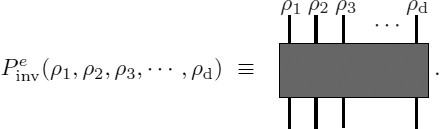(89)

We can now express the product of projection operators defining the BF quantum amplitudes in Eq. (88) in an entirely graphical way. Let us show this in 2, 3 and 4 dimensions. The generalization to arbitrary dimensions is obvious.

According to Figure (6) in two dimensions edges e ∈ Δ* are shared by two faces. This means that there are two representation matrices associated to the group element g e and hence two wires labeled by two, in principle different, ρ f s. The endpoint of the open wires are connected to the neighboring vertices and form closed loops around each face (due to the trace in (86)). There is a cable with two wires on each e ∈ Δ*. The BF amplitude is obtained by plugging together all the cables in the way dictated by the dual 2-complex Δ*. In two dimensions the result is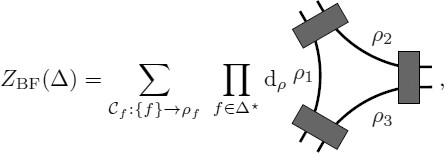(90)

where it is understood that the open ends of the wires are connected to neighboring vertices according to the combinatorial structure provided by the cellular 2-complex Δ*. In other words, the graph on the right-hand side of the previous equation is just the basic building block of a closed cable-wire diagram involving the whole complex Δ.

The construction is now obvious in three dimensions. The only difference is that now three faces share each edge and hence cables have three wires labeled by three different representations. The wires are connected according to the structure provided by the dual two complex Δ* (see Figure 7) so that a closed wire-loop is obtained for each face f ∈ Δ*. The result is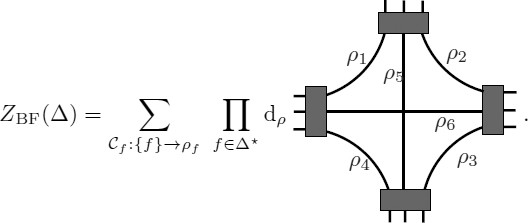(91)

At each vertex we have six wires corresponding to the six faces f ∈ Δ* sharing a vertex v ∈ Δ*. As in the 2D case, the cable diagram on the right is only a piece of a global diagram embracing the whole complex Δ.

Finally, in four dimensions the construction follows the same lines. Now edges are shared by four faces; each cable has four wires. The cable-wire diagram giving the BF amplitude is dictated by the combinatorics of the dual two complex Δ*. From Figure 8 we get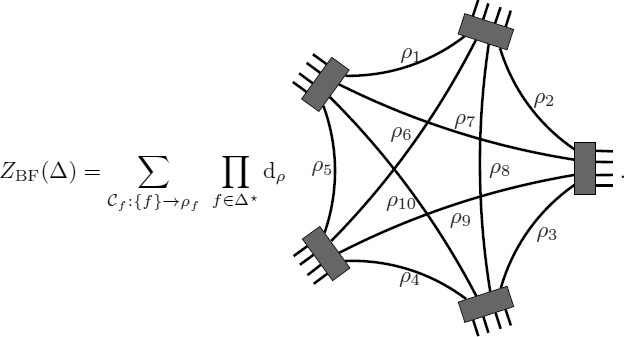(92)

The 10 wires corresponding to the 10 faces f ∈ Δ* sharing a vertex v ∈ Δ* are connected to the neighboring vertices through the 5 cables (representing the projectors in (88) and Figure 89) associated to the 5 edges e ∈ Δ* sharing the vertex v ∈ Δ*.

### Special cases

We end this section with some simple examples of BF theory that will be useful in following applications.

#### SU(2) BF theory in 2D: the simplest topological model

The amplitude of BF theory in two dimensions can be entirely worked out due to the simplicity of the projectors $$P_{{\rm{inv}}}^e$$ in this case. For concreteness, we take the special case G = SU(2), as this structure group will play an important case in the gravity context, while the techniques used here will provide a nice warming-up exercise for what follows. Irreducible representations of SU(2) are labeled by spins (half-integers): from now on we set ρ f = j f ∈ ℕ/2. In two dimensions, the relevant projectors are $$P_{{\rm{inv}}}^e:{j_f} \otimes {j_{f{\prime}}} \rightarrow {\rm{Inv}}[{j_f} \otimes {j_{f{\prime}}}]$$, which in turn are non-trivial if and only if $${j_f} = j_{{f{\prime}}}^{\ast}$$. In our graphical notation the projector is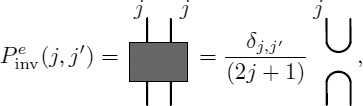(93)

where the lines on the right-hand side denote the identity representation matrices in the representation j. Replacing this in the expression of the BF amplitude given in Eq. (90), one gets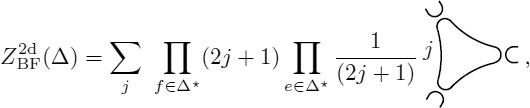(94)

where the sum over j f in (90) has collapsed to a single sum as according to (93). Spin-foam amplitudes vanish unless all the spins assigned to faces are equal, i.e., j f = j for all f ∈ Δ*. In addition to a factor d j = (2j + 1) for each face f ∈ Δ* one gets a factor 1/(2j + 1) for each edge e ∈ Δ* from the projectors (see 93). Finally, for each vertex v ∈ Δ*, one obtains a factor (2j + 1) coming from the remaining closed loop that is left over once (93) is used in all the edges associated with v (see (94)). Finally, if we denote N f , N e , and N v the number of faces, the number of edges, and the number of vertices in Δ*, respectively, then it follows that the BF partition function becomes

$$Z_{{\rm{BF}}}^{2{\rm{d}}}(\Delta) = \sum\limits_j {{{(2j + 1)}^{{N_f} - {N_e} + {N_v}}}} = \sum\limits_j {{{(2j + 1)}^\chi},}$$
(95)

where χ is the Euler character of the two-dimensional manifold $${\mathcal M}$$. In this simple two dimensional example one discovers explicitly two important properties of the partition function (and quantum amplitudes) of BF theory: first, quantum amplitudes are independent of the regulating triangulation used in their definition, and second, their discretization-independent value depends only on global properties of the spacetime-defining topological invariants of the manifold $${\mathcal M}$$. One can genuinely write

$$Z_{{\rm{BF}}}^{2{\rm{d}}}({\mathcal M}) = Z_{{\rm{BF}}}^{2{\rm{d}}}(\Delta){.}$$
(96)
##### Extra remarks on two-dimensional BF theory

Two-dimensional BF theory has been used as the basic theory in an attempt to dehne a manifold-independent model of QFT in . It is also related to gravity in two dimensions in two ways: on the one hand it is equivalent to the Jackiw-Teitelboim model [232, 351]; on the other hand, it is related to usual 2D gravity via constraints in a way similar to the one exploited in four dimensions (see Section 7). The first relationship has been used in the canonical quantization of the Jackiw-Teitelboim model in . The second relationship has been explored in .

#### SU(2) BF theory and 3D Riemannian gravity

In three dimensions, edges e ∈ Δ* are shared by three faces and thus the relevant projector is represented by a cable with three wires. In the case G = SU(2), the singlet component Inv[J1j2j3] is one dimensional when it is non-trivial. Therefore, we can write the projector as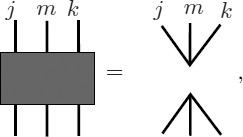(97)

where the three valent open graphs on the right-hand side represent the unique normalized invariant vector in jmk. Now we can play the same game as in the previous example. Replacing the expression of the projectors in Eq. (91) we get(98)

Hence, the amplitude can be written as a sum over spins j f associated to faces of a product of face and vertex amplitudes, namely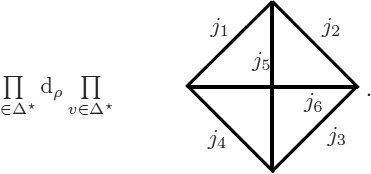(99)

The vertex amplitude is a 6j-symbol and depends on six spins labeling the corresponding faces associated with a vertex.

##### Extra remarks on three dimensional BF theory

Three dimensional BF theory and the spin-foam quantization presented above is intimately related to classical and quantum gravity in three dimensions (for a classic reference see ). We will discuss this relationship in detail in Part IV. The state sum as presented above matches the quantum amplitudes first proposed by Ponzano and Regge in the 1960s based on their discovery of the asymptotic expressions of the 6j symbols  and is often referred to as the Ponzano-Regge model. Divergences in the above formal expression require regularization. We will see in Part IV that natural regularizations are available and that the model is well defined [67, 281, 186]. For a detailed study of the divergence structure of the model see [100, 101, 102, 103]. The quantum deformed version of the above amplitudes lead to the Turaev-Viro model , which is expected to correspond to the quantization of three-dimensional Riemannian gravity in the presence of a non-vanishing positive cosmological constant. For the definition of observables in the latter context, as well as in the four-dimensional analog see .

The topological character of BF theory can be preserved by the coupling of the theory with topological defects that play the role of point particles. In the spin-foam literature, this has been considered form the canonical perspective in [279, 280] and from the covariant perspective extensively by Freidel and Louapre . These theories have been shown by Freidel and Livine to be dual, in a suitable sense, to certain non-commutative fields theories in three dimensions [185, 184].

Concerning coupling BF theory with non-topological matter, see [172, 155] for the case of fermionic matter, and  for gauge fields. A more radical perspective for the definition of matter in 3D gravity is taken in . For three-dimensional supersymmetric BF theory models, see [250, 36].

Recursion relations for the 6j vertex amplitudes have been investigated in [98, 157]. They provide a tool for studying dynamics in spin foams of 3D gravity and might be useful in higher dimensions .

#### SU(2) BF theory: The Ooguri model

In four dimensions the structure is essentially the same. Now edges are shared by four representations. In the case of G = SU(2), the vector space Inv[j1j2j3j4] has a dimension that is generically greater than one. Consequently, if one wants to write the BF quantum partition function as a sum over representations of products of faces and vertex amplitudes (the spin-foam representation) one has to express the projectors in terms of a basis of invariant vectors in j1j2j3j4. Graphically, this can be done as follows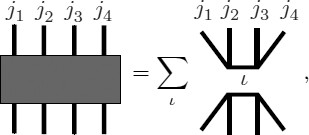(100)

where the four-valent diagrams on the right-hand side represent elements of an orthonormal basis of invariant vectors in j1j2j3j4 and its dual, which are labeled by a half integer ι. As in the previous lower dimensional cases, we replace the previous equation in expression (92) to obtain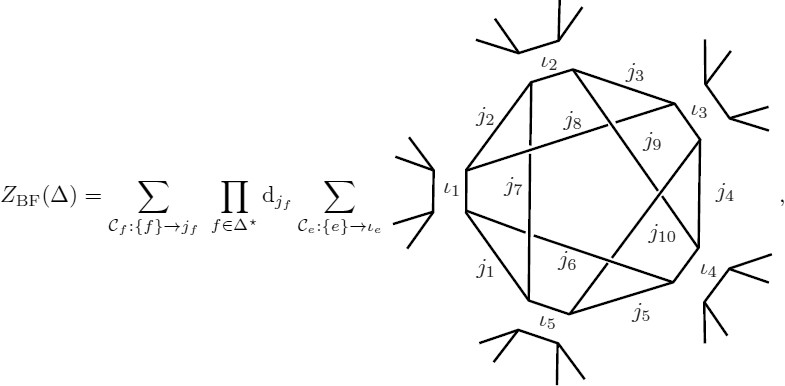(101)

which can be re-written in the spin-foam representation as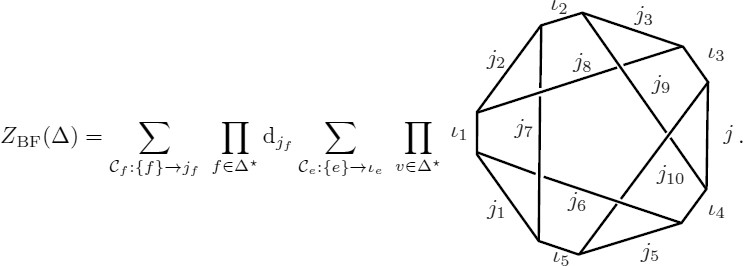(102)

The vertex amplitude in the previous expression is often called a 15j-symbol.

#### SU(2)×SU(2) BF theory: a starting point for 4D Riemannian gravity

For completeness we now present the BF quantum amplitudes in the case G = SU(2) × SU(2). This special case is of fundamental importance in the construction of the gravity models presented in Sections 7 and 8. The construction mimics that of Section 6.1.3 in all details. In fact, the product form of the structure group implies the simple relationship ZBF(SU(2) × SU(2)) = ZBF(SU(2))2. Nevertheless, it is important for us to present this example in explicit form as it will provide the graphical notation that is needed to introduce the gravity models in a simple manner. As in the previous cases, the spin-foam representation of the BF partition function follows from expressing the projectors in (92) in the orthonormal basis of intertwiners. From the previous example and the product form of the structure group we have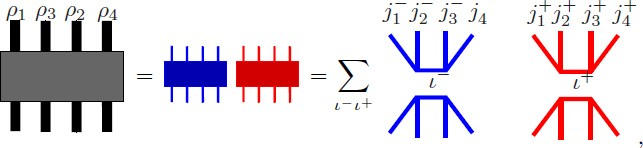(103)

where $${\rho _f} = j_f^{-} \otimes j_f^{+}$$, and $$j_f^ \pm$$ and ± are half integers labeling left and right representations of SU(2) that defined the irreducible unitary representations of G = SU(2) × SU(2). Accordingly, when replacing the previous expression in (92) one gets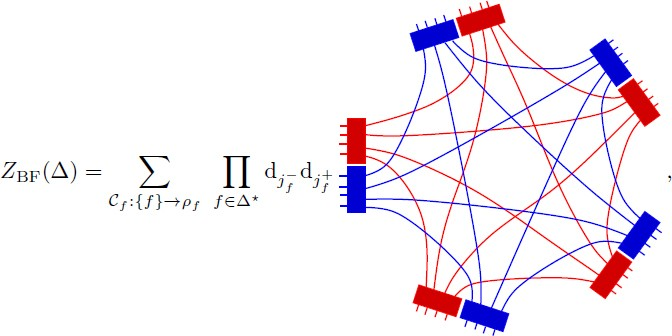(104)

and equivalently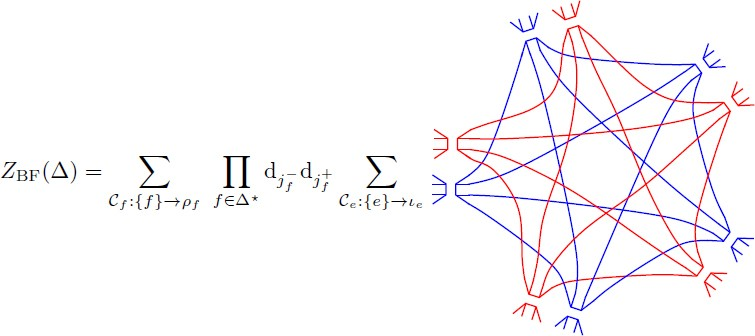(105)

from which we finally obtain the spin-foam representation of the SU(2) × SU(2) partition function as a product of two SU(2) amplitudes, namely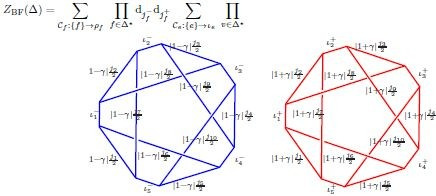(106)
##### Extra remarks on four-dimensional BF theory

The state sum (86) is generically divergent (due to the gauge freedom analogous to (78)). A regularized version defined in terms of SU q (2) × SU q (2) was introduced by Crane and Yetter [130, 129]. As in three dimensions, if an appropriate regularization of bubble divergences is provided, (86) is topologically invariant and the spin-foam path integral is discretization independent.

As in the three-dimensional case, BF theory in any dimensions can be coupled to topological defects . In the four-dimensional case, defects are string-like  and can carry extra degrees of freedom such as topological Yang-Mills fields . The possibility that quantum gravity could be defined directly from these simple kinds of topological theories has also been considered outside spin foams  (for which the UV problem described in the introduction is absent); this is attractive and should, in my view, be considered further.

It is also possible to introduce one-dimensional particles in four-dimensional BF theory and gravity as shown in .

### The coherent-states representation

In this section we introduce the coherent-state representation of the SU(2) and Spin(4) path integral of BF theory. This will be particularly important for the models defined by Freidel and Krasnov in , which we will address in Section 9, as well as in the semiclassical analysis of the new models reported in Section 13. The relevance of such representation for spin foams was first emphasized by Livine and Speziale in .

#### Coherent states

Coherent states associated with the representation theory of a compact group have been studied by Thiemann and collaborators [357, 355, 342, 360, 361, 362, 358, 50, 51, 177]; see also . Their importance for the new spin-foam models was put forward by Livine and Speziale in , where the emphasis is put on coherent states of intertwiners or the quantum tetrahedron (see also ). Here we follow the presentation of .

In order to built coherent states for Spin(4), we start by introducing them in the case of SU(2). Starting from the representation space ℋj of dimension d j ≡ 2j + 1, one can write the resolution of the identity in terms of the canonical orthonormal basis |j, m⟩ as

$$1_j=\sum\limits_m\vert j,m\rangle\langle j,m\vert{.}$$
(107)

where −jmj. There exists an over-complete basis ∣j, g⟩ ∈ ℋj labeled by gSU(2) such that

$$1_j={\rm{d}}_j\int\limits_{{\rm{SU}}(2)}dg\vert j,g\rangle\langle j,g\vert{.}$$
(108)

The states ∣j, g⟩ ∈ ℋj are SU(2) coherent states defined by the action of the group on maximum weight states |j,j⟩ (themselves coherent), namely

$$\vert j,g\rangle\equiv g\vert j,j\rangle\equiv\sum\limits_{m}\vert j,m\rangle D_{mj}^j(g),$$
(109)

where $$D_{mj}^j(g)$$ are the matrix elements of the unitary representations in the |j, m⟩ (Wigner matrices). Eq. (108) follows from the orthonormality of unitary representation matrix elements, namely

$${{\rm{d}}_j}\int\nolimits_{{{\rm{SU}}(2)}} dg{\vert}j,g\rangle \langle j,g{\vert}, = {{\rm{d}}_j}\sum\limits_{mm{\prime}} {{\vert}j,m\rangle \langle j,m{\prime}{\vert}\int\nolimits_{{{\rm{SU}}(2)}} \,dg\,D_{mj}^j(g)\overline {D_{m{\prime}j}^j(g)} = \sum\limits_m {{\vert}j,m\rangle \langle j,m{\vert},}}$$
(110)

where in the last equality we have used the orthonormality of the matrix elements graphically represented in (93). The decomposition of the identity (108) can be expressed as an integral on the two-sphere of directions S2 = SU(2)/U(1) by noticing that $$D_{mj}^j(g)$$ and $$D_{mj}^j(gh)$$ differ only by a phase for any group element h from a suitable U(1) ⊂ SU(2). Thus, one has

$${1_j} = {{\rm{d}}_j}\int\nolimits_{{{S^2}}} dn{\vert}j,n\rangle \langle j,n{\vert},$$
(111)

where nS2 is integrated with the invariant measure of the sphere. The states |j,n⟩ form (an over-complete) basis in ℋj. SU(2) coherent states have the usual semiclassical properties. Indeed, if one considers the operators Ji generators of su(2) one has

$$\langle j,n{\vert}{\hat J^i}{\vert}j,n\rangle = j{n^i},$$
(112)

where ni is the corresponding three-dimensional unit vector for nS2. The fluctuations of $${\hat J^2}$$ are also minimal with ΔJ2 = ℏ2j, where we have restored ℏ for clarity. The fluctuations go to zero in the limit ℏ → 0 and j → ∞ while ℏj is kept constant. This kind of limit will often be used as a notion of the semiclassical limit in spin foams. The state |j,n⟩ is a semiclassical state describing a vector in ℝ3 of length j and of direction n. It is convenient to introduce the following graphical notation for Eq. (111)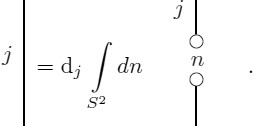(113)

Finally, an important property of SU(2) coherent states stemming from the fact that

$$\left\vert j,j\right\rangle = \left\vert {{1 \over 2}},{{1 \over 2}}\right\rangle \left\vert {{1 \over 2}},{{1 \over 2}}\right\rangle \cdots \left\vert{{1 \over 2}},{{1 \over 2}}\right\rangle \equiv \left\vert{{1 \over 2}},{{1 \over 2}}\right\rangle ^{\otimes 2j}$$

is that

$$\left\vert {j,n} \right\rangle = {\left\vert {{1 \over 2},n} \right\rangle ^{\otimes 2j}}{.}$$
(114)

The above property will be of key importance in constructing effective discrete actions for spin-foam models. In particular, it will play a central role in the study of the semiclassical limit of the EPRL and FK models studied in Sections 7, 8, and 9. In the following subsection we provide an example for Spin(4) BF theory.

#### Spin(4) BF theory: amplitudes in the coherent state basis

Here we study the coherent states representation of the path integral for Spin(4) BF theory. The construction presented here can be extended to more general cases. The present case is however of particular importance for the study of gravity models presented in Sections 7, 8, and 9. With the introductions of coherent states one achieved the most difficult part of the work. In order to express the Spin(4) BF amplitude in the coherent state representation one simply inserts a resolution of the identity in the form (111) on each and every wire connecting neighboring vertices in the expression (104) for the BF amplitudes. The result is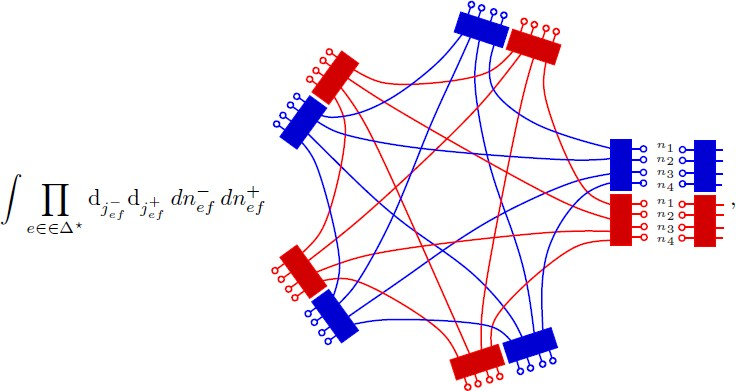(115)

where we have explicitly written the n±S2 integration variables only on a single cable. One observes that there is one n ± S2 per each wire coming out at an edge e ∈ Δ*; as wires are in one-to-one correspondence with faces f ∈ Δ* the integration variables $$n_{ef}^ \pm \in {S^2}$$ are labeled by an edge and face subindex. In order to get an expression of the BF path integral in terms of an affective action we restore at this stage the explicit group integrations represented by the boxes in the previous equation. One gets,

$$\begin{array}{*{20}c} {{Z_{{\rm{BF}}}}(\Delta) = \sum\limits_{{{\mathcal C}_f}:\{f\} \rightarrow {\rho _f}} {\prod\limits_{f \in \Delta ^{\ast}} {{{\rm{d}}_{j_f^ -}}{{\rm{d}}_{j_f^ +}}} \int {\prod\limits_{e \in \Delta ^{\ast}} {{{\rm{d}}_{j_{ef}^ -}}{{\rm{d}}_{j_{ef}^ +}}}} dn_{ef}^ - dn_{ef}^ +} \quad \quad \quad \quad \quad \quad \quad \quad \quad \quad \quad \quad \quad \quad \quad} \\ {\prod\limits_{v \in \Delta ^{\ast}} {\prod\limits_{e,e{\prime} \in v} {dg_{ev}^ - dg_{ev}^ +} {{(\langle n_{ef}^ - {\vert}({g^ -})_{ev}^{- 1}g_{e{\prime}v}^ - {\vert}n_{e{\prime}f}^ - \rangle)}^{2j_f^ -}}{{(\langle n_{ef}^ + {\vert}({g^ +})_{ev}^{- 1}g_{e{\prime}v}^ + {\vert}n_{e{\prime}f}^ + \rangle)}^{2j_f^ +}},}} \\ \end{array}$$
(116)

where we have used the coherent states property (114), and |n±⟩ is a simplified notation for $${\vert_{{1 \over 2}}},{n^ \pm}\rangle$$. Also we denote by $$g_{e\upsilon}^ \pm$$ the group elements associated with the five boxes for each given vertex (see Eq. (115)). Finally, the previous equation can be finally written as

$${Z_{{\rm{BF}}}}(\Delta) = \sum\limits_{{{\mathcal C}_f}:\{f\} \rightarrow {\rho _f}} {\prod\limits_{f \in {\Delta ^{\ast}}} {{{\rm{d}}_{j_f^ -}}{{\rm{d}}_{j_f^ +}}} \int {\prod\limits_{e \in {\Delta ^{\ast}}} {{{\rm{d}}_{j_{ef}^ -}}{{\rm{d}}_{j_{ef}^ +}}}} dn_{ef}^ - dn_{ef}^ + dg_{ev}^ - dg_{ev}^ + \exp (S_{{j^ \pm},{{\bf{n}}^ \pm}}^d[{g^ \pm}])},$$
(117)

where the discrete action

$$S_{{j^ \pm},{{\bf{n}}^ \pm}}^d[{g^ \pm}] = \sum\limits_{v \in \Delta ^{\ast}} {S_{{j_v},{{\bf{n}}_v}}^v} [{g^ \pm}]$$
(118)

with

$$S_{j,{\bf{n}}}^v[g] = \sum\limits_{a < b = 1}^5 {2{j_{ab}}} \ln \langle {n_{ab}}\vert g_a^{- 1}{g_b}\vert {n_{ba}}\rangle,$$
(119)

and the indices a, b label the five edges of a given vertex. The previous expression is exactly equal to the form (86) of the BF amplitude. In the case of the gravity models studied in what follows, the coherent state path integral representation will be the basic tool for the study of the semiclassical limit of the models and the relationship with the Regge discrete formulation of general relativity.

## The Riemannian EPRL Model

In this section we introduce the Riemannian version of the Engle-Pereira-Rovelli-Livine (EPRL) [168, 164]. The section is organized as follows. The relevant Spin(4) representation theory is introduced in Section 7.1. In Section 7.2 we present and discuss the linear simplicity constraints — classically equivalent to the Plebanski constraints — and discuss their implementation in the quantum theory. In Section 7.3 we introduce the EPRL model of Riemannian gravity. In Section 7.4 we prove the validity of the quadratic Plebanski constraints — reducing BF theory to general relativity — directly in the spin-foam representation. In Section 7.5 we discuss a certain modification of the EPRL model. In Section 7.6 we present the coherent state representation of the EPRL model, which is essential for the semiclassical analysis of Section 13. The material of this section will also allow us to describe the construction of the closely related (although derived from a different logic) Riemannian FK constructed in . The idea that linear simplicity constraints are more convenient for dealing with the constraints that reduce BF theory to gravity was pointed out by Freidel and Krasnov in this last reference. We have shown in Part II that they arise directly from the canonical analysis of the Plebanski formulation of gravity.

### Representation theory of Spin(4) and the canonical basis

In order to introduce the definition of the EPRL model, we need to briefly review the representation theory of Spin(4). The group Spin(4) = SU(2) × SU(2) with Lie algebra spin(4) = su(2) ⊕ su(2) can explicitly be described in terms of generators $$J_ \pm ^i$$ such that

$$[J_ \pm ^i,J_ \pm ^j] = {\epsilon ^{ij}}_kJ_ \pm ^k{.}$$
(120)

Unitary irreducible representations $${\mathscr{H}_{{j^ +}}}_{,{j^ -}}$$ of Spin(4) are given by the product of unitary irreducible representations of SU(2), i.e., they are labeled by two half-integers j±. A standard basis in $${\mathscr{H}_{{j^ +}}}_{,{j^ -}}$$ is given by vectors |j+, j, m+, m⟩ of eigenstates of the Casimirs $${C_1} = J_ + ^2 + J_ - ^2$$ and $${C_2} = J_ + ^2 - J_ - ^2$$ and the components $$J_ + ^3$$ and $$J_ - ^3$$, explicitly

$$\begin{array}{*{20}c} {{C_1}{\vert}{j^ +},{j^ -},{m^ +},{m^ -}\rangle = ({j_ +}({j_ +} + 1) + {j_ -}({j_ -} + 1)){\vert}{j^ +},{j^ -},{m^ +},{m^ -}\rangle,} \\ {{C_2}{\vert}{j^ +},{j^ -},{m^ +},{m^ -}\rangle = ({j_ +}({j_ +} + 1) + {j_ -}({j_ -} + 1)){\vert}{j^ +},{j^ -},{m^ +},{m^ -}\rangle,} \\ {J_ \pm ^3{\vert}{j^ +},{j^ -},{m^ +},{m^ -}\rangle = {m^ \pm}{\vert}{j^ +},{j^ -},{m^ +},{m^ -}\rangle{.}\quad \quad \quad \quad \quad \quad \quad \,\,\,\,\,}\\ \end{array}$$
(121)

The definition of the EPRL model requires the introduction of an (arbitrary) subgroup SU(2) ⊂ Spin(4). This subgroup will be shown, a posteriori, to be the internal gauge group of the gravitational phase space in connection variables (appearing in the classical canonical study of Section 3.2 and leading to the quantization of Section 4). In the quantum theory, the representation theory of this SU(2) subgroup will be important. This importance will soon emerge as apparent from the imposition of the constraints that dehne the EPRL as well as the FK model of Section 9.

The link between the unitary representations of spin(4) and those of su(2) comes from the fact that the former can be expressed as a direct sum of the latter according to

$${\mathscr H}_{j^+,j^-}=\overset{j^++j^-}{{\underset{j=\vert j^+-j^-\vert}{\bigoplus}}}{\mathscr H}_j{.}$$
(122)

As the unitary irreducible representations of the subgroup SU(2) ∈ Spin(4) are essential in understanding the link of the EPRL model and the operator canonical formulation of LQG, it will be convenient to express the action of the generators of the Lie algebra spin(4) in a basis adapted to the above equation. In order to do this, we first notice that the Lie algebra (120) can be equivalently characterized in terms of the generators of a rotation subgroup Li and the remaining “boost” generators Ki as follows

$$\begin{array}{*{20}c} {[{L_3},{L_ \pm}] = \pm {L_ \pm},\quad [{L_ +},{L_ -}] = 2\,{L_3},\quad \,\,\,\,\,\,\,\quad \quad \quad \quad \quad \quad \quad \,\,} \\ {[{L_ +},{K_ +}] = [{L_ -},{K_ -}] = [{L_3},{K_3}] = 0,\,\quad \quad \quad \quad \quad \quad \quad \quad \quad} \\ {[{K_3},{L_ \pm}] = \pm \,\,{K_ \pm},\quad [{L_ \pm},{K_ \mp}] = \pm 2\,\,{K_3},\quad \quad [{L_3},{K_ \pm}] = \pm \,{K_ \pm},} \\ {[{K_3},{K_ \pm}] = \pm \,{L_ \pm},\quad [{K_ +},{K_ -}] = 2\,\,{L_3},\quad \quad \,\,\quad \quad \quad \quad \quad \quad \,\,} \\ \end{array}$$
(123)

where K± = K1 ± iK2 and L± = L1±iL2, respectively. The action of the previous generators in the basis |j+, j, j, m⟩ can be shown to be

$$\begin{array}{*{20}c} {{L^3}|{j^ +},\,{j^ -},\,j,\,m\rangle = m|{j^ +},\,{j^ -},\,j,\,m\rangle ,\quad \quad \quad \quad \quad \quad \quad \quad \quad \quad \quad \quad \quad \quad \quad \quad \quad \quad \quad \quad \quad} \\ {{L^ +}|{j^ +},\,{j^ -},\,j,\,m\rangle = \sqrt {(j + m + 1)\,(j - m)} |{j^ +},\,{j^ -},\,j,\,m + 1\rangle ,\quad \quad \quad \quad \quad \quad \quad \quad \quad \quad \quad \quad \quad} \\ {{L^ -}|{j^ +},\,{j^ -},\,j,\,m\rangle = \sqrt {(j + m)\,(j - m + 1)} |{j^ +},\,{j^ -},\,j,\,m - 1\rangle ,\quad \quad \quad \quad \quad \quad \quad \quad \quad \quad \quad \quad \,\,\,\,} \\ {{K^3}|{j^ +},\,{j^ -},\,j,\,m\rangle = \quad \quad \quad \quad \quad \quad \quad \quad \quad \quad \quad \quad \quad \quad \quad \quad \quad \quad \quad \quad \quad \quad \quad \quad \quad \quad \,\,\,\,\,} \\ {{\alpha _j}\sqrt {{j^2} - {m^2}} |{j^ +},\,{j^ -},\,j - 1,\,m\rangle + {\gamma _j}m|{j^ +},\,{j^ -},\,j,\,m\rangle - {\alpha _{j + 1}}\sqrt {{{(j + 1)}^2} - {m^2}} |{j^ +},\,{j^ -},\,j + 1,\,m\rangle ,\quad} \\ {{K^ +}|{j^ +},\,{j^ -},\,j,\,m\rangle = {\alpha _j}\sqrt {(j - m)\,(j - m - 1)} |{j^ +},\,{j^ -},\,j - 1,\,m + 1\rangle \quad \quad \quad \quad \quad \quad \quad \quad \quad \quad \,\,\,} \\ {+ {\gamma _j}\sqrt {(j - m)\,(j + m + 1)} |{j^ +},\,{j^ -},\,j,\,m + 1\rangle \quad \quad \quad \quad \quad \quad \quad \quad \quad \quad \quad \quad \quad \quad \quad \quad \quad \quad \,\,\,} \\ {+ {\alpha _{j + 1}}\sqrt {(j + m + 1)\,(j + m + 2)} |{j^ +},\,{j^ -},\,j + 1,\,m + 1\rangle ,\quad \quad \quad \quad \quad \quad \quad \quad \quad \quad \quad \quad \quad \quad \quad \,} \\ {{K^ -}|{j^ +},\,{j^ -},\,j,\,m\rangle = - {\alpha _j}\sqrt {(j + m)\,(j + m - 1)} |{j^ +},\,{j^ -},\,j - 1,\,m - 1\rangle \quad \quad \quad \quad \quad \quad \quad \quad \quad \quad} \\ {+ {\gamma _j}\sqrt {(j + m)\,(j - m + 1)} |{j^ +},\,{j^ -},\,j,\,m - 1\rangle \quad \quad \quad \quad \quad \quad \quad \quad \quad \quad \quad \quad \quad \quad \quad \quad \quad \quad \quad} \\ {- {\alpha _{j + 1}}\sqrt {(j - m + 1)\,(j - m + 2)} |{j^ +},\,{j^ -},\,j + 1,\,m - 1\rangle ,\quad \quad \quad \quad \quad \quad \quad \quad \quad \quad \quad \quad \quad \quad \quad} \\ \end{array}$$
(124)

where

$${\gamma _j} = {{{j^ +}({j^ +} + 1) - {j^ -}({j^ -} + 1)} \over {j(j + 1)}}\,\,\,\,{\rm{and}}\,\,\,{\alpha _j} = \sqrt {{{({j^2} - {{({j^ +} + {j^ -} + 1)}^2})({j^2} - {{({j^ +} - {j^ -})}^2})} \over {{j^2}(4{j^2} - 1)}}} {.}$$
(125)

The previous equations will be important in what follows: they will allow for the characterization of the solutions of the quantum simplicity constraints in a direct manner. This concludes the review of the representation theory that is necessary for the definition of the EPRL model.

### The linear simplicity constraints

As first emphasized in , the quadratic simplicity constraints (25) — and more precisely in their dual version presented below (154) — are equivalent in the discrete setting to the linear constraint on each face of a given tetrahedron

$$D_f^i = L_f^i - {1 \over \gamma}K_f^i \approx 0,$$
(126)

where the label f makes reference to a face f ∈ Δ*, and where (very importantly) the subgroup SU(2) ⊂ Spin(4) that is necessary for the definition of the above constraints is chosen arbitrarily at each tetrahedron, equivalent on each edge e ∈ Δ*. Such choice of the rotation subgroup is the precise analog of the time gauge in the canonical analysis of Section 3. Moreover, the linear simplicity constraint above is the simplicial version of the canonical primary constraint (37). The EPRL model is defined by imposing the previous constraints as operator equations on the Hilbert spaces defined by the unitary irreducible representations of Spin(4) that take part in the state-sum of BF theory. We will show in Section 7.4 that the models constructed on the requirement of a suitable imposition of the linear constraints (126) satisfy the usual quadratic Plebanski constraints in the path-integral formulation (up to quantum corrections, which are neglected in the usual semiclassical limit).

It will be useful in what follows to write the above constraint in its equivalent form

$$D_f^i = (1 - \gamma)J_f^{+ i} - (1 + \gamma)J_f^{- i} \approx 0,$$
(127)

From the commutation relations (120) of Section 7.1 we can easily compute the commutator of the previous tetrahedron constraints and conclude that in fact it does not close, namely

$$\begin{array}{*{20}c} {[D_f^i,D_{f{\prime}}^i] = {\delta_{ff{\prime}}}{\epsilon ^{ij}}_k\left[ {(1 + {1 \over {{\gamma ^2}}})L_f^k - {2 \over \gamma}K_e^k} \right]\quad \quad \quad \quad \,\,\,\,} \\ {= 2{\delta _{ee{\prime}}}{\epsilon ^{ij}}_k{D^k} + {\delta _{ee{\prime}}}{{1 - {\gamma ^2}} \over {{\gamma ^2}}}{\epsilon ^{ij}}_kL_f^k.} \\ \end{array}$$
(128)

The previous commutation relations imply that the constraint algebra is not closed and cannot therefore be imposed as operator equations on the states summed over in the BF partition function in general. There are two interesting exceptions to the previous statement:

1. 1.

The first is to take γ = ±1, in which case the constraint (186) can be imposed strongly. More precisely, for the plus sign it reduces to the condition $$J_{f +}^i = 0$$ (or equivalently for the other sign $$J_{f -}^i = 0$$), which amounts to setting all the left quantum numbers in the BF state-sum for the group Spin(4) to zero. It is immediate to see that if we impose such restriction on the Spin(4) BF amplitude (106) it simply reduces to the amplitude (102) defining SU(2) BF theory. Thus, this possibility does not lead to an acceptable model for quantum gravity in 4D.

2. 2.

The second possibility is to work in the sector where $$L_f^i = 0$$. We will show later that this corresponds to the famous Barret-Crane model  with the various limitations discussed in Section 10.

The EPRL model is obtained by restricting the representations appearing in the expression of the BF partition function so that at each tetrahedron the linear constraints (186) are satisfied the strongest possible way that is compatible with the uncertainties relations stemming from (187). In addition, one would add the requirement that the state-space of a tetrahedra is compatible with the state-space of the analogous excitation in the canonical context of LQG so that arbitrary states in the kinematical state of LQG have non-trivial amplitudes in the model.

### On the weak imposition of the linear simplicity constraints

We now discuss the weak imposition of the linear simplicity constraints in the quantum framework. There are essentially three views in the literature: two of them, discussed below, directly concerns the way the EPRL model has usually been introduced. The third possibility is the semiclassical view based on the coherent-state representation leading to the FK model (see Section 9).

#### Riemannian model: The Gupta-Bleuler criterion

Due to the fact that the constraints do not form a closed (first class) algebra in the generic case, one needs to devise a weaker sense in which they are to be imposed. One possibility is to consider the Gupta-Bleuler criterion consisting in selecting a suitable class of states for which the matrix elements on $$D_f^i$$ vanish. In this respect, one notices that if we choose the subspace $${{\mathscr{H}}_j} \subset {{\mathscr{H}}_{{j^ +},{j^ -}}}$$ one has

$$\begin{array}{*{20}c} {\langle {j^ +},{j^ -},j,q{\vert}D_f^3{\vert}{j^ +},{j^ -},j,m\rangle = {\delta _{q,m}}m(1 - {{{\gamma _j}} \over \gamma}),\quad \quad \quad \quad \quad \quad \quad \,\,\,\,\,} \\ {\langle {j^ +},{j^ -},j,q{\vert}D_f^ \pm {\vert}{j^ +},{j^ -},j,m\rangle = {\delta _{q \pm 1,m}}\sqrt {(j \pm m + 1)(j \mp m)} \left({1 - {{{\gamma _j}} \over \gamma}} \right).} \\ \end{array}$$

One immediately observes that matrix elements of the linear constraints vanish in this subclass if one can chose

$${\gamma _j} = {{{j^ +}({j^ +} + 1) - {j^ -}({j^ -} + 1)} \over {j(j + 1)}} = \gamma {.}$$
(129)

There are two cases:

1. 1.

Case γ < 1: Following , in this case one restricts the Spin(4) representations to

$${j^ \pm} = (1 \pm \gamma){j \over 2},$$
(130)

which amounts to choosing the maximum weight component j = j+ + j in the expansion (182). Simple algebra shows that condition (188) is met. There are indeed other solutions  of the Gupta-Bleuler criterion in this case.

2. 2.

Case γ > 1: In this case  one restricts the Spin(4) representations to

$${j^ \pm} = (\gamma \pm 1){j \over 2} + {{\gamma - 1} \over 2},$$
(131)

which amounts to choosing the minimum weight component j = j+j in the expansion (182). This choice of is the solution to condition (188).

#### Riemannian model: The Master-constraint criterion

Another criterion for weak imposition can be developed by studying the spectrum of the Master constraint M f = D f ·D f . Strong imposition of the constraints $$D_f^i$$ would amount to looking for the kernel of the Master constraint M f . However, generically the positive operator associated with the Master constraint does not contain the zero eigenvalue in the spectrum due to the open nature of the constraint algebra (187). The proposal of , that we follow here, is to look for the minimum eigenvalue among spaces $${{\mathscr{H}}_j} \in {{\mathscr{H}}_{{j^ +}}}_{,{j^ -}}$$. Explicitly

$${M_f}{\vert}\psi > = {m_{{j^ \pm},j}}{\vert}\psi >,$$
(132)

where

$${m_{{j^ \pm},j}} = {(1 - \gamma)^2}{j_ +}({j_ +} + 1) + (1 + {\gamma ^2}){j_ -}({j_ -} + 1) - (1 - {\gamma ^2})[j(j + 1) - {j_ +}({j_ +} + 1) - {j_ -}({j_ -} + 1)]{.}$$

There are two cases:

1. 1.

Case γ < 1: In this case the minimum eigenvalue is obtained for

$${j^ \pm} = (1 \pm \gamma)j/2,$$
(133)

i.e., one has

$${m_{{j^ \pm},j}} \geq {m_j},$$

where m j = ℏ2(1 − γ2)j, where we have restored the explicit dependence on so that it is apparent that the selected eigenvalue vanishes in the semiclassical limit ℏ → 0 and k → ∞ with ℏk = constant.

2. 2.

Case γ > 1: In this case, the minimum eigenvalue is obtained for

$${j^ \pm} = (\gamma \pm 1)j/2,$$
(134)

for which m j = ℏ2(γ2 − 1)j, and thus vanishes in the semiclassical limit → 0 and k → ∞ with ℏk = constant.

The Master-constraint criterion will be used here as the basic justification for the definition of the EPRL model. Notice however, that the Master constraint is only invariant under the subgroup SU(2) ⊂ Spin(4) chosen at each tetrahedron. Its lack of Lorentz invariance is clear from the fact that its validity is justified from the canonical analysis in the time gauge. Nevertheless, as shown in , one can also write down a criterion (equivalent to the Master-constraint one) in a manifestly Spin(4) invariant way. This reflects the arbitrariness in the choice of the internal subgroup SU(2) ⊂ Spin(4) and implies the consistency of the time gauge. In order to get a gauge-invariant constraint, one starts from the Master constraint and uses the $$D_f^i = 0$$ classically to rewrite it entirely in terms of the Spin(4) Casimirs, namely

$$\begin{array}{*{20}c} {{M_f} = {{(1 - \gamma)}^2}J_ + ^2 + {{(1 + \gamma)}^2}J_ - ^2 - 2(1 - {\gamma ^2}){J_ +} \cdot {J_ -}} \\ {\approx (1 + {\gamma ^2}){C_2} - 2{C_{1\gamma}},\quad \quad \quad \quad \quad \quad \quad} \\ \end{array}$$
(135)

where C1 and are the two spin(4) Casimirs given in Eq. (121). The Lorentz invariant version of the Master constraint is hence

$$M_f^{LI} = (1 + {\gamma ^2}){C_2} - 2{C_1}\gamma = 0{.}$$
(136)

One can verify for the cases γ < 1 and γ > 1 the Lorentz invariant criterion is just equivalent to the Master-constraint analysis above.

#### Riemannian model: Restrictions on the Immirzi parameter

We have just shown how the states in $${{\mathscr{H}}_{{j^ +}}}_{,{j^ -}}$$ can be restricted in order to satisfy the linear simplicity constraints according to different criteria. From all these the EPRL model is defined most simply using the Master-constraint criterion. In all cases, the restriction takes the form of the selection of a subspace $${{\mathscr{H}}_j} \subset {{\mathscr{H}}_{{j^ +}}}_{,{j^ -}}$$ given by unitary irreducible representations of a subgroup SU(2) ∂ Spin(4). As we will show in what follows, this implies that the boundary states of the spin-foam models defined by the EPRL construction are in correspondence to SU(2) spin network states: the states of the kinematical Hilbert space of LQG reviewed in Part II. This is very nice as it suggests that the spin-foam amplitudes can be interpreted as dynamical transition amplitudes of the canonical quantum theory. Now, in order for this to be so, one would like arbitrary spins j ∈ ℕ/2 to be admissible in the state sum. We shall now see that this imposes restrictions on the possible values of the Immirzi parameter. However, these restrictions seem so far of little physical importance as they are a special feature of the Riemannian model that is absent in its Lorentzian relative. As above, there are two distinct cases:

1. 1.

Case γ < 1: If one wants the spin j ∈ ℕ/2 to be arbitrary, then the only possibilities are γ = 0 or γ = 1. Rational γ values would restrict the spins j to a subclass in ℕ/2. This restriction is not natural from the viewpoint of LQG. Its relevance, if any, remains mysterious at this stage.

2. 2.

Case γ > 1: In this case, γ would allow any j ∈ ℕ/2. Rational choices of γ have the same effect as in the previous case.

#### Riemannian model: Overview of the solutions of the linear simplicity constraints

We have reviewed different criteria given in the literature for the definition of the subset of quantum states that are selected in the construction of the EPRL model. Of all the criteria, the Master constraint is the most direct and clear cut. For the Riemannian model, the result is:

1. 1.

Case γ < 1:

$${j^ \pm} = (1 + \gamma)j/2,$$
(137)
2. 2.

Case γ > 1:

$${j^ \pm} = (\gamma \pm 1)j/2,$$
(138)

where j ∈ ℕ/2 is arbitrary — except from the requirement that j± ∈ ℕ/2, which depends on the value of the Immirzi parameter according to Section 7.2.2. From now on we denote the subset of admissible representation of Spin(4) as

$${{\mathscr K}_\gamma} \subset {\rm{Irrep}}(Spin(4)){.}$$
(139)

It follows from the discussion in the previous sections that states $$\Psi \in {{{\mathscr{K}}}_\gamma}$$ satisfy the constraints (186) in the following semiclassical sense:

$$(K_f^i - \gamma L_f^i)\Psi = {{\mathscr O}_{sc}},$$
(140)

where the symbol $${{{\mathscr{O}}}_{sc}}$$ (order semiclassical) denotes a quantity that vanishes in limit → 0, j → ∞ with ℏj = constant. Equivalently, one has

$$[(1 - \gamma)J_ + ^i - (1 + \gamma)J_ - ^i]\Psi = {{\mathscr O}_{sc}}{.}$$
(141)

Using the decomposition (122), the last equation can be written graphically as: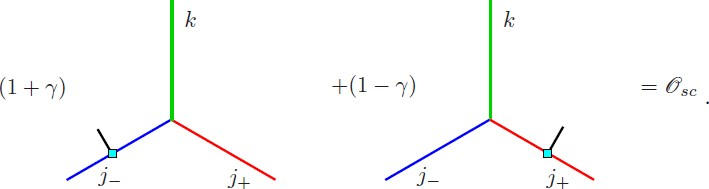(142)

The previous equation will be of great importance in the graphical calculus that will allow us to show that the linear constraint imposed here at the level of states implies the vanishing of the quadratic Plebanski constraints (25) and their fluctuations, computed in the path integral sense, in the appropriate large spin semiclassical limit.

### Presentation of the EPRL amplitude

Here we complete the definition of the EPRL models by imposing the linear constraints on the BF amplitudes constructed in Section 6. We will also show that the path-integral expectation value of the Plebanski constraints (25), as well as their fluctuations, vanish in a suitable semiclassical sense. This shows that the EPRL model can be considered as a lattice definition of quantum-gravity theory.

We start with the Riemannian model for which a straightforward graphical notation is available. The first step is the translation of Eq. (122) in terms of the graphical notation introduced in Section 6. Concretely, for γ < 1, one has $${j^ \pm} = (1 \pm \gamma)j/2 \in {{\mathscr{K}}_\gamma}$$ — as defined in (139) — becomes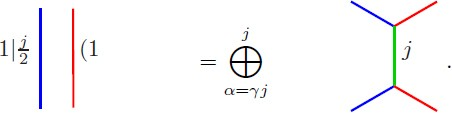(143)

For γ > 1 we have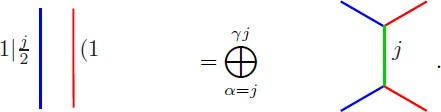(144)

The implementation of the linear constraints of Section (7.2) consist in restricting the representations ρ f of Spin(4) appearing in the state sum amplitudes of BF theory as written in Eq. (104) to the subclass $${\rho _f} \in {{\mathscr{K}}_\gamma} \subset$$, defined above, while projecting to the highest weight term in (143) for γ < 1. For γ > 1 one must take the minimum weight term in (144). The action of this projection will be denoted $${{\mathscr{Y}}_j}:{{\mathscr{H}}_{(1 + \gamma)j/2, \vert (1 - \gamma)j/2}} \rightarrow {{\mathscr{H}}_j}$$, graphically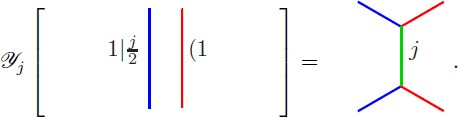(145)

Explicitly, one takes the expression of the BF partition function (88) and modifies it by replacing the projector $$P_{{\rm{inv}}}^e({\rho _1}, \cdots, {\rho _4})$$ with $${\rho _1}, \cdots {\rho _4} \in {{\mathscr{K}}_\gamma}$$ by a new object

$$P_{{\rm{EPRL}}}^e({j_1}, \cdots, {j_4}) \equiv P_{{\rm{inv}}}^e({\rho _1} \cdots {\rho _4})({{\mathscr Y}_{{j_1}}} \otimes \cdots \otimes {{\mathscr Y}_{{j_4}}})P_{{\rm{inv}}}^e({\rho _1} \cdots {\rho _4}),$$
(146)

with j1, … j4 ∈ ℕ/2 implementing the linear constraints described in Section 7.2. Graphically the modification of BF theory that produces the EPRL model corresponds to the replacement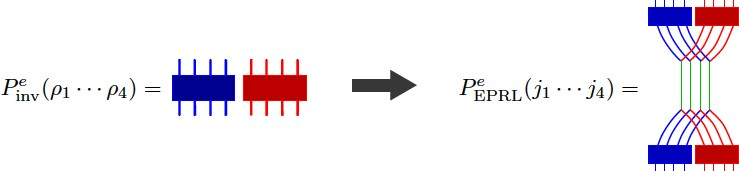(147)

on the expression (104), where we have dropped the representation labels from the figure for simplicity. We have done the operation (145) on each of the four pairs of representations. The Spin(4) integrations represented by the two boxes at the top and bottom of the previous graphical expression restore the full Spin(4) invariance as the projection (145) breaks this latter symmetry for being based on the selection of a special subgroup SU(2) ⊂ Spin(4) in its definition (see Section 7.5 for an important implication). One should simply keep in mind that green wires in the previous two equations and in what follows are labeled by arbitrary spins j (which are being summed over in the expression of the amplitude (148)), while red and blue wires are labeled by j+ = (1 + γ)j/2 and j = |1 − γ|j/2, respectively. With this (104) is modified to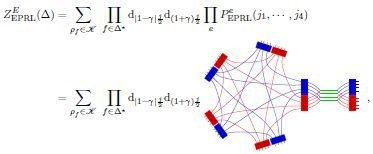(148)

The previous expression defines the EPRL model amplitude.

#### The spin-foam representation of the EPRL amplitude

Now we will work out the spin-foam representation of the EPRL amplitude, which, at this stage, will take no more effort than the derivation of the spin-foam representation for Spin(4) BF theory as we went from Eq. (104) to (106) in Section 6. The first step is given in the following equation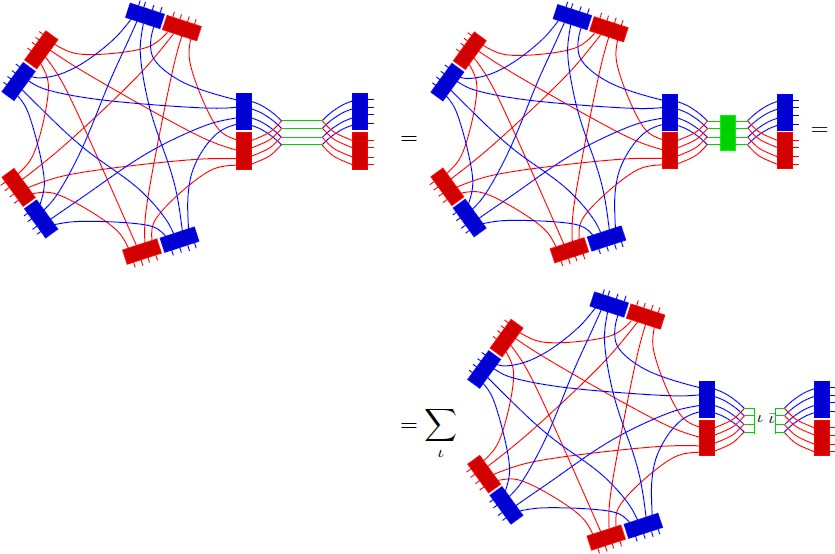(149)

which follows from the invariance of the Haar measure (84) (in the last line we have used (100)). More precisely, the integration of the subgroup SU(2) ∈ Spin(4), represented by the green box on the right, can be absorbed by suitable redefinition of the integration on the right and left copies of SU(2), represented by the red and blue boxes, respectively. With this we can already write the spin-foam representation of the EPRL model, namely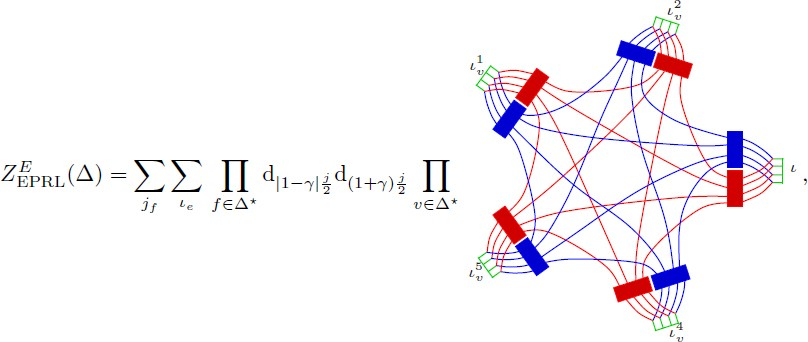(150)

where the vertex amplitude (graphically represented) depends on the 10 spins j associated to the face-wires and the 5 intertwiners associated to the five edges (tetrahedra). As in previous equations we have left the spin labels of wires implicit for notational simplicity. We can write the previous spin-foam amplitude in another form by integrating out all the projectors (boxes) explicitly. Using (100), we get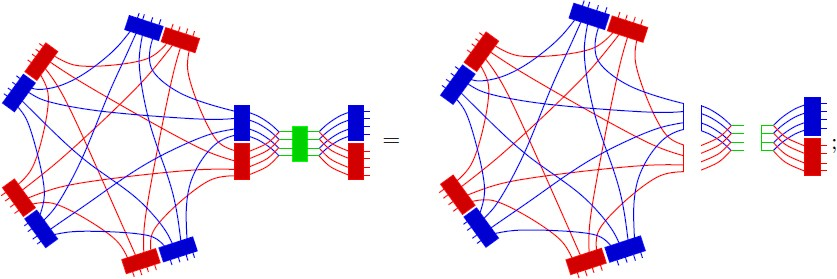(151)

replacing this in (148) we get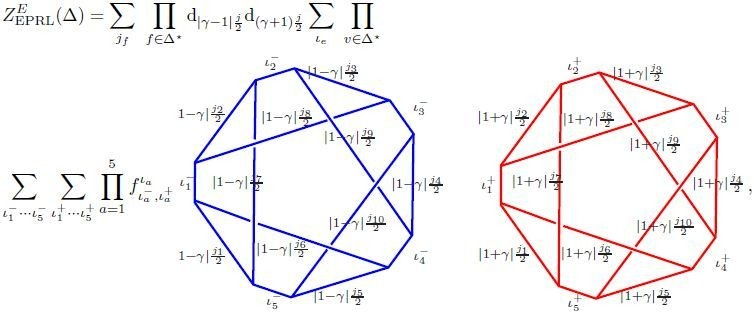(152)

where the coefficients $$f_{{\iota ^ +}{\iota ^ -}}^\iota$$ are called the fusion coefficients, which appear in their graphical form already in (151), more explicitly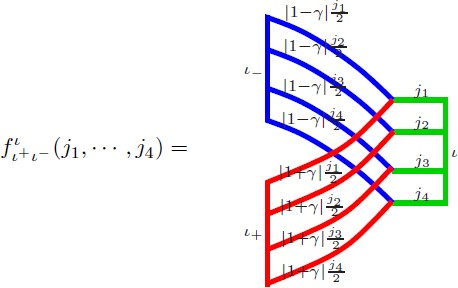(153)

The previous is the form of the EPRL model as derived in .

### Proof of validity of the Plebanski constraints

In this section, we prove that the quadratic constraints are satisfied in the sense that their path integral expectation value and fluctuation vanish in the appropriate semiclassical limit.

#### The dual version of the constraints

In this section, we rewrite the Plebanski constraints (25) in an equivalent version where spacetime indices are traded with internal Lorentz indices, namely

$${\epsilon _{IJKL}}B_{\mu \nu}^{IJ}B_{\rho \sigma}^{KL} - e\,{\epsilon _{\mu \nu \rho \sigma}} \approx 0{.}$$
(154)

The constraints in this form are more suitable for translation into the discrete formulation. More precisely, according to (81), the smooth fields $$B_{\mu \nu}^{I\,J}$$ is now associated with the discrete quantities $$B_{{\rm{triangles}}}^{I\,J}$$, or equivalently $$B_{f}^{I\,J}$$ as, we recall, faces f ∈ Δ* are in one-to-one correspondence to triangles in four dimensions. The constraints (154) are local constraints valid at every spacetime point. In the discrete setting, spacetime points are represented by four-simplexes or (more adapted to our discussion) vertices v ∈ Δ*. With all this, the constraints (154) are discretized as follows:

$${\bf{Triangle}}\,{\bf{(or}}\,{\bf{diagonal)}}\,{\bf{constraints}}:\quad \quad {\epsilon _{I\,J\,K\,L}}B_f^{I\,J}B_f^{K\,L} = 0,$$
(155)

for all fv, i.e., for each and every face of the 10 possible faces touching the vertex v.

$${\bf{Tetrahedron}}\,{\bf{constraints:}}\quad \quad {\epsilon _{I\,J\,K\,L}}B_f^{I\,J}B_{{f\prime}}^{K\,L} = 0,$$
(156)

for all f, f′v such that they are dual to triangles sharing a one-simplex, i.e., belonging to the same tetrahedron out of the five possible ones.

$$4 - {\bf{simplex}}\,{\bf{constraints}}:\quad \quad \quad {\epsilon _{I\,J\,K\,L}}B_f^{I\,J}B_{\bar f}^{K\,L} = {e_v},$$
(157)

for any pair of faces $$f,\bar f \in \upsilon$$ that are dual to triangles sharing a single point. The last constraint will require a more detailed discussion. At this point, let us point out that constraint (157) is interpreted as a definition of the four volume e v of the four-simplex. The constraint requires that such definition be consistent, i.e., the true condition is

$${\epsilon _{IJKL}}B_f^{IJ}B_{\bar f}^{IJ} = {\epsilon _{IJKL}}B_{f{\prime}}^{IJ}B_{\bar f{\prime}}^{KL} = {\epsilon _{IJKL}}B_{f^{\prime\prime}}^{IJ}B_{\bar f^{\prime\prime}}^{KL} = \cdots = {e_v}$$
(158)

for all five different possible pairs of f and $$\bar f$$ in a four simplex, and where we assume the pairs $$f - \bar f$$ are ordered in agreement with the orientation of the complex Δ*.

#### The path integral expectation value of the Plebanski constraints

Here we prove that the Plebanski constraints are satisfied by the EPRL amplitudes in the path integral expectation value sense.

##### The triangle constraints:

We start from the simplest case: the triangle (or diagonal) constraints (155). We choose a face fv (dual to a triangle) in the cable-wire-diagram of Eq. (148). This amounts to choosing a pair of wires (right and left representations) connecting two nodes in the vertex cable wire diagram. The two nodes are dual to the two tetrahedra — in the four simplex dual to the vertex — sharing the chosen triangle. From (29) one can show that

$${\epsilon _{IJKL}}B_f^{IJ}B_f^{KL} \propto {(1 + \gamma)^2}J_f^ - \cdot J_f^ - - {(1 - \gamma)^2}J_f^ + \cdot J_f^ +,$$
(159)

where $$J_f^ \pm$$ denotes the self-dual and anti-self-dual parts of $$\Pi _f^{I\,J}$$. The path integral expectation value of the triangle constraint is then(160)

where the double graspings on the anti-self-dual (blue) wire and the self-dual (red) wire represent the action of the Casimirs $$J_f^ - \cdot J_f^ -$$ and $$J_f^ + \cdot J_f^ +$$ on the cable-wire diagram of the corresponding vertex. Direct evaluation shows that the previous diagram is proportional to $${\hbar ^2}{j_f}$$, which vanishes in the semiclassical limit ℏ → 0, j → ∞ with ℏj = constant. We use the notation already adopted in (140) and call such a quantity $${{\mathscr{O}}_{sc}}$$. This concludes the proof that the triangle Plebanski constraints are satisfied in the semiclassical sense.

##### The tetrahedra constraints:

The proof of the validity of the tetrahedra constraints (156). In this case we also have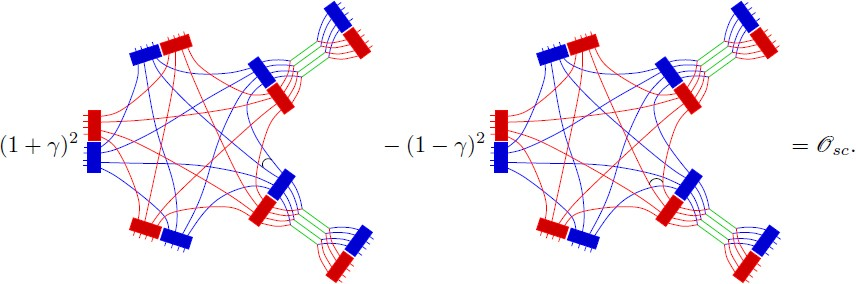(161)

where we have chosen an arbitrary pair of faces. In order to prove this, let us develop the term on the right. The result follows from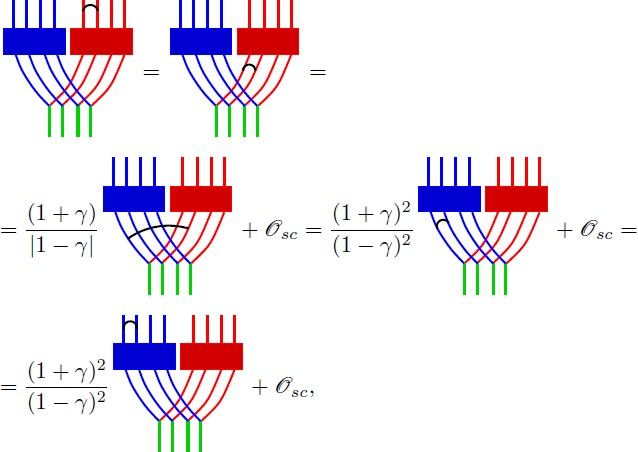(162)

where in the first line we have used the fact that the double grasping can be shifted through the group integration (due to gauge invariance (84)), and in the first and second terms on the second line we have used Eq. (142) to move the graspings on self-dual wires to the corresponding anti-self-dual wires. Eq. (161) follows immediately from the previous one; the argument works in the same way for any other pair of faces. Notice that the first equality in Eq. (162) implies that we can view the Plebanski constraint as applied in the frame of the tetrahedron as well as in a Lorentz invariant framework (the double grasping defines an intertwiner operator commuting with the projection $$P_{{\rm{inv}}}^e$$ represented by the box). An analogous statement also holds for the triangle constraints (14.1).

##### The 4-simplex constraints

Now we show the validity of the four simplex constraints in their form (158). As we show below, this last set of constraints follow from the Spin(4) gauge invariance of the EPRL node (i.e., the validity of the Gauss law) plus the validity of the tetrahedra constraints (156). Gauge invariance of the node takes the following form in graphical notation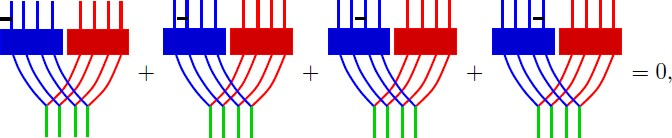(163)

where the above equation represents the gauge invariance under infinitesimal left SU(2) rotations. An analogous equation with insertions on the right is also valid. The validity of the previous equation can again be related to the invariance of the Haar measure used in the integration on the gauge group that defines the boxes (84).

Now we choose an arbitrary pair f and $$\bar f$$ (where, recall, $$\bar f$$ is one of the three possible faces whose dual triangle only shares a point with the one corresponding to f) and will show how the four volume e v defined by it equals the one defined by any other admissible pair. The first step is to show that we get the same result using the pair $$f - \bar f$$ and $$f - \overline{\overline f}$$, where $$\overline{\overline f}$$ is another of the three admissible faces opposite to f. The full result follows from applying the same procedure iteratively to reach any admissible pair. It will be obvious from the treatment given below that this is possible. Thus, for a given pair of admissible faces we have(164)

where going from the first line to the second and third lines we have simply used (163) on the bottom graspings on the right and left wires. The last line results from the validity of (156): notice that the second terms in the second and third lines add up to $${{\mathscr{O}}_{sc}}$$ as well as the third terms in the second and third line. There is an overall minus sign, which amounts to an orientation factor. It should be clear that we can apply the same procedure to arrive at any admissible pair.

### Modifications of the EPRL model

#### PEPRL is not a projector

Let us study in a bit more detail the object $$P_{{\rm{EPRL}}}^e({j_1}, \cdots, {j_4})$$. We see that it is made of two ingredients. The first is the projection to the maximum weight subspace ℋ j for γ > 1 in the decomposition of $${{\mathscr{H}}_{{j^ +},{j^ -}}}$$ for j±= (1 ± γ)j/2 (j± = (γ ± 1)j/2 for γ > 1) in terms of irreducible representations of an arbitrarily chosen SU(2) subgroup of Spin(4). The second ingredient is to eliminate the dependence on the choice of subgroup by group averaging with respect to the full gauge group Spin(4). This is diagrammatically represented in (147). However, $$P_{{\rm{EPRL}}}^e({j_1}, \cdots, {j_4})$$ is not a projector, namely

$$P_{{\rm{EPRL}}}^e{({j_1}, \cdots, {j_4})^2} \neq P_{{\rm{EPRL}}}^e({j_1}, \cdots, {j_4}){.}$$
(165)

Technically, this follows from (146) and the fact that

$$[P_{{\rm{inv}}}^e({\rho _1} \cdots {\rho _4}),({{\mathscr Y}_{{j_1}}} \otimes \cdots \otimes {{\mathscr Y}_{{j_4}}})] \neq 0,$$
(166)

i.e., the projection imposing the linear constraints (defined on the frame of a tetrahedron or edge) and the Spin(4) (or Lorentz) group averaging — rendering the result gauge invariant — do not commute. The fact the $$P_{{\rm{EPRL}}}^e({j_1}, \cdots, {j_4})$$ is not a projection operator has important consequences in the mathematical structure of the model:

1. 1.

From (148) one can immediately obtain the following expression for the EPRL amplitude

$${Z_{{\rm{EPRL}}}}(\Delta) = \sum\limits_{{\rho _f} \in {\mathscr K}} {\prod\limits_{f \in \Delta {\ast}} {{{\rm{d}}_{{\vert}1 - \gamma {\vert}{j \over 2}}}{{\rm{d}}_{(1 + \gamma){j \over 2}}}}} \prod\limits_e {P_{{\rm{EPRL}}}^e} ({j_1}, \cdots, {j_4}){.}$$
(167)

This expression has the formal structure of expression (88) for BF theory. However, the formal similarity is broken by the fact that $$P_{{\rm{EPRL}}}^e({j_1}, \cdots, {j_4})$$ is not a projection operator. From the formal perspective, the possibility that the amplitudes be defined in terms of a network of projectors (as in BF theory) might provide an interesting structure that might be of relevance in the definition of a discretization-independent model (see discussion in Part V). On the contrary, the failure of $$P_{{\rm{EPRL}}}^e({j_1}, \cdots, {j_4})$$ is not a projector may lead, in my opinion, to difficulties in the limit, where the complex Δ is refined: the increasing of the number of edges might produce either trivial or divergent amplitudes.Footnote 25

2. 2.

Another difficulty associated with $$P_{{\rm{EPRL}}}^e{({j_1}, \cdots, {j_4})^2} \neq P_{{\rm{EPRL}}}^e({j_1}, \cdots, {j_4})$$ is the failure of the amplitudes of the EPRL model, as defined here, to be consistent with the abstract notion of spin foams as defined in . This is a point of crucial importance under current discussion in the community. The point is that the cellular decomposition Δ has no physical meaning and is to be interpreted as a subsidiary regulating structure to be removed when computing physical quantities. Spin foams (as defined in Section 5) can fit in different ways on a given Δ, yet any of these different embeddings represent the same physical process (like the same gravitational field in different coordinates). Consistency requires the spin-foam amplitudes to be independent of the embedding, i.e., well defined on the equivalence classes of spin foams as defined by Baez in  (the importance of these consistency requirements was emphasized in ). The amplitude (167) fails this requirement due to $$P_{{\rm{EPRL}}}^e{({j_1}, \cdots, {j_4})^2} \neq P_{{\rm{EPRL}}}^e({j_1}, \cdots, {j_4})$$.

#### The Warsaw proposal

The above difficulties have a simple solution in the Riemannian case. As proposed in [49, 235] one can obtain a consistent modification of the EPRL model by replacing $$P_{{\rm{EPRL}}}^e$$ (167) by a genuine projector $$P_w^e$$, graphically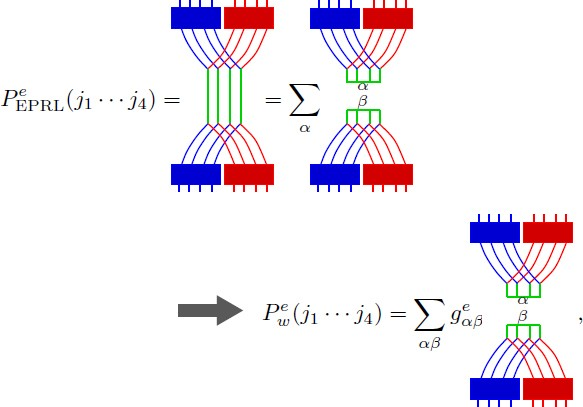(168)

where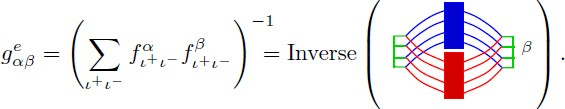It is easy to check that, by construction,

$${(P_w^e({j_1} \cdots {j_4}))^2} = P_w^e({j_1} \cdots {j_4}){.}$$
(169)

The variant of the EPRL model proposed in [49, 235] then takes the form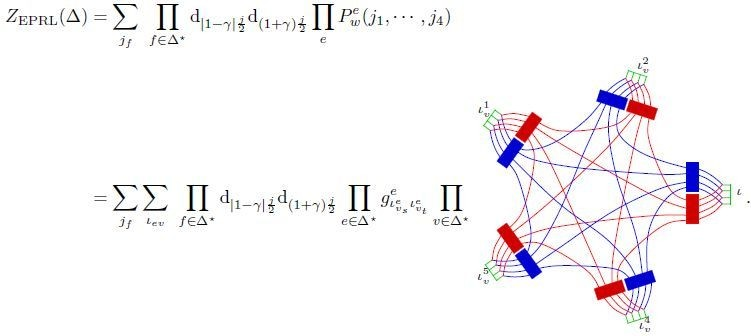(170)

Thus, in the modified EPRL model, edges e ∈ Δ* are assigned pairs of intertwiner quantum numbers $$\iota _{{\upsilon _s}}^e$$ and $$\iota _{{\upsilon _t}}^e$$ and an edge amplitude given by the matrix elements $$g_{\iota _{{\upsilon _s}}^e,\iota _{{\upsilon _t}}^e}^e$$ (where v s and v t stand for the source and target vertices of the given oriented edge). The fact that edges are not assigned a single quantum number is not really significant; one could go to a basis of normalized eigenstates of $$P_w^e$$ and rewrite the modified model above as a spin-foam model, where edges are assigned a single (basis element) quantum number. As the nature of such a basis and the quantum geometric interpretation of its elements is not clear at this stage, it seems simpler to represent the amplitudes of the modified model in the above form.

The advantages of the modified model are important, but a generalization of the above modification of the EPRL model in the Lorentzian case is still lacking. Notice that this modification does not interfere with the results of the semiclassical limit (to leading order) as reviewed in Section 13. The reason is that the matrix elements $$g_{\alpha \beta}^e \rightarrow {\delta _{\alpha \beta}}$$ in that limit .

### The coherent states representation

We have written the amplitude defining the EPRL model by constraining the state sum of BF theory. For semiclassical studies that we will review in Section 13, it is convenient to express the EPRL amplitude in terms of the coherent states basis. The importance of coherent states in spin-foam models was put forward in  and explicitly used to re-derive the EPRL model in . The coherent state technology was used by Freidel and Krasnov in  to introduce a new kind of spin-foam model for gravity: the FK models. In some cases, the FK model is equivalent to the EPRL model; we will review this in detail in Section 9.

The coherent state representation of the EPRL model is obtained by replacing (113) in each of the intermediate SU(2) (green) wires in the expression (148) of the EPRL amplitudes, namely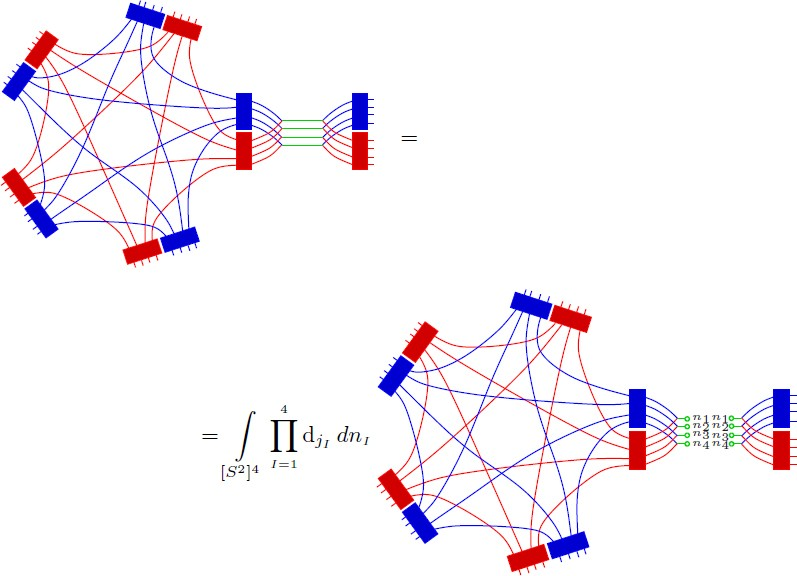(171)

#### The case of γ < 1

In this case, the coherent state property (114) implies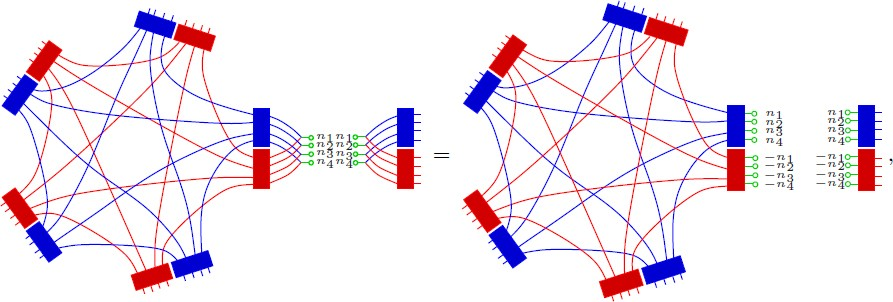(172)

where we used in the last line the fact that for γ < 1 the representations j of the subgroup SU(2) ∈ Spin(4) are of maximum weight, i.e., j = j+ + j. Doing this at each edge we get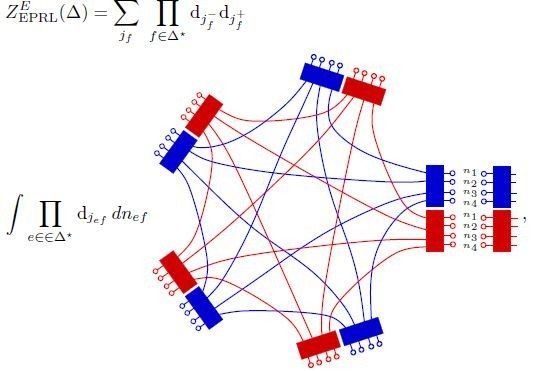(173)

where we have explicitly written the nS2 integration variables on a single cable. The expression above is very similar to the coherent states representation of Spin(4) BF theory given in Eq. (115). In fact, one would get the above expression if one would start from the expression (115) and would set $$n_{ef}^ + = n_{ef}^ - = {n_{ef}}$$, while dropping, for example, all the sphere integrations corresponding to the $$n_{ef}^ +$$, (or equivalently $$n_{ef}^ -$$). Moreover, by construction the coherent states participating in the previous amplitude satisfy the linear constraints (127) in expectation values, namely

$$\langle j,{n_{ef}}{\vert}{D^i}_f{\vert}j,{n_{ef}}\rangle = \langle j,{n_{ef}}{\vert}(1 - \gamma)J_f^{+ i} + (1 + \gamma)J_f^{- i}{\vert}j,{n_{ef}}\rangle = 0{.}$$

Thus the coherent states participating in the above representation of the EPRL amplitudes solve the linear simplicity constraints in the usual semiclassical sense. The same manipulations leading to (175) in Section 6 lead to a discrete effective action for the EPRL model, namely

$$Z_{{\rm{EPRL}}}^{\gamma < 1} = \sum\limits_{{j_f}} {\prod\limits_{f \in \Delta{\ast}} {{{\rm{d}}_{(1 - \gamma){{{j_f}} \over 2}}}} {{\rm{d}}_{(1 + \gamma){{{j_f}} \over 2}}}} \int {\prod\limits_{e \in \Delta {\ast}} {{{\rm{d}}_{{j_{ef}}}}} d{n_{ef}}} dg_{ev}^ - dg_{ev}^ + \exp \,(S_{{j^ \pm},{\bf{n}}}^{\gamma < 1}[{g^ \pm}]),$$
(174)

where the discrete action

$$S_{{j^ \pm},{\bf{n}}}^{\gamma < 1}[{g^ \pm}] = \sum\limits_{v \in \Delta {\ast}} {(S_{(1 - \gamma){{{j_f}} \over 2},{\bf{n}}}^v[{g^ -}] + S_{(1 + \gamma){{{j_f}} \over 2},{\bf{n}}}^v[{g^ +}])}$$
(175)

with

$$S_{j,{\bf{n}}}^v[g] = \sum\limits_{a < b = 1}^5 {2{j_{ab}}} \ln \langle {n_{ab}}{\vert}g_a^{- 1}{g_b}{\vert}{n_{ba}}\rangle,$$
(176)

and the indices a, b label the five edges of a given vertex. The previous expression is exactly equal to the form (86) of the BF amplitude. In the case of the gravity models presented here, the coherent state path integral representation (analogous to (117)) will be the basic tool for the study of the semiclassical limit of the models and the relationship with Regge discrete formulation of general relativity.

#### The case of γ > 1

The case of γ > 1 is more complicated . The reason is that step (172) directly leading to the discrete action in the previous case is no longer valid, as the representations of the subgroup SU(2) ∈ Spin(4) are now minimum instead of maximum weight. However, the representations j+ = j + j are of maximum weight. Therefore, we can insert a coherent states resolution of the identity on the right representations and get: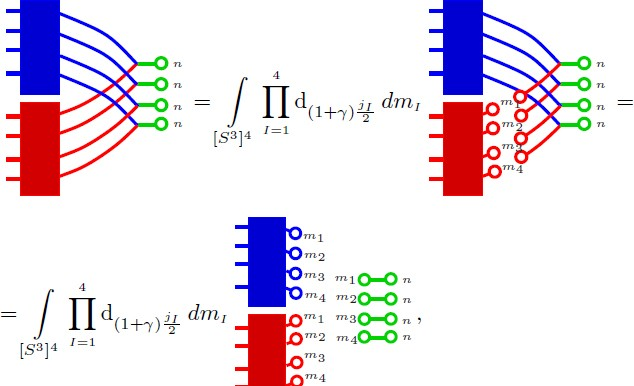(177)

where we are representing the relevant part of the diagram appearing in Eq. (171). In the last line we have used that j+ = j+j (i.e., maximum weight), and the graphical notation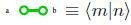, as follows from our previous conventions. With all this, one gets

$$\begin{array}{*{20}c} {Z_{{\rm{EPRL}}}^{\gamma > 1} = \quad \quad \quad \quad \quad \quad \quad \quad \quad \quad \quad \quad \quad \quad \quad \quad \quad \quad \quad \quad \quad \quad \quad \quad \quad} \\ {\sum\limits_{{j_f}} {\prod\limits_{f \in \Delta {\ast}} {{{\rm{d}}_{(1 - \gamma){{{j_f}} \over 2}}}} {{\rm{d}}_{(1 + \gamma){{{j_f}} \over 2}}}} \int {\prod\limits_{e \in \Delta {\ast}} {{{\rm{d}}_{{j_{ef}}}}}} {{\rm{d}}_{(1 + \gamma){{{j_{ef}}} \over 2}}}d{n_{ef}}\,d{m_{ef}}\,dg_{ev}^ - \,dg_{ev}^ + \,\,\exp \,(S_{{j^ \pm},{\bf{n}},{\bf{m}}}^{\gamma > 1}[{g^ \pm}]),} \\ \end{array}$$
(178)

where the discrete action

$$S_{{j^ \pm},{\bf{n}},{\bf{m}}}^{\gamma > 1}[{g^ \pm}] = \sum\limits_{v \in \Delta {\ast}} {S_{{j^ \pm},{\bf{n}},{\bf{m}}}^v[{g^ \pm}]}$$
(179)

with

$$\begin{array}{*{20}c} {S_{{j^ \pm},{\bf{n}},{\bf{m}}}^v[{g^ \pm}] = \sum\limits_{1 \leq a < b \leq 5} {{j_{ab}}(1 + \gamma)\log (\langle {m_{ab}}{\vert}g_{ab}^ + {\vert}{m_{ba}}\rangle) + {j_{ab}}(\gamma - 1)\log (\langle {m_{ab}}{\vert}g_{ab}^ - {\vert}{m_{ba}}\rangle) +}} \\ {\quad \quad \quad \quad \quad \quad \quad \quad \quad \quad \quad \quad \quad \quad \quad \quad + 2{j_{ab}}(\log (\langle {n_{ab}}{\vert}{m_{ab}}\rangle) + \log (\langle {m_{ba}}{\vert}{n_{ba}}\rangle)){.}} \\ \end{array}$$
(180)

It is important to point out that the commutation relations of basic fields — reflecting the simple algebraic structure of spin(4) — used here are those induced by the canonical analysis of BF theory presented previously. The presence of constraints generally modifies canonical commutation relations in particular in the presence of second-class constraints. For some investigation of the issue in the context of the EPRL and FK models, see . In  it is pointed out that the presence of secondary constraints in the canonical analysis of the Plebanski action should translate into additional constraints in the holonomies of the spin-foam models here considered (see also ). From the viewpoint of the GFT formulation, and using the tool of the non-commutative Fourier transform , this question has been explored in [54, 55] A possible view is that the simplicity constraints are here imposed for all times and thus secondary constraints should be imposed automatically.

Recently, a nice example has been worked out in three dimensions . In this example, one defines a Plebanski-like field theory consisting of SO(4) BF theory on a three-dimensional manifold plus simplicity constraints. The continuum theory is shown, via the canonical analysis, to be equivalent to three dimensional gravity: the analysis is non-trivial — there are first-class constraints, as well as second-class constraints, to be taken into account, in analogy with the case of four-dimensional Plebanski action. The quantum simplicity constraints are the analog of the ones in four dimensional models. The difference is that the second-class constraints required by the condition that simplicity constraints are preserved in time are now simple to impose at the quantum level. The authors show that unless one imposes the second-class constraints, one does not obtain the state sum model of three-dimensional BF theory, i.e., three-dimensional spin-foam gravity. A similar example in four dimensions has been analyzed in .

There are alternative derivations of the models presented in the previous sections. In particular one can derive them from a strict Lagrangian approach of Plebanski’s action. Such a viewpoint is taken in [92, 91, 97]. The path integral formulation of Plebanski theory using commuting B-fields was studied in , where it is shown that, only in the appropriate semiclassical limit the amplitudes coincide with the ones presented in the previous sections (this is just another indication that the construction of the models have a certain semiclassical input; see below). The spin-foam quantization of the Holst formulation of gravity via cubulations was investigated in . The simplicity constraints can also be studied from the perspective of the U(N) formulation of quantum geometry [160, 159, 161], which also has been explored in the Lorentzian sector . Such U(N) treatment is related to previous work, which has been extended to a completely new perspective on quantum geometry with possible advantageous features [104, 257]. For additional discussion on the simplicity constraints, see .

## The Lorentzian EPRL Model: Representation Theory and Simplicity Constraints

In this section we introduce some elements of SL(2, ℂ) representation theory and show how the linear-simplicity constraints are solved in the Lorentzian EPRL model [300, 167, 164].

### Representation theory of SL(2, ℂ) and the canonical basis

Unitary irreducible representations $${{\mathscr{H}}_{\rho, k}}$$ of SL(2, ℂ) are infinite dimensional and are labelled by a positive real number p ∈ ℝ+ and a half-integer k ∈ ℕ/2. The two Casimirs are $${C_1} = {1 \over 2}{J_{I\,J}}{J^{I\,J}} = {L^2} - {K^2}$$ and $${C_2} = {1 \over 2}{\ast}{J_{I\,J}}{J^{I\,J}} = K \cdot L$$, where Li are the generators of an arbitrary rotation subgroup and Ki are the generators of the corresponding boosts. The Casimirs act on $$\vert p,k\rangle \in {{\mathscr{H}}_{p,k}}$$ as follows

$$\begin{array}{*{20}c} {{C_1}{\vert}p,k\rangle = ({k^2} - {p^2} - 1){\vert}p,k\rangle,} \\ {{C_2}{\vert}p,k\rangle = 2pk{\vert}p,k\rangle {.}\quad \quad \quad \,\,} \\ \end{array}$$
(181)

For details on the representation theory of SL(2, ℂ), see [338, 203, 204]. As in the Riemannian case, the definition of the EPRL model requires the introduction of an (arbitrary) subgroup SU(2) ⊂ SL(2, ℂ). This subgroup corresponds to the internal gauge group of the gravitational phase space in connection variables in the time gauge. Therefore, in the quantum theory, the representation theory of this SU(2) subgroup will be important. This importance will soon become apparent from the imposition of the constraints that dehne the EPRL. The link between the unitary representations of SL(2, ℂ) and those of SU(2) is given by the decomposition

$${\mathscr H}_{p,k}=\overset{\infty}{{\underset{j=k}{\bigoplus}}}{\mathscr H}_j{.}$$
(182)

As the unitary irreducible representations of the subgroup SU(2) ∈ SL(2, ℂ) are essential in understanding the link of the EPRL model and the operator canonical formulation of LQG, it will be convenient to express the action of the generators of the Lie algebra sl(2, ℂ) in a basis adapted to the above equation. In order to do this, we first notice that the Lie algebra (120) can be equivalently characterized in terms of the generators of the rotation subgroup Li and the remaining boost generators Ki as follows

$$\begin{array}{*{20}c} {[{L_3},{L_ \pm}] = \pm {L_ \pm},\quad [{L_ +},{L_ -}] = 2\,{L_3},\quad \,\,\,\,\,\,\,\quad \quad \quad \quad \quad \quad \quad \,\,} \\ {[{L_ +},{K_ +}] = [{L_ -},{K_ -}] = [{L_3},{K_3}] = 0,\,\quad \quad \quad \quad \quad \quad \quad \quad \quad} \\ {[{K_3},{L_ \pm}] = \pm \,\,{K_ \pm},\quad [{L_ \pm},{K_ \mp}] = \pm 2\,\,{K_3},\quad \quad [{L_3},{K_ \pm}] = \pm \,{K_ \pm},} \\ {[{K_3},{K_ \pm}] = \mp \,{L_ \pm},\quad [{K_ +},{K_ -}] = 2\,\,{L_3},\quad \quad \,\,\quad \quad \quad \quad \quad \quad \,\,} \\ \end{array}$$
(183)

where K± = K1 ± iK2 and L± = L1±iL2. The action of the previous generators in the basis |p, k, j, m) can be shown to be 

$$\begin{array}{*{20}c} {{L^3}|p,\,k,\,j,\,m\rangle = m|p,\,k,\,j,\,m\rangle ,\,\quad \quad \quad \quad \quad \quad \quad \quad \quad \quad \quad \quad \quad \quad \quad \quad \quad \quad \quad \quad \quad \quad \quad \quad \quad \quad} \\ {{L^ +}|p,\,k,\,j,\,m\rangle = \sqrt {(j + m + 1)(j - m)} |p,\,k,\,j,\,m + 1\rangle ,\,\quad \quad \quad \quad \quad \quad \quad \quad \quad \quad \quad \quad \quad \quad \quad \quad \quad \quad} \\ {{L^ -}|p,\,k,\,j,\,m\rangle = \sqrt {(j + m)(j - m + 1)} |p,\,k,\,j,\,m - 1\rangle ,\,\quad \quad \quad \quad \quad \quad \quad \quad \quad \quad \quad \quad \quad \quad \quad \quad \quad \quad} \\ {{K^3}|p,\,k,\,j,\,m\rangle = {\alpha _j}\sqrt {{j^2} - {m^2}} |p,\,k,\,j - 1,\,m\rangle + {\gamma _j}m|p,\,k,\,j,\,m\rangle - {\alpha _{j + 1}}\sqrt {{{(j + 1)}^2} - {m^2}} |p,\,k,\,j + 1,\,m\rangle ,\,} \\ {{K^ +}|p,\,k,\,j,\,m\rangle = {\alpha _j}\sqrt {(j - m)(j - m - 1)} |p,\,k,\,j - 1,\,m + 1\rangle \quad \quad \quad \quad \quad \quad \quad \quad \quad \quad \quad \quad \quad \quad \quad \,\,\,\,\,\,} \\ {+ {\gamma _j}\sqrt {(j - m)(j + m + 1)} |p,\,k,\,j,\,m + 1\rangle \quad \quad \quad \quad \quad \quad \quad \quad \quad \quad \quad \quad \quad \quad \quad \quad \quad \quad \quad \quad \quad \quad \quad} \\ {+ {\alpha _{j + 1}}\sqrt {(j + m + 1)(j + m + 2)} |p,\,k,\,j + 1,\,m + 1\rangle ,\,\quad \quad \quad \quad \quad \quad \quad \quad \quad \quad \quad \quad \quad \quad \quad \quad \quad \quad \quad \,\,\,\,} \\ {{K^ -}|p,\,k,\,j,\,m\rangle = - {\alpha _j}\sqrt {(j + m)(j + m - 1)} |p,\,k,\,j - 1,\,m - 1\rangle \quad \quad \quad \quad \quad \quad \quad \quad \quad \quad \quad \quad \quad \quad \quad} \\ {+ {\gamma _j}\sqrt {(j + m)(j - m + 1)} |p,\,k,\,j,\,m - 1\rangle \quad \quad \quad \quad \quad \quad \quad \quad \quad \quad \quad \quad \quad \quad \quad \quad \quad \quad \quad \quad \quad \quad \quad} \\ {- {\alpha _{j + 1}}\sqrt {(j - m + 1)(j - m + 2)} |p,\,k,\,j + 1,\,m - 1\rangle ,\,\quad \quad \quad \quad \quad \quad \quad \quad \quad \quad \quad \quad \quad \quad \quad \quad \quad \quad \quad \,\,\,\,} \\ \end{array}$$
(184)

where

$${\gamma _j} = {{kp} \over {j(j + 1)}}\quad {\rm{and}}\quad {\alpha _j} = i\sqrt {{{({j^2} - {k^2})({j^2} + {p^2})} \over {{j^2}(4{j^2} - 1)}}} {.}$$
(185)

This concludes the review of the representation theory that is necessary for the definition of the EPRL model.

### Lorentzian model: the linear simplicity constraints

As in the Riemannian case, it can be shown as in Section (7.4) that the quadratic simplicity constraints (154) are implied, in the discrete setting, by the linear constraint on each face

$$D_f^i = L_f^i - {1 \over \gamma}K_f^i \approx 0,$$
(186)

where the label f makes reference to a face f ∈ Δ*, and where (very importantly) the subgroup SU(2) ⊂ SL(2, ℂ) that is necessary for the definition of the above constraints is chosen arbitrarily at each tetrahedron, equivalent on each edge e ∈ Δ*. The commutation relations of the previous tetrahedron constraint are

$$\begin{array}{*{20}c} {[D_f^i,D_{f{\prime}}^j] = {\delta _{ff{\prime}}}{\epsilon ^{ij}}_k\left[ {(1 - {1 \over {{\gamma ^2}}})L_f^k - {2 \over \gamma}K_e^k} \right]\,\,\,\,\,\,\,\,\,} \\ {\quad \quad \quad = 2{\delta _{ee{\prime}}}{\epsilon ^{ij}}_k{D^k} - {\delta _{ee{\prime}}}{{{\gamma ^2} + 1} \over {{\gamma ^2}}}{\epsilon ^{ij}}_kL_f^k.} \\ \end{array}$$
(187)

The previous commutation relation implies that the constraint algebra is not closed and therefore cannot be imposed as operator equations of the states summed over in the BF partition function in general. There are two interesting exceptions:

1. 1.

The first one is to take γ = ±i, in which case the constraint (186) could, in principle, be imposed strongly. However, the self-dual sector is not present in $${{\mathscr{H}}_{p,k}}$$.

2. 2.

The second possibility is to work in the sector where $$L_e^i = 0$$. Where, as in the Riemannian case, it corresponds to the Barret-Crane model  with the various limitations discussed in Section 10.

The EPRL model is obtained by restricting the representations appearing in the expression of the BF partition function, so that at each tetrahedron the linear constraints (186) are satisfied the strongest possible way that is compatible with the uncertainties relations stemming from (187). In addition, one would add the requirement that the state-space of tetrahedra is compatible with the state-space of the analogous excitation in the canonical context of LQG so that arbitrary states in the kinematical state of LQG have non-trivial amplitudes in the model.

### On the weak imposition of the linear simplicity constraints

We now discuss the weak imposition of the linear simplicity constraints in the quantum framework. There are essentially three views in the literature: two of them, discussed below, directly concerns the way the EPRL model has usually been introduced. The third possibility is the semiclassical view based on the coherent state representation leading to the FK model (see Section 9).

#### Lorentzian model: The Gupta-Bleuler criterion

As in the Riemannian case, the fact that the constraints do not form a closed (first class) algebra in the generic case requires a weaker sense in which the constraints are imposed. One possibility is to consider the Gupta-Bleuler criterion consisting in selecting a suitable class of states for which the matrix elements on vanish. In this respect, we notice that if we choose the subspace $${{\mathscr{H}}_{j}}\subset{{\mathscr{H}}_{p,k}}$$ we have

$$\begin{array}{*{20}c} {\langle p,k,j,q{\vert}D_f^3{\vert}p,k,j,m\rangle = {\delta _{q,m}}m(1 - {{{\gamma _j}} \over \gamma}),\quad \quad \quad \quad \quad \quad \quad \quad} \\ {\langle p,k,j,q{\vert}D_f^ \pm {\vert}p,k,j,m\rangle = {\delta _{q \pm 1,m}}\sqrt {(j \pm m + 1)(j \mp m)} \left({1 - {{{\gamma _j}} \over \gamma}} \right){.}} \\ \end{array}$$

One immediately observes that matrix elements of the linear constraints vanish in this subclass if one can choose

$${\gamma _j} = {{kp} \over {j(j + 1)}} = \gamma {.}$$
(188)

Now the situation simplifies with respect to the Riemannian case, as there is only one single case. The linear simplicity constraint is satisfied as long as p = γj(j+1)/k. Therefore, one has an integer worth of possibilities. In particular, given j, one has k = jr for all possible half-integers such that k > 0 . As we will see in what follows the best choice from the point of view of the Master-constraint criterion is k = j, from which it follows that

$$p = \gamma (j + 1){.}$$
(189)

#### Lorentzian model: The Master-constraint criterion

Another criterion for weak imposition can be developed by studying the spectrum of the Master constraint

$$\begin{array}{*{20}c} {{M_f} = {D_f}\cdot{D_f} = {L^2} - {1 \over \gamma}2L\cdot K + {1 \over {{\gamma ^2}}}{K^2}\quad \quad \quad \quad \quad \quad \,\,} \\ {\quad \quad = {1 \over {{\gamma ^2}}}\left[ {(1 + {\gamma ^2}){L^2} - 2\gamma {C_2} - {C_1}} \right].} \\ \end{array}$$
(190)

Strong imposition of the constraints $$D_f^i$$ would amount to looking for the kernel of the Master constraint M f . However, generically the positive operator associated with the Master constraint does not have the zero eigenvalue in the spectrum due to the open nature of the constraint algebra (187). The proposal of  is to look for the minimum eigenvalue among spaces Explicitly

$${M_f}{\vert}\psi \rangle = {m_{p,k,j}}{\vert}\psi \rangle,$$
(191)

where

$${m_{p,k,j}} = {1 \over {{\gamma ^2}}}\left[ {{p^2} + 1 - {k^2} + (1 + {\gamma ^2})j(j + 1) - 2\gamma kp} \right]{.}$$

For given j the minimum eigenvalue is obtained when k = j and p = γj in which case one gets m p,k,j m j for m j = ℏ2((1 + γ2)j + 1). Again we see that the important condition

$${M_f}{\vert}\gamma j,j,j,m\rangle = {\mathscr O}_{sc}$$
(192)

holds (recall the notion $${{\mathscr{O}}_{sc}}$$ from Section 7.2.4). Interestingly, the states that satisfy the previous semiclassical criterion satisfy the Gupta-Bleuler criterion not exactly and only in the large spin sense. However, the advantage of the Master-constraint criterion is clear from the point of view of cylindrical consistency as the choice p = γ(j + 1) obtained in the previous subsection would imply that j = 0 spin-network links would be have a non-trivial amplitude in the path integral defined in Section 7.3. Such thing would be in sharp conflict with diffeomorphism invariance in the model.

Therefore, as in the Riemannian case, we will use the Master-constraint criterion as the basic ingredient in the definition of the EPRL model. One can write a Lorentz invariant constraint  from the Master constraint using the $$D_f^i = 0$$ classically. This leads to the following Lorentz invariant condition in terms of the SL(2, ℂ) Casimirs

$$M_f^{L\,I} = (1 - {\gamma ^2}){C_2} - 2{C_1}\gamma ,$$
(193)

where C1 and C2 are the two sl(2, ℂ) Casimirs given in Eq. (181).Footnote 26 Surprisingly, the conditions p = γj and k = j minimizing the Master constraint, solve its Lorentz invariant version strongly! This resolves the cylindrical consistency issue pointed out above. More recently, it has been shown that the constraint solution p = γj and k = j also follows naturally from a spinor formulation of the simplicity constraints [369, 156, 256].

#### Lorentzian model: Restrictions on the Immirzi parameter

Unlike the Riemannian model, all values of spin j are allowed, and no restrictions on the Immirzi parameter arise.

#### Lorentzian model: Overview of the solutions of the linear simplicity constraints

In the Lorentzian models one restricts the SL(2, ℂ) representations of BF theory to those satisfying

$$p = \gamma j\quad {\rm{and}}\quad k = j$$
(194)

for j ∈ ℕ/2. From now on we denote the subset of admissible representation of SL(2, ℂ) as

$${{\mathscr K}_\gamma} \subset {\rm{Irrep}}(SL(2,C))$$
(195)

The admissible quantum states Ψ are elements of the subspace $${{\mathscr{H}}_j} \subset {{\mathscr{H}}_{\gamma j,j}}$$ (i.e., minimum weight states) and satisfy the constraints (186) in the following semiclassical sense:

$$(K_f^i - \gamma L_f^i)\Psi = {{\mathscr O}_{sc}},$$
(196)

where, as in the Riemannian case, the symbol $${{\mathscr{O}}_{sc}}$$ (order semiclassical) denotes a quantity that vanishes in the limit ℏ → 0, j → ∞ with ℏj = constant.

### Presentation of the EPRL Lorentzian model

As briefly discussed in Section 8, unitary irreducible representations of SL(2, ℂ) are infinite dimensional and labeled by a positive real number p ∈ ℝ+ and a half-integer k ∈ ℕ/2. These representations are the ones that intervene in the harmonic analysis of square integrable functions of SL(2,ℂ) . Consequently, one has an explicit expression of the delta function distribution (defined on such a test function), namely

$$\delta (g) = \sum\limits_k {\int\nolimits_{{{\mathbb R} +}} \,dp({p^2} + {k^2})} \sum\limits_{j,m} {D_{jmjm}^{p,k}(g)},$$
(197)

where $$D_{jmj{\prime}m{\prime}}^{p,k}(g)$$ with jk and jm ≥ −j (similarly for the primed indices) are the matrix elements of the unitary representations pk in the canonical basis . One can use the previous expression for the Lorentzian version of Eq. (86) to introduce a formal definition of the BF amplitudes, which now would involve integration of the continuous labels p f in addition of sums over discrete quantum numbers such as k, j and m. The Lorentzian version of the EPRL model can be obtained from the imposition of the linear simplicity constraints to this formal expression, as discussed in Section 8.2. As the continuum labels p f are restricted to p f = γj f , the Lorentzian EPRL model becomes a state-sum model, as its Riemannian relative. Using the following graphical notation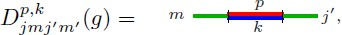(198)

the amplitude is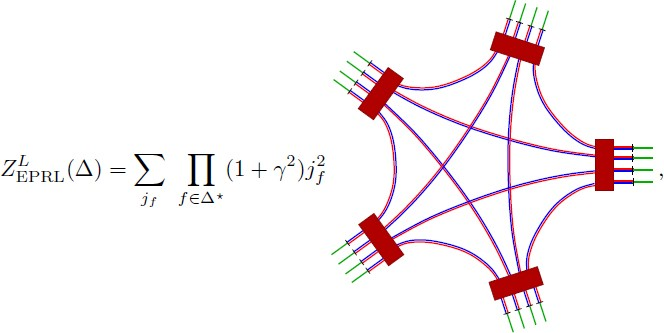where the boxes now represent SL(2, ℂ) integrations with the invariant measure. The previous amplitude is equivalent to its spin-foam representation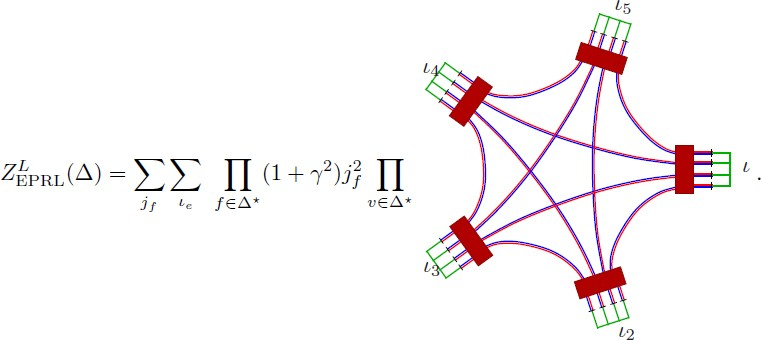The vertex amplitude is divergent due to the presence of a redundant integration over SL(2, ℂ), it becomes finite by dropping an arbitrary integration, i.e., removing any of the 5 boxes in the vertex expression .

### The coherent state representation

It is crucial to obtain the coherent-state representation of the Lorentzian models. As in the Riemannian case, one simply inserts a resolution of the identities (108) on the intermediate SU(2) (green) wires in (199) from which it results that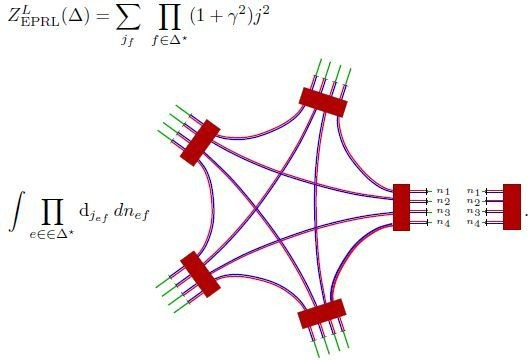(199)

## The Freidel-Krasnov Model

Shortly after the appearance of , Freidel and Krasnov  introduced a set of new spin-foam models for four-dimensional gravity using the coherent state basis of the quantum tetrahedron of Livine and Speziale . The idea is to impose the linearized simplicity constraints (126) directly as a semiclassical condition on the coherent state basis. As we have seen above, coherent states are quantum states of the right and left tetrahedra in BF theory, which have a clear-cut semiclassical interpretation through their property (112). We have also seen that the imposition of the linear constraints (126) à la EPRL is, in essence, semiclassical, as the constraints are strictly valid only in the large spin limit. In the FK approach, one simply accepts from the starting point that, due to their property of not defining the set that is closed under commutation relations, the Plebanski constraints are to be imposed semiclassically. One defines new models by restricting the set of coherent states entering into the coherent-state representation of Spin(4) BF theory (115) to those that satisfy condition (126) in expectation values. They also emphasize how the model  corresponds to the sector γ = ∞, which has been shown to be topological .

### The case of γ < 1

For γ < 1, the vertex amplitude is identical to the EPRL model. This is apparent in the coherent-state expression of the EPRL model (174). Thus, we have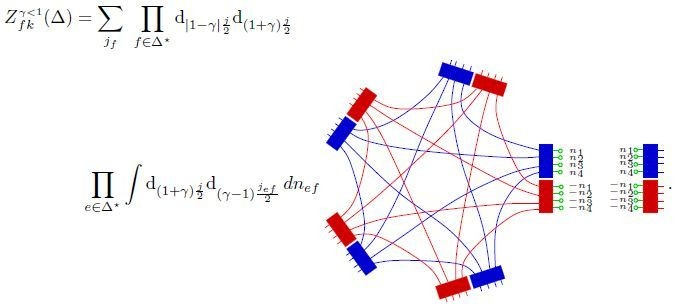(200)

From the previous expression we conclude that the vertex amplitudes of the FK and EPRL model coincide for γ < 1

$$A_{v\,\,\,\,fk}^{\gamma < 1} = A_{v\,\,{\rm{EPRL}}}^{\gamma < 1}{.}$$
(201)

However, notice that different weights are assigned to edges in the FK model. This is due to the fact that one is restricting the Spin(4) resolution of identity in the coherent basis in the previous expression, while in the EPRL model the coherent state resolution of the identity is used for SU(2) representations. This difference is important and has to do with the still-unsettled discussion concerning the measure in the path integral representation (see Section 12.1 and references therein).

### The case of γ > 1

For the case of γ > 1 the FK amplitude is given by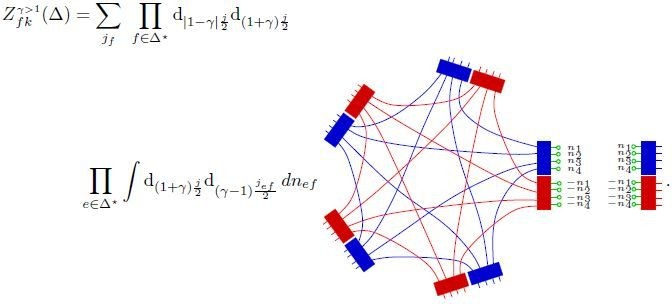(202)

The study of the coherent state representation of the FK model for γ > 1, and comparison with Eq. (177) for the EPRL model, clearly shows the difference between the two models in this regime.

$$Z_{fk}^\gamma = \sum\limits_{{j_f}} {\prod\limits_{f \in \Delta {\ast}} {{{\rm{d}}_{(1 - \gamma){{{j_f}} \over 2}}}} {{\rm{d}}_{(1 + \gamma){{{j_f}} \over 2}}}} \int {\prod\limits_{e \in \Delta {\ast}} {{{\rm{d}}_{{\vert}1 - \gamma {\vert}{{{j_{ef}}} \over 2}}}}} {{\rm{d}}_{(1 + \gamma){{{j_{ef}}} \over 2}}}d{n_{ef}}dg_{ev}^ - \,dg_{ev}^ + \exp \,(S_{{j^ \pm},{\bf{n}}}^{fk\,\,\gamma}[{g^ \pm}]),$$
(203)

where the discrete action

$$S_{{j^ \pm},{\bf{n}}}^{fk\,\,\gamma}[{g^ \pm}] = \sum\limits_{v \in \Delta {\ast}} {(S_{(1 - \gamma){{{j_f}} \over 2},{\bf{n}}}^v[{g^ -}] + S_{(1 + \gamma){{{j_f}} \over 2},s(\gamma){\bf{n}}}^v[{g^ +}]),}$$
(204)

where s(γ) = sign(l − γ) and

$$S_{j,{\bf{n}}}^v[g] = \sum\limits_{a < b = 1}^5 {2{j_{ab}}} \ln \langle {n_{ab}}\vert g_a^{- 1}{g_b}\vert {n_{ba}}\rangle,$$
(205)

with the indices a and b labeling the five edges of a given vertex.

## The Barrett-Crane Model

The Barrett-Crane models [60, 59] can easily be obtained in the present framework as a suitable limiting case γ → ∞ of the previous constructions. One way to get it is to take the limit γ → ∞ of the linear simplicity constraint (126) at the classical level. In this way the constraint becomes

$${D^i} = L_f^i \approx 0$$
(206)

with which the constraint algebra closes. One can impose the constraints strongly in this case. The solution is obtained by setting j f = 0 in all faces at the level of the amplitude (148). An immediate consequence of this is that $$j_f^ + = j_f^ -$$ and all tetrahedra are assigned a unique intertwiner: the Barrett-Crane intertwiner. Explicitly, setting j f = 0 in (148) we get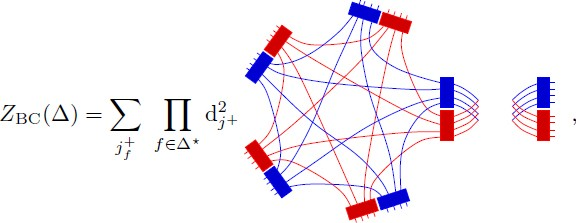(207)

where, since $$j_f^ + = j_f^ -$$, we are arbitrarily choosing $$j_f^ +$$ as the spins summed over in the state sum. Eliminating redundant group integrations we get the previous amplitude in the spin-foam representation, namely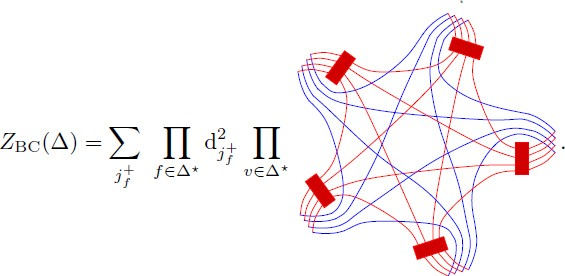(208)

Observe that the vertex amplitude depends only on the 10 corresponding $$j_f^ +$$, this is the Riemannian Barrett-Crane vertex. It is interesting to write the analog of Eq. (147). The Barrett-Crane model is obtained by the modification of the BF projectors in (88), so that

$${Z_{{\rm{BC}}}}(\Delta) = \sum\limits_{j_f^ +} {\prod\limits_{f \in \Delta {\ast}} {{\rm{d}}_{{j^ +}}^2} \prod\limits_e {P_{{\rm{BC}}}^e}} (j_1^ +, \cdots, j_4^ +),$$
(209)

with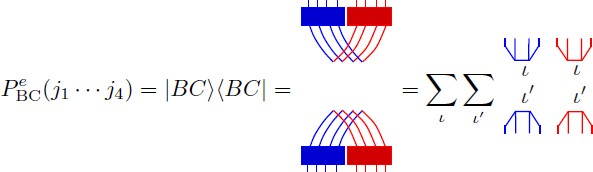(210)

, where |BC⟩ is the Barrett-Crane intertwiner. The tetrahedra state space is one-dimensional once the four quantum numbers $$j_f^ +$$ have been fixed. Indeed, it has been shown that the state space of strong solutions of the quadratic quantum Plebanski constraints is one dimensional and it is given by the BC intertwiner |BC⟩ .

### The coherent states representation of the BC amplitude

One can insert a coherent states resolution of the identity (111) in the 10 wires obtaining the following coherent state representation of the Barrett-Crane amplitude

$${Z_{{\rm{BC}}}} = \sum\limits_{j_f^ +} {\prod\limits_{f \in \Delta {\ast}} {{\rm{d}}_{j_f^ +}^2} \int {\prod\limits_{e \in \Delta {\ast}} {{d_{{j_{ef}}}}}}} d{n_{ef}}\,dg_{ev}^ + \exp \,(S_{{j^ +},{\bf{n}}}^{BC}[{g^ +}]),$$
(211)

where the discrete action

$$S_{{j^ +},{\bf{n}}}^{BC}[{g^ +}] = \sum\limits_{v \in \Delta {\ast}} {\sum\limits_{a < b = 1}^5 {2j_{ab}^ + \ln \langle {n_{ab}}{\vert}{{(g_a^ +)}^{- 1}}g_b^ + {\vert}{n_{ab}}\rangle}.}$$
(212)

Notice that there are only 10 normals n ab , as in this case n ab = n ba . The large spin asymptotics of the Barrett-Crane vertex has been investigated in .

## Boundary Data for the New Models and Relation with the Canonical Theory

So far we have considered cellular complexes with no boundary. Transition amplitudes are expected to be related to the definition of the physical scalar product, as discussed in Section 2.2. In order to dehne them, one needs to consider complexes with boundaries. Boundary states are defined on the boundary of the dual two-complex Δ* that we denote Δ*. The object Δ* is a one-complex (a graph). According to the construction of the model (Sections 7 and 8), boundary states are in one-to-one correspondence with SU(2) spin networks. This comes simply from the fact that links (one-cells) Δ* inherit the spin labels (unitary irreducible representations of the subgroup SU(2)) of the boundary faces, while nodes (zero-cells) nΔ* inherit the intertwiner levels of boundary edges.

At this stage one can associate the boundary data with elements of a Hilbert space. Being in one-to-one correspondence with SU(2) spin networks, a natural possibility is to associate to them an element of the kinematical Hilbert space of LQG. More precisely, with a given colored boundary graph γ with links labeled by spins j and nodes labeled by interwiners ι n , we associate a cylindrical function $${\Psi _{\gamma, \{{j_\ell}\}, \{{\iota _n}\}}} \in {{\mathscr{L}}^2}(SU{(2)^{{N_\ell}}})$$, where here N denotes the number of links in the graph γ (see Eq. 69). In this way, the boundary Hilbert space associated with Δ* is isomorphic (if one used the natural Ashtekar-Lewandowski measure) with the Hilbert space of LQG truncated to that fixed graph. Moreover, geometric operators such as volume and area defined in the covariant context are shown to coincide with the corresponding operators defined in the canonical formulation [141, 142]. Now, if cellular complexes are dual to triangulations, then the boundary spin networks can have, at most, four valent nodes. This limitation can be easily overcome: as in BF theory, the EPRL amplitudes can be generalized to arbitrary complexes with boundaries given by graphs with nodes of arbitrary valence. The extension of the model to arbitrary complexes was first studied in [237, 236] and has been revisited in .

Alternatively, one can associate the boundary states with elements of $${{\mathscr{L}}^2}(Spin{(4)^{{N_\ell}}})$$ (in the Riemannian models) — or carefully dehne the analog of spin-network states as distributions in the Lorentzian case.Footnote 27 In this case, one gets a special kind of spin-network states that are a subclass of the projected spin networks introduced in [19, 247] in order to dehne an heuristic quantization of the (non-commutative and very complicated) Dirac algebra of a Lorentz connection formulation of the phase space of gravity [15, 10, 19, 9, 8, 7, 17, 21]. The fact that these special subclasses of projected spin networks appear naturally as boundary states of the new spin foams is shown in .

Due to their similarity to γ < 1, the same relationship between boundary data and elements of the kinematical Hilbert space hold for the FK model. However, such a simple relationship does not hold for the model in the case of γ > 1.

The role of knotting in the Hilbert space of the EPRL model is studied in .

## Further Developments and Related Models

### The measure

The spin-foam amplitudes discussed in the previous sections have been introduced by constraining the BF histories through the simplicity constraints. However, in the path integral formulation, the presence of constraints has the additional effect of modifying the weights with which those histories are to be summed: second-class constraints modify the path-integral measure (in the spin-foam context this issue was raised in ). As pointed out before, this question has not been completely settled yet in the spin-foam community. The explicit modification of the formal measure in terms of continuous variables for the Plebanski action was presented in . A systematic investigation of the measure in the spin-foam context was attempted in  and . As pointed out in , there are restrictions in the manifold of possibilities coming from the requirement of background independence. The simple BF measure chosen in the presentation of the amplitudes in the previous sections satisfies these requirements. There are other consistent possibilities; see, for instance,  for a modified measure, which remains extremely simple and is suggested from the structure of LQG.

### Relation with the canonical formulation: the scalar constraint

An important question is the relationship between the spin-foam amplitudes and the canonical-operator formulation. The question of whether one can reconstruct the Hamiltonian constraints out of spin-foam amplitudes has been analyzed in detail in three dimensions. For the study of quantum three-dimensional gravity from the BF perspective, see ; we will in fact present this perspective in detail in the three-dimensional part of this article. For the relationship with the canonical theory using variables that are natural from the Regge gravity perspective, see [94, 93]. In four dimensions the question has been investigated in  in the context of the new spin foam models. In the context of group field theories this issue is explored in . Finally, spin foams can, in principle, be obtained directly from the implementation of the Dirac program using path-integral methods; this avenue has been explored in [219, 220], from which a discrete path-integral formulation followed . The question of the relationship between covariant and canonical formulations in the discrete setting has also been analyzed in .

### Spin-foam models with timelike faces

By construction, all tetrahedra in the FK and EPRL models are embedded in a spacelike hypersurface and, hence, have only spacelike triangles. It seem natural to ask the question of whether a more general construction allowing for timelike faces is possible. The models described in previous sections have been generalized in order to include timelike faces in the work of Conrady [125, 120, 124]. An earlier attempt to define such models in the context of the Barrett-Crane model can be found in .

### Coupling to matter

The issue of the coupling of the new spin-foam models to matter remains, to a large extent, unexplored territory. Nevertheless, some results can be found in the literature. The coupling of the Barrett-Crane model (the γ → ∞ limit of the EPRL model) to Yang-Mills fields was studied in . More recently, the coupling of the EPRL model to fermions has been investigated in [217, 73]. A novel possibility of unification of the gravitational and gauge fields was recently proposed in .

### Cosmological constant

The introduction of a cosmological constant in the construction of four-dimensional spin-foam models has a long history. Barrett and Crane introduced a vertex amplitude  in terms of the Crane and Yetter model  for BF theory with cosmological constant. The Lorentzian quantum-deformed version of the previous model was studied in . For the new models, the coupling with a cosmological constant is explored in terms of the quantum deformation of the internal gauge symmetry in [139, 215, 216] as well as (independently) in .

### Spin-foam cosmology

The spin-foam approach applied to quantum cosmology has been explored in [74, 366, 222, 79, 335, 334]. The spin-foam formulation can also be obtained from the canonical picture provided by loop quantum cosmology (see  and references therein). This has been explored systematically in [29, 28, 27, 108]. Very recent results are .

### Constraints are imposed semiclassically

As we have discussed in the introduction of the new models, the Heisenberg uncertainty principle precludes the strong imposition of the Plebanski constraints that reduce BF theory to general relativity. The results on the semiclassical limit of these models seem to indicate that metric gravity should be recovered in the low energy limit. However, its seems likely that the semiclassical limit could be related to certain modifications of Plebanski’s formulation of gravity [240, 241, 243, 242, 244]. A simple interpretation of the new models in the context of the bi-gravity paradigm proposed in  could be of interest.

### Group field theories associated to the new spin foams

As pointed out in , spin foams can be interpreted in close analogy to Feynman diagrams. Standard Feynman graphs are generalized to 2-complexes and the labeling of propagators by momenta to the assignment of spins to faces. Finally, momentum conservation at vertices in standard Feynmanology is now represented by spin-conservation at edges, ensured by the assignment of the corresponding intertwiners. In spin-foam models, the non-trivial content of amplitudes is contained in the vertex amplitude, which in the language of Feynman diagrams can be interpreted as an interaction. This analogy is indeed realized in the formulation of spin-foam models in terms of a group field theory (GFT) [315, 314].

The GFT formulation resolves by definition the two fundamental conceptual problems of the spin-foam approach: diffeomorphism gauge symmetry and discretization dependence. The difficulties are shifted to the question of the physical role of λ (a dimensionless coupling constant in the theory) and the convergence of the corresponding perturbative series.

This idea has been studied in more detail in three dimensions. In  scaling properties of the modification of the Boulatov group field theory introduced in  were studied in detail. In a further modification of the previous model (known as colored tensor models ) new techniques based on a suitable 1/N expansion imply that amplitudes are dominated by spherical topology ; moreover, it seems possible that the continuum limit might be critical, as in certain matrix models [209, 95, 210, 211, 339]. However, it is not yet clear if there is a sense in which these models correspond to a physical theory. The naive interpretation of the models is that they correspond to a formulation of 3D quantum gravity including a dynamical topology.

## The Semiclassical Limit

Having introduced the relevant spin-foam models in the previous sections, we now present the results on the large-spin asymptotics of the spin-foam amplitudes suggesting that, on a fixed discretization, the semiclassical limit of the EPRL-FK models is given by Regge’s discrete formulation of general relativity [61, 64].

The semiclassical limit of spin foams is based on the study of the the large-spin-limit asymptotic behavior of coherent-state spin-foam amplitudes. The notion of large spin can be defined by the rescaling of quantum numbers and Planck’s constant according to jλj and ℏ > ℏ/λ and taking λ >> 1. In this limit the quantum geometry approximates the classical one when tested with suitable states (e.g., coherent states). However, the geometry remains discrete during this limiting process, as the limit is taken on a fixed regulating cellular structure. That is why one usually makes a clear distinction between the semiclassical limit and the continuum limit. In the semiclassical analysis presented here, one can only hope to make contact with discrete formulations of classical gravity; hence, the importance of Regge calculus in the discussion of this section.

The key technical ingredient in this analysis is the representation of spin-foam amplitudes in terms of the coherent-state basis introduced in Section 6.2. Here we follow [61, 64, 62, 65, 63]. The idea of using coherent states and discrete effective actions for the study of the large-spin asymptotics of spin-foam amplitudes was put forward in [122, 121]. The study of large-spin asymptotics has a long tradition in the context of quantum gravity, dating back to the work of Ponzano-Regge . More directly related to our discussion here are the early works [68, 69]. The key idea is to use asymptotic stationary phase methods for the amplitudes, written in terms of the discrete actions presented in Sections 7, 8, and 9.

### Vertex amplitudes asymptotics

In this section we review the results of the analysis of the large-spin asymptotics of the EPRL vertex amplitude for both the Riemannian and Lorentztian models. We follow the notation and terminology of  and related papers.

#### SU(2) 15j-symbol asymptotics

As SU(2) BF theory is quite relevant for the construction of the EPRL-FK models, the study of the large-spin asymptotics of the SU(2) vertex amplitude is a key ingredient in the analysis of . The coherent state vertex amplitude is

$$15j(j,{\bf{n}}) = \int {\prod\limits_{a = 1}^5 {d{g_a}} \prod\limits_{1 \leq a \leq b \leq 5} {{{\langle {n_{ab}}{\vert}g_a^{- 1}{g_b}{\vert}{n_{ba}}\rangle}^{2{j_{ab}}}}}},$$
(213)

which depends on 10 spins j ab and 20 normals n ab n ba . The previous amplitude can be expressed as

$$15j(j,{\bf{n}}) = \int {\prod\limits_{a = 1}^5 {d{g_a}} \prod\limits_{1 \leq a \leq b \leq 5} {\exp \,{S_{j,{\bf{n}}}}[g]}},$$
(214)
$${S_{j,{\bf{n}}}}[g] = \sum\limits_{a < b = 1}^5 {2{j_{ab}}} \ln \langle {n_{ab}}{\vert}g_a^{- 1}{g_b}{\vert}{n_{ba}}\rangle,$$
(215)

and the indices a, b label the five edges of a given vertex. The previous expression is exactly equal to the form (86) of the BF amplitude. In the case of the EPRL model studied in Sections 7 and 8, the coherent-state representation — see Eqs. 174, 178, and 199 — is the basic tool for the study of the semiclassical limit of the models and the relationship with the Regge discrete formulation of general relativity.

In order to study the asymptotics of (214), one needs to use extended stationary phase methods due to the fact the the action (215) is complex (see [122, 121]). The basic idea is that, in addition to stationarity, one requires a real part of the action to be maximal. Points satisfying these two conditions are called critical points. As the real part of the action is negative definite, the action at critical points is purely imaginary.

Notice that the action (215) depends parametrically on 10 spins j and 20 normals n. These parameters define the boundary data for the four simplex v ∈ Δ*. Thus, there is an action principle for every given boundary data. The number of critical points and their properties depend on these boundary data, hence the asymptotics of the vertex amplitude is a function of the boundary data. Different cases are studied in detail in ; here we present their results in the special case where the boundary data describe a non-degenerate Regge geometry for the boundary of a four simplex; these data are referred to as Regge-like, and satisfy the gluing constraints. For such boundary data, the action (215) has exactly two critical points leading to the asymptotic formula

$$15j(\lambda j,{\rm{n}})\sim{1 \over {{\lambda ^{12}}}}\left[ {{N_ +}\exp \,(i\sum\limits_{a < b} {\lambda {j_{ab}}} \Theta _{ab}^E) + {N_ -}\exp \,(- i\sum\limits_{a < b} {\lambda {j_{ab}}} \Theta _{ab}^E)} \right],$$
(216)

where Φ ab are the appropriate dihedral angles defined by the four simplex geometry; finally, N± are constants that do not scale with λ.

#### The Riemannian EPRL vertex asymptotics

The previous result together with the fact that the EPRL amplitude for γ < 1 is a product of SU(2) amplitudes with the same n in the coherent-state representation (174) implies the asymptotic formula for the vertex amplitude to be given by the unbalanced square of the above formula , namely

$$\begin{array}{*{20}c} {A_\upsilon ^{{\rm{EPRL}}} \sim {1 \over {{\lambda ^{12}}}}\left[ {{N_ +}{e^{i{{(1 - \gamma)} \over 2}\sum\limits_{a < b} {\lambda {j_{ab}}\Theta _{ab}^E}}} + {N_ -}{e^{- i{{(1 - \gamma)} \over 2}\sum\limits_{a < b} {\lambda {j_{ab}}\Theta _{ab}^E}}}} \right] \times \quad \quad \quad \quad \quad \quad \quad} \\ {\quad \quad \quad \quad \quad \left[ {{N_ +}{e^{i{{(1 - \gamma)} \over 2}\sum\limits_{a < b} {\lambda {j_{ab}}\Theta _{ab}^E}}} + {N_ -}{e^{- i{{(1 - \gamma)} \over 2}\sum\limits_{a < b} {\lambda {j_{ab}}\Theta _{ab}^E}}}} \right].} \\ \end{array}$$

One can write the previous expression as

$$\begin{array}{*{20}{c}} {A_\upsilon ^{{\text{EPRL}}}\sim\frac{1}{{{\lambda ^{12}}}}\left[ {{N_ + }{e^{i\frac{{(1 - \gamma )}}{2}{\kern 1pt} {\kern 1pt} \sum\limits_{a < b} {\lambda {j_{ab}}} \Theta _{ab}^E}} + {N_ - }{e^{ - i\frac{{(1 - \gamma )}}{2}{\kern 1pt} {\kern 1pt} \sum\limits_{a < b} {\lambda {j_{ab}}} \Theta _{ab}^E}}} \right] \times \quad \quad \quad \quad \quad \quad \quad \quad \quad \quad \quad } \\ {\left[ {{N_ + }{\mkern 1mu} {e^{i\frac{{(1 + \gamma )}}{2}{\kern 1pt} {\kern 1pt} \sum\limits_{a < b} {\lambda {j_{ab}}} \Theta _{ab}^E}} + {N_ - }{\mkern 1mu} {e^{ - i\frac{{(1 + \gamma )}}{2}{\kern 1pt} {\kern 1pt} \sum\limits_{a < b} {\lambda {j_{ab}}} \Theta _{ab}^E}}} \right].} \end{array}$$
(217)

where

$$S_{{\rm{Regge}}}^E = \sum\limits_{a < b} {\lambda \gamma {j_{ab}}} \Theta _{ab}^E$$
(218)

is the Regge-like action for λγj ab = A ab , the ten triangle areas (according to the LQG area spectrum (71)). Remarkably, the above asymptotic formula is also valid for the case of γ > 1 . The first term in the vertex asymptotics is, in essence, the expected one: it is the analog of the 6j symbol asymptotics in three-dimensional spin foams. Due to their explicit dependence on the Immirzi parameter, the last two terms are somewhat strange from the point of view of the continuum field theoretical view point. However, this seems to be a peculiarity of the Riemannian theory alone as the results of  for the Lorentzian models show. Non-geometric configurations are exponentially suppressed. Finally, it has recently been shown that it is possible to restrict the state sum in order to obtain complex amplitudes  and [271, 273].

#### Lorentzian EPRL model

To each solution one can associate a second solution corresponding to a parity related 4-simplex and, consequently, the asymptotic formula has two terms. It is given, up to a global sign, by the expression

$$A_v^{{\rm{EPRL}}}\sim{1 \over {{\gamma ^{12}}}}\left[ {{N_ +}\exp \left({i\lambda \gamma \sum\limits_{a < b} {{j_{ab}}\Theta _{ab}^L}} \right) + {N_ -}\exp \left({- i\lambda \gamma \sum\limits_{a < b} {{j_{ab}}\Theta _{ab}^L}} \right)} \right],$$
(219)

where N± are constants that do not scale. Non-geometric configurations are exponentially suppressed. By taking into account discrete symmetries, it might be possible to further improve the asymtotics of the model .

### Full spin-foam amplitudes asymptotics

In , Freidel and Conrady gave a detailed description of the coherent-state representation of the various spin-foam models described so far. In particular, they provided the definition of the effective discrete actions associated with each case presented in (203). This provides the basic elements for setting up the asymptotic analysis presented in  (the first results on the semiclassical limit of the new spin-foam models), which is similar to the studies of the asymptotics of the vertex amplitude reviewed above, but more general in the sense that the semiclassical limit of a full spin-foam configuration (involving many vertices) is studied. The result is technically more complex as one studies critical points of the action associated with a colored complex, which, in addition to depending on group variables g, depends on the coherent state parameters n. The authors of  write Eq. (203) in the following way

$$Z_{fk}^\gamma = \sum\limits_{{j_f}} {\prod\limits_{f \in \Delta {\ast}} {{{\rm{d}}_{(1 - \gamma){{{j_f}} \over 2}}}} {{\rm{d}}_{(1 + \gamma){{{j_f}} \over 2}}}} W_{\Delta {\ast}}^\gamma ({j_f}),$$
(220)

where

$$W_{\Delta {\ast}}^\gamma ({j_f}) = \int {\prod\limits_{e \in \Delta {\ast}} {{{\rm{d}}_{{\vert}1 - \gamma {\vert}{{{j_{ef}}} \over 2}}}} {{\rm{d}}_{(1 + \gamma){{{j_{ef}}} \over 2}}}} d{n_{ef}}\,dg_{ef}^ - \,dg_{ef}^ + \,\exp \,(S_{{j^ \pm},{\bf{n}}}^{fk}[{g^ \pm}]){.}$$
(221)

They show that those solutions of the equations of motion of the effective discrete action that are non-geometric (i.e., the opposite of Regge-like) are not critical and hence exponentially suppressed in the scaling j f → λj f with λ ≫ 1. If configurations are geometric (i.e., Regge-like) one has two kinds of contributions to the amplitude asymptotics: those coming from degenerate and non-degenerate configurations. If one (by hand) restricts it to the non-degenerate configurations, then one has

$$W_{\Delta ^{\ast}}^\gamma ({j_f})\sim{c \over {\lambda (33{n_e} - 6{n_v} - 4{n_f})}}\exp (i\lambda S_{{\rm{Regge}}}^E(\Delta ^{\ast},{j_f})),$$
(222)

where n e , n v , and n f denote the number of edges, vertices, and faces in the two complex Δ*, respectively. For more recent results on the asymptotics of spin-foam amplitudes of arbitrary simplicial complexes, see .

### Fluctuations: two point functions

The problem of computing the two point function and higher correlation functions in the context of spin foam has received lots of attention recently. The framework for the definition of the correlation functions in the background-independent setting has been generally discussed by Rovelli in  and corresponds to a special application of a more general proposal investigated by Oeckl [288, 290, 289, 119, 118, 117, 116, 287]. It was then applied to the Barrett-Crane model in [5, 4, 77], where it was discovered that certain components of the two-point function could not yield the expected result compatible with Regge gravity in the semiclassical limit. This was used as the main motivation for the weakening of the imposition of the Plebanski constraints leading to the new models. Soon thereafter it was argued that the difficulties of the Barrett-Crane model were indeed absent in the EPRL model . The two point function for the EPRL model was calculated in  and was shown to produce a result in agreement with that of Regge calculus [80, 260] in the limit γ → 0.

The fact that, for the new model, the double scaling limit γ → 0 and j → ∞ with γj = constant defines the appropriate regime, where the fluctuation behaves as in Regge gravity (in the leading order) has been further clarified in . This indicates that the quantum fluctuations in the new models are more general than simply metric fluctuations. The fact the the new models are not metric at all scales should not be surprising, as we know that the Plebanski constraints that produce metric general relativity out of BF theory has been implemented only semiclassically (in the large spin limit). At the deep Planckian regime, fluctuations are more general than metric. However, it not clear at this stage why this is controlled by the Immirzi parameter.

All the previous calculations involve a complex with a single four-simplex. The first computation involving more than one simplex was performed in [263, 77] for the case of the Barrett-Crane model. Certain peculiar properties were found and it is not clear at this stage whether these issues remain in the EPRL model. Higher-order correlation functions have been computed in ; the results are in agreement with Regge gravity in the γ → 0 limit.

## Three-dimensional gravity

In this part, we shall review the quantization of three-dimensional gravity, introducing in this simpler context what, in this case, can be called the spin-foam representation of the quantum dynamics. We present this case in great detail as it is completely solvable. More precisely, it allows for an explicit realization of the path integral quantization for a generally-covariant system, as generally discussed in Section 2.2. Moreover, the path integral takes the form of a sum of spin-foam amplitudes, which share all the kinematical quantum geometric properties with their four-dimensional relatives.

The simplicity of this theory allows for the clear-cut illustration of some central conceptual difficulties associated with the quantization of a generally-covariant system. We will use the insights provided by 3D gravity to support the discussion of certain difficult conceptual issues for the spin-foam approach in Section V.

## Spin Foams for Three-Dimensional Gravity

Three-dimensional gravity is an example of BF theory for which the spin-foam approach can be implemented in a rather simple way. Despite its simplicity, the theory allows for the study of many of the conceptual issues to be addressed in four dimensions. In addition, spin foams for BF theory are the basic building block of 4-dimensional gravity models. For a beautiful presentation of BF theory and its relation to spin foams, see . For simplicity, we study the Riemannian theory; the Lorentzian generalization of the results of this section have been studied in .

### Spin foams in 3D quantum gravity

Here we derive the spin-foam representation of LQG in a simple solvable example: 2+1 gravity. For the definition of other approaches to 3D quantum gravity, see Carlip’s book .

### The classical theory

Riemannian gravity in 3 dimensions is a theory with no local degrees of freedom, i.e., a topological theory. Its action (in the first-order formalism) is given by

$$S[e,\omega ] = \int\limits_M {{\rm{Tr}}(e \wedge F(\omega)),}$$
(223)

where M = Σ × ℝ (for Σ an arbitrary Riemann surface), ω is an SU(2)-connection and the triad e is an su(2)-valued 1-form. The gauge symmetries of the action are the local SU(2) gauge transformations

$$\delta e = [e,\alpha ],\quad \quad \delta \omega = {d_\omega}\alpha,$$
(224)

where α is an su(2)-valued 0-form, and the ‘topological’ gauge transformation

$$\delta e = {d_\omega}\eta, \quad \quad \delta \omega = 0,$$
(225)

where d ω denotes the covariant exterior derivative and η is an su(2)-valued 0-form. The first invariance is manifest from the form of the action, while the second is a consequence of the Bianchi identity, d ω F(ω) = 0. The gauge symmetries are so large that all the solutions to the equations of motion are locally pure gauge. The theory has only global or topological degrees of freedom.

Upon the standard 2+1 decomposition, the phase space in these variables is parametrized by the pull back to Σ of ω and e. In local coordinates, one can express them in terms of the 2-dimensional connection $$A_a^i$$ and the triad field $$E_j^b = {\epsilon^{bc}}e_c^k{\delta _{jk}}$$, where a = 1, 2 are space coordinate indices and i, j = 1, 2, 3 are su(2) indices. The Poisson bracket is given by

$$\{A_a^i(x),E_j^b(y)\} = \delta _a^b\delta _j^i{\delta ^{(2)}}(x,y){.}$$
(226)

Local symmetries of the theory are generated by the first-class constraints

$${D_b}E_j^b = 0,\quad \quad F_{ab}^i(A) = 0,$$
(227)

which are referred to as the Gauss law and the curvature constraint respectively. This simple theory has been quantized in various ways in the literature ; here we will use it to introduce the spin-foam representation.

### Spin foams from the Hamiltonian formulation

The physical Hilbert space, $${\mathcal H_{{\rm{phys}}}}$$, is defined by those ‘states in $${\mathcal H_{{\rm{kin}}}}$$’ that are annihilated by the constraints. As discussed in [321, 359], spin network states solve the Gauss constraint — $$\hat{{D_a}E_i^a}\vert s\rangle = 0$$ — as they are manifestly SU(2) gauge invariant. To complete the quantization, one needs to characterize the space of solutions of the quantum curvature constraints $$\hat F_{ab}^i$$, and to provide it with the physical inner product. As discussed in Section 2.2, we can achieve this if we can make sense of the following formal expression for the generalized projection operator P:

$$P = \int {D[N]} \,\,\exp (i\int\limits_\Sigma {{\rm{Tr}}[N\hat F(A)]}) = \prod\limits_{x \subset \Sigma} {\delta [\hat{F(A)}],}$$
(228)

where N(x) ∈ su(2). Notice that this is just the field theoretical analog of Eq. (8). P will be defined below by its action on a dense subset of test states called the cylindrical functions $${\rm{Cyl}} \subset {{\mathcal H}_{{\rm{kin}}}}$$. If P exists, then we have

$$\langle sPU[N],s{\prime}\rangle = \langle sP,s{\prime}\rangle \,\forall \,\,s,s{\prime} \in {\rm{Cyl}},\,\,\,N(x) \in su(2),$$
(229)

where $$U[N] = \exp (i\,\int {{\rm{Tr}}[iN\hat F(A)]{\rm}})$$. P can be viewed as a map P : Cyl − K F ⊂ Cyl⋆ (the space of linear functionals of Cyl) where K F denotes the kernel of the curvature constraint. The physical inner product is defined as

$${\langle {s\prime},s\rangle _p}: = \langle {s\prime}P,s\rangle,$$
(230)

where 〈,〉 is the inner product in $${\mathcal H_{{\rm{kin}}}}$$, and the physical Hilbert space as

$${H_{phys}}: = \overline {{\rm{Cyl}}/J} \,{\rm{for}}\,J: = \{s \in {\rm{Cyl}}\,\,{\rm{s}}{.}{\rm{t}}{.}\,\,{\langle s,s\rangle _p} = 0\},$$
(231)

where the bar denotes the standard Cauchy completion of the quotient space in the physical norm.

One can make (228) a rigorous definition, if one introduces a regularization. A regularization is necessary to avoid the naive UV divergences that appear in QFT when one quantizes non-linear expressions of the canonical fields, such as F(A) in this case (or those representing interactions in standard particle physics). A rigorous quantization is achieved if the regulator can be removed without the appearance of infinities, and if the number of ambiguities appearing in this process is under control. We shall see that all this can be done in the simple toy example in this section.

We now introduce the regularization. Given a partition of Σ in terms of 2-dimensional plaquettes of coordinate area ϵ2 (Figure 9), one can write the integral

$$F[N]: = \int\limits_\Sigma {{\rm{Tr}}[NF(A)]}) = \lim\limits_{\epsilon \rightarrow 0} \sum\limits_p {{\epsilon ^2}} {\rm{Tr}}[{N_p},{F_p}]$$
(232)

as a limit of a Riemann sum, where N p and F p are values of the smearing field N and the curvature $${\epsilon^{ab}}F_{ab}^i\left[ A \right]$$ at some interior point of the plaquette p and ϵab is the Levi-Civita tensor. Similarly, the holonomy W p [A] around the boundary of the plaquette p (see Figure 9) is given by

$${W_p}[A] = 1 + {\epsilon ^2}{F_p}(A) + {\mathcal O}({\epsilon ^2}){.}$$
(233)

The previous two equations imply that F[N] = limϵ→0 p Tr[N p W p ], and lead to the following definition: given s, s′ ∈ Cyl (think of spin-network states) the physical inner product (230) is given by

$$\langle {s\prime}P,s\rangle : = \underset {\epsilon \to 0} {\lim} \,\,\langle s\prod\limits_p {\,\int {\,\,d{N_p}} \exp (i{\rm{Tr}}[{N_p}{W_p}]),s} \rangle .$$
(234)

The partition is chosen so that the links of the underlying spin-network graphs border the plaquettes. One can easily perform the integration over the N p using the identity (Peter-Weyl theorem)

$$\int {dN\,\,\exp (i{\rm{Tr}}[NW])} = \sum\limits_j {(2j + 1)\,\,{\rm{Tr}}[\overset{j}{\Pi} (W)],}$$
(235)

where $$\overset {j} \Pi (W)$$ is the spin j unitary irreducible representation of SU(2). Using the previous equation,

$$\langle s{\prime}P,s\rangle : = \lim\limits_{\epsilon \rightarrow 0} \,\prod\limits_p^{{n_p}(\epsilon)} {\sum\limits_{{j_p}} {(2{j_p} + 1)}} \,\langle s{\prime}\,{\rm{Tr}}[\overset{j_p}{\Pi} ({W_p})]),s\rangle,$$
(236)

where the spin j p is associated to the p-th plaquette, and n p (ϵ) is the number of plaquettes. Since the elements of the set of Wilson loop operators {W p } commute, the ordering of plaquette-operators in the previous product does not matter. The limit ϵ → 0 exists and one can give a closed expression for the physical inner product. That the regulator can be removed follows from the orthonormality of SU(2)-irreducible representations, which implies that the two spin sums associated to the action of two neighboring plaquettes collapses into a single sum over the action of the fusion of the corresponding plaquettes (see Figure 10). One can also show that it is finite,Footnote 28 and satisfies all the properties of an inner product .

### The spin-foam representation

Each $${\rm{Tr}}[\overset {{j_p}} \Pi ({W_p})]$$ in (236) acts in $${\mathcal H_{{\rm{kin}}}}$$ by creating a closed loop in the j p representation at the boundary of the corresponding plaquette (Figures 11 and 13).

Now, in order to obtain the spin-foam representation, we introduce a non-physical coordinate time as follows: Instead of working with one copy of the space manifold Σ, we consider n p (ϵ) copies as a discrete foliation $$\{{\Sigma _p}\} _{p = 1}^{{n_p}(\epsilon)}$$. Next we represent each of the $${\rm{Tr}}[\overset {{j_p}} \Pi ({W_p})]$$ in (236) on the corresponding Σ p . If one inserts the partition of unity in $${\mathcal H_{{\rm{kin}}}}$$ between the slices, graphically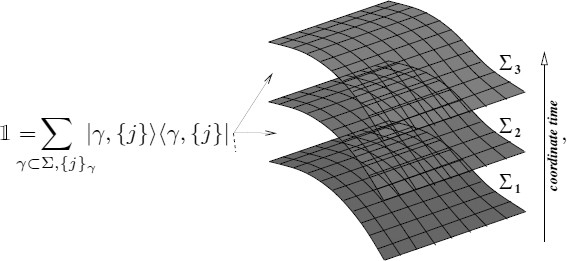(237)

where the sum is over the complete basis of spin-network states {|γ, {j}〉} — based on all graphs γ ∈ Σ and with all possible spin labeling — one arrives at a sum over a spin-network histories representation of 〈s,s′〉 p . More precisely, 〈s′,s p can be expressed as a sum over amplitudes corresponding to a series of transitions that can be viewed as the ‘time evolution’ between the ‘initial’ spin network s′ and the ‘final’ spin network s. This is illustrated in the two simple examples of Figure 12 and 14); on the r.h.s. we illustrate the continuum spin-foam picture obtained when the regulator is removed in the limit ϵ → 0.

Spin-network nodes evolve into edges, while spin-network links evolve into 2-dimensional faces. Edges inherit the intertwiners associated to the nodes and faces inherit the spins associated to the links. Therefore, the series of transitions can be represented by a 2-complex, whose 1-cells are labeled by intertwiners and whose 2-cells are labeled by spins. The places where the action of the plaquette loop operators create new links (Figure 13 and 14) define 0-cells or vertices. These foam-like structures are the spin foams. The spin-foam amplitudes are purely combinatorial and can be explicitly computed from the simple action of the loop operator in $${\mathcal H_{{\rm{kin}}}}$$.

The physical inner product takes the standard Ponzano-Regge form when the spin-network states s and s′ have only 3-valent nodes. Explicitly,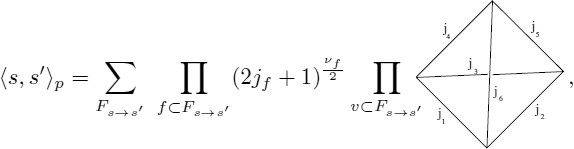(238)

where the sum is over all the spin foams interpolating between s and s′ (denoted Fss′, see Figure 18), fFss′ denotes the faces of the spin foam (labeled by the spins j f ), vF s→s′ denotes vertices, and ν f = 0 if fs ≠ 0 ∧ fs′ ≠ 0, ν f = 1 if fs ≠ 0 ∨ fs′ ≠ 0, and ν f = 2 if fs = 0 ∧ fs′ = 0. The tetrahedral diagram denotes a 6j-symbol: the amplitude obtained by means of the natural contraction of the four intertwiners corresponding to the 1-cells converging at a vertex. More generally, for arbitrary spin networks, the vertex amplitude corresponds to 3nj-symbols, and 〈s, s′ p takes the same general form.

Even though the ordering of the plaquette actions does not affect the amplitudes, the spin-foam representation of the terms in the sum (238) is highly dependent on that ordering. This is represented in Figure 15, where a spin-foam equivalent to that of Figure 12 is obtained by choosing an ordering of plaquettes where those of the central region act first. One can see this freedom of representation as an analogy of the gauge freedom in the spacetime representation in the classical theory.

In fact, one can explicitly construct a basis of $${\mathcal H_{{\rm{phys}}}}$$ by choosing a linearly-independent set of representatives of the equivalence classes defined in (231). One such basis is illustrated in Figure 16. The number of quantum numbers necessary to label the basis element is 6g − 6, corresponding to the dimension of the moduli space of SU(2) flat connections on a Riemann surface of genus g. This is the number of degrees of freedom of the classical theory. In this way we arrive at a fully combinatorial definition of the standard $${\mathcal H_{{\rm{phys}}}}$$ by reducing the infinite degrees of freedom of the kinematical phase space to finitely many by the action of the generalized projection operator P.

### Spin-foam quantization of 3D gravity

Here we apply the general framework of Sections 6 and 6.1.2. This was first studied by Iwasaki (in the spin-foam framework) in [229, 228]. The partition function, $$\mathcal{Z}$$, is formally given byFootnote 29

$${\mathcal Z} = \int {\mathcal D} [e]{\mathcal D}[A]\,\,\,{e^{i\int{}_{\mathcal M}\,{\rm{Tr}}[e \wedge F(A)]}},$$
(239)

where, for the moment, we assume $$\mathcal{M}$$ to be compact and orientable. Integrating over the e field in (239), we obtain

$${\mathcal Z} = \int{\mathcal D} [A]\,\,(\delta (A)){.}$$
(240)

The partition function $$\mathcal{Z}$$ corresponds to the ‘volume’ of the space of flat connections on $$\mathcal{M}$$.

In order to give meaning to the formal expressions above, we replace the 3-dimensional manifold $$\mathcal{M}$$ with an arbitrary cellular decomposition Δ. We also need the notion of the associated dual 2-complex of Δ denoted by Δ*. The dual 2-complex Δ* is a combinatorial object defined by a set of vertices v ∈ Δ* (dual to 3-cells in Δ), edges e ∈ Δ* (dual to 2-cells in Δ) and faces f ∈ Δ* (dual to 1-cells in Δ). The fields e and A have support on these discrete structures. The su(2)-valued 1-form field e is represented by the assignment of an esu(2) to each 1-cell in Δ. The connection field A is represented by the assignment of group elements g e SU(2) to each edge in Δ*.

The partition function is defined by

$${\mathcal Z}(\Delta) = \int {\prod\limits_{f \in \Delta {\ast}} {d{e_f}} \,\,\prod\limits_{e \in \Delta {\ast}} {d{e_e}\,\,{e^{i{\rm{Tr}}[{e_f},{U_f}]}},}}$$
(241)

where de f is the regular Lebesgue measure on ℝ3, dg e is the Haar measure on SU(2), and U f denotes the holonomy around faces, i.e., $${U_f} = g_e^1 \ldots g_e^N$$ for N being the number of edges bounding the corresponding face. Since U f SU(2), we can write it as $${U_f} = u_f^0\,1 + {F_f}$$ where $$u_f^0 \in \mathbb{C}$$ and F f su(2). F f is interpreted as the discrete curvature around the face f. Clearly Tr[e f U f ] = Tr[e f F f ]. An arbitrary orientation is assigned to faces when computing U f . We use the fact that faces in Δ* are in one-to-one correspondence with 1-cells in Δ and label e f with a face subindex.

Integrating over e f , we obtain

$${\mathcal Z}(\Delta) = \int {\,\,\prod\limits_{e \in \Delta *} {d{g_e}} \,\,\prod\limits_{f \in \Delta *} {\,\delta (g_e^1 \ldots g_e^N),}}$$
(242)

where δ corresponds to the delta distribution defined on $${\mathcal{L}^2}(SU(2))$$. Notice that the previous equation corresponds to the discrete version of Eq. (240).

The integration over the discrete connection (Π e dg e ) can be performed, expanding first the delta function in the previous equation using the Peter-Weyl decomposition

$$\delta (g) = \sum\limits_{j \in {\rm{irrep}}(SU(2))} {{\Delta _j}} \,{\rm{Tr}}\,[j(g)],$$
(243)

where Δ j = 2 j + 1 denotes the dimension of the unitary representation j, and j(g) is the corresponding representation matrix. Using Eq. (243), the partition function (242) becomes

$${\mathcal Z}(\Delta) = \sum\limits_{{\mathcal C}:\{j\} \rightarrow \{f\}} {\int {\prod\limits_{e \in \Delta ^{\ast}} {d{g_e}}}} \,\prod\limits_{f \in \Delta ^{\ast}} {{\Delta _{{j_f}}}} \,{\rm{Tr}}\,[{j_f}(g_e^1 \ldots g_e^N)],$$
(244)

where the sum is over coloring of faces in the notation of (86).

Going from Eq. (241) to (244), we have replaced the continuous integration over the e’s by the sum over representations of SU(2). Roughly speaking, the degrees of freedom of e are now encoded in the representation being summed over in (244).

Now it remains to integrate over the lattice connection {g e }. If an edge e ∈ Δ* bounds n faces there are n traces of the form Tr[j f (⋯ g e ⋯)] in (244) containing g e in the argument. The relevant formula is

$$P_{{\rm{inv}}}^n: = \int {dg} \,{j_1}(g) \otimes {j_2}(g) \otimes \cdots \otimes {j_n}(g) = \sum\limits_\iota {C_{{j_1}{j_2} \cdots {j_n}}^\iota C_{{j_1}{j_2} \cdots {j_n}}^{\ast\iota}},$$
(245)

where $$P_{{\rm{inv}}}^n$$ is the projector onto Inv[j1j2 ⊗ ⋯ ⊗ j n ]. On the right-hand side we have chosen an orthonormal basis of invariant vectors (intertwiners) to express the projector. Notice that the assignment of intertwiners to edges is a consequence of the integration over the connection. This is not a particularity of this example, but rather a general property of local spin foams. Finally, (242) can be written as a sum over spin-foam amplitudes

$${\mathcal Z}(\Delta) = \sum\limits_{{\mathcal C}:\{j\} \rightarrow \{f\}} {\,\sum\limits_{{\mathcal C}:\{\iota \} \rightarrow \{e\}} {\prod\limits_{f \in \Delta ^{\ast}} {{\Delta _{{j_f}}}}} \prod\limits_{v \in {{\mathcal J}_\Delta}} {\,{A_v}} ({\iota _v},{j_v})},$$
(246)

where A v (ι v , j v ) is given by the appropriate trace of the intertwiners ι v corresponding to the edges bounded by the vertex and j v are the corresponding representations. This amplitude is given, in general, by an SU(2) 3Nj-symbol corresponding to the flat evaluation of the spin network defined by the intersection of the corresponding vertex with a 2-sphere. When Δ is a simplicial complex, all the edges in $${{\mathcal J}_\Delta}$$ are 3-valent and vertices are 4-valent (one such vertex is emphasized in Figure 5, the intersection with the surrounding S2 is shown in dotted lines). Consequently, the vertex amplitude is given by the contraction of the corresponding four 3-valent intertwiners, i.e., a 6j-symbol. In that case, the partition function takes the familiar Ponzano-Regge  form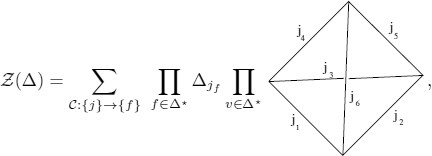(247)

where the sum over intertwiners disappears since dim(Inv[j1j2j3]) = 1 for SU(2) and there is only one term in (245). Ponzano and Regge originally defined the amplitude (247) from the study of the asymptotic properties of the 6j-symbol.

#### Discretization independence

A crucial property of the partition function (and transition amplitudes in general) is that it does not depend on the discretization Δ. Given two different cellular decompositions Δ and Δ′ (not necessarily simplicial)

$$\tau^{-n_0}Z(\Delta) = \tau^{-n_0^{\prime}}Z(\Delta{\prime}),$$
(248)

where n0 is the number of 0-simplexes in Δ (hence the number of bubbles in $${{\mathcal J}_\Delta}$$), and τ = ∑ j (2j + 1) is clearly divergent, which makes discretization independence a formal statement without a suitable regularization.

The sum over spins in (247) is typically divergent, as indicated by the previous equation. Divergences occur due to infinite volume factors corresponding to the topological gauge freedom (225) (see ).Footnote 30 The factor τ in (248) represents such a volume factor. It can also be interpreted as a δ(0) coming from the existence of a redundant delta function in (242). One can partially gauge fix this freedom at the level of the discretization. This has the effect of eliminating bubbles from the 2-complex.

In the case of simply connected Σ, the gauge fixing is complete. One can eliminate bubbles and compute finite transition amplitudes. The result is equivalent to the physical scalar product defined in the canonical picture in terms of the delta measure.Footnote 31

In the case of gravity with cosmological constant the state-sum generalizes to the Turaev-Viro model  defined in terms of SU q (2) with qn = 1, where the representations are finitely many. Heuristically, the presence of the cosmological constant introduces a physical infrared cutoff. Eq. (248) has been proven in this case for the case of simplicial decompositions in ; see also [363, 238]. The generalization for arbitrary cellular decomposition was obtained in .

#### Transition amplitudes

Transition amplitudes can be defined along similar lines using a manifold with boundaries. Given Δ, $${{\mathcal J}_\Delta}$$ then defines graphs on the boundaries. Consequently, spin foams induce spin networks on the boundaries. The amplitudes have to be modified concerning the boundaries to have the correct composition property (74). This is achieved by changing the face amplitude from (Δ jf ) to (Δjℓ)1/2 on external faces.

The crucial property of this spin-foam model is that the amplitudes are independent of the chosen cellular decomposition [363, 206]. This allows for computing transition amplitudes between any spin network states s = (γ, {j}, {ι}) and s′ = (γ, {j′}, {ι′}) according to the following rules:Footnote 32

• Given $${\mathcal M} = \Sigma \times [0,1]$$ (piecewise linear) and spin network states s = (γ, {j}, {ι}) and s′ = (γ, {j′}, {ι′}) on the boundaries — for γ and γ′ piecewise linear graphs in Σ — choose any cellular decomposition Δ such that the dual 2-complex $${{\mathcal J}_\Delta}$$ is bordered by the corresponding graphs γ and γ′ respectively (existence can be easily shown).

• Compute the transition amplitude between s and s′ by summing over all spin-foam amplitudes (rescaled as in (248)) for the spin foams F : ss′ defined on the 2-complex $${{\mathcal J}_\Delta}$$.

#### The generalized projector

We can compute the transition amplitudes between any element of the kinematical Hilbert space $${\mathcal H}$$.Footnote 33 Transition amplitudes define the physical scalar product by reproducing the skein relations of the canonical analysis. We can construct the physical Hilbert space by considering equivalence classes under states with zero transition amplitude with all the elements of $${\mathcal H}$$, i.e., null states.

Here we explicitly construct a few examples of null states. For any contractible Wilson loop in the j representation, the state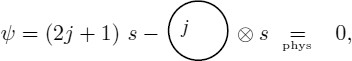(252)

for any spin network state s, has vanishing transition amplitude with any element of $${\mathcal H}$$. This can easily be checked by using the rules stated above and the portion of illustrated in Figure 17 to show that the two terms in the previous equation have the same transition amplitude (with opposite sign) for any spin-network state in $${\mathcal H}$$. Using the second elementary spin foam in Figure 17, one can similarly show that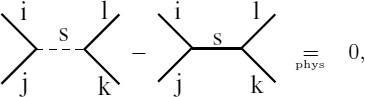(253)

or the re-coupling identity using the elementary spin foam on the right of Figure 17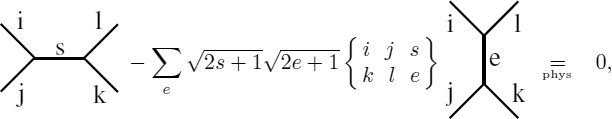(254)

where the quantity in brackets represents an SU(2) 6j-symbol. All skein relations can be found in this way. The transition amplitudes imply the skein relations that define the physical Hilbert space! The spin-foam quantization is equivalent to the canonical one.

#### The continuum limit

Recently, Zapata [372, 371] formalized the idea of a continuum spin-foam description of 3-dimensional gravity using projective techniques inspired by those utilized in the canonical picture . The heuristic idea is that, due to the discretization invariance one can define the model in an ‘infinitely’ refined cellular decomposition that contains any possible spin-network state on the boundary (this intuition is implicit in our rules for computing transition amplitudes above). Zapata concentrates on the case with non-vanishing cosmological constant and constructs the continuum extension of the Turaev-Viro model.

### Conclusion

We have illustrated the general notion of the spin-foam quantization in the simple case of three-dimensional Riemannian gravity (for the generalization to the Lorentzian case, see ). The main goal of the approach is to provide a definition of the physical Hilbert space. The example of this section sets the guiding principles of what one would like to realize in four dimensions. However, as should be expected, there are various new issues that make the task by far more involved.

### Further results in 3D quantum gravity

In this part of the article we have reviewed SU(2) BF theory from the perspective of classical and quantum gravity in three dimensions (for a classic reference see ). The state sum as presented above matches the quantum amplitudes first proposed by Ponzano and Regge in the 1960s, based on their discovery of the asymptotic expressions of the 6j-symbols , and is often referred to as the Ponzano-Regge model. Divergences in the above formal expression require regularization. We have seen in Section 14.3 that transition amplitudes are indeed finite in the canonical framework, where M = Σ × R. Natural regularizations are available in more general cases [67, 281, 186]. For a detailed study of the divergence structure of the model, see [100, 101, 102]. The quantum deformed version of the above amplitudes lead to the Turaev-Viro model , which is expected to correspond to the quantization of three-dimensional Riemannian gravity in the presence of a non-vanishing positive cosmological constant.

The topological character of BF theory can be preserved by the coupling of the theory with topological defects playing the role of point particles. In the spin-foam literature this has been considered from the canonical perspective in [279, 280] and extensively from the covariant perspective by Freidel and Louapre . These theories have been shown by Freidel and Livine to be dual, in a suitable sense, to certain non-commutative field theories in three dimensions [185, 184].

Concerning coupling BF theory with non-topological matter, see [172, 155] for the case of fermionic matter, and  for gauge fields. A more radical perspective for the definition of matter in 3D gravity is taken in . For three-dimensional supersymmetric BF theory models see [250, 36].

Recursion relations for the 6j vertex amplitudes have been investigated in [98, 157]. They provide a tool for studying dynamics in spin foams of 3D gravity and might be useful in higher dimensions .

## Conceptual issues and open problems

In the last part of this review we discuss some important conceptual issues that remain open questions to a large degree in the formulation. The description of the completely solvable model of Section 14 will serve as the main example to illustrate some of these issues.

## Quantum Spacetime and Gauge Histories

What is the geometric meaning of the spin-foam configurations? Can we identify the spin foams with “quantum spacetime configurations”? The answer to the above questions is, strictly speaking, in the negative, in agreement with our discussion at the end of Section 2.2. Physical degrees of freedom are extracted from the huge set of kinematical ones by the sum over gauge-histories that is realized in the spin-foam representation.

This conclusion is illustrated in an exact way by the simple example in 2+1 gravity where M = S2 × ℝ (g = 0) described in detail in Section 14. In this case, the spin-foam configurations appearing in the transition amplitudes look locally the same as those appearing in the representation of P for any other topology. However, a close look at the physical inner product defined by P permits one to conclude that the physical Hilbert space is one dimensional — the classical theory has zero degrees of freedom and so there is no non-trivial Dirac observable in the quantum theory. This means that the sum over spin foams in Eq. (238) is nothing but a sum over pure gauge degrees of freedom and hence no physical interpretation can be associated to it. The spins labeling the intermediate spin foams do not correspond to any measurable quantity. For any other topology this still holds true, the true degrees of freedom being of a global topological character. This means that, in general, (even when local excitations are present, as in 4D) the spacetime geometric interpretation of the spin-foam configurations is subtle. This is an important point that is often overlooked in the literature: one cannot interpret the spin-foam sum of Eq. (238) as a sum over geometries in any obvious way. Its true meaning instead comes from averaging over the gauge orbits generated by the quantum constraints that define P — recall the classical picture Figure 2, the discussion around Eq. (8), and the concrete implementation in 2+1, where U(N) in (229) is the unitary transformation representing the orbits generated by F. Spin foams represent a gauge history of a kinematical state. A sum over gauge histories is what defines P as a means for extracting the true degrees of freedom from those that are encoded in the kinematical boundary states.

Here we are discussing the interpretation of the spin-foam representation in the precise context of our solvable 3D example; however, the validity of the conclusion is of a general nature and holds true in the case of physical interest: four-dimensional LQG. Although, the quantum numbers labeling the spin-foam configurations correspond to eigenvalues of kinematical geometric quantities, such as length (in 2+1) or area (in 3+1) LQG, their physical meaning and measurability depend on dynamic considerations (for instance, the naive interpretation of the spins in 2+1 gravity as quanta of physical length is shown here to be of no physical relevance). Quantitative notions such as time or distance, as well as qualitative statements about causal structure or time ordering, are misleading (at best) if they are naively constructed in terms of notions arising from an interpretation of spin foams as quantum spacetime configurations.

Now this does not mean that kinematical features (that usually admit simpler geometric interpretation) are completely meaningless. It may be that such kinematical quantum geometric considerations might reflect true physical features when Dirac observables are considered. For instance, the simplicial geometry interpretation of spin-foam histories (e.g., the total area of a polyhedron associated to a spin network vertex) is the analog of the computation of some classical geometric quantity in general relativity (e.g., the area of some closed surface embedded in spacetime). Both the former and the latter have no intrinsic physical meaning: in the first case, as argued above, in the second case, because of the absent diffeomorphism-invariant characterization of the surface. However, in classical general relativity, the ability to compute the area of surfaces is useful to construct a physical meaningful quantity, such as the area of the event horizon in Schwarzschild spacetime, which is a fully-diffeomorphism invariant property of the BH spacetime. Similarly, all the machinery of quantum geometry (and its simplicial geometric interpretation in suitable cases) might play an important role in the construction of the physically-meaningful objects (Dirac observables) of quantum gravity.Footnote 34

It is well known (and can be illustrated by many simple examples) that gauge invariant properties can often be obtained by the suitable combination of gauge covariant quantities. For example, in Yang-Mills theory, the holonomy h(γ AB ) ∈ G from a point A to a point B along a path γ AB connecting the two points is not physically meaningful. Nor is the value of some field multiplets Ψ(A) and Ψ(B) at points A and B respectively. A physically-meaningful quantity arises from the gauge-invariant combination of the above quantities, namely 〈Ψ(A)h(γ AB )Ψ(B)〉. The pure gauge character of the quantum geometric interpretation pointed out above in the simple 3D context corresponds to the holonomy h(γ AB ) ∈ G in the above analogy. As soon as we couple 2+1 gravity with matter fields (corresponding to the Ψ in the above analogy) non-trivial Dirac observables become available (in fact such theory would have local degrees of freedom). These will have to encode the relationship between the gauge-dependent geometry and the gauge dependent field content in a gauge-invariant fashion.

In four dimensions we do not need to add anything, as gravity already has its local degrees of freedom, yet physically-meaningful questions are still relational. Unfortunately, no background-independent quantum theory with local degrees of freedom is sufficiently simple to illustrate this with an example. There is no lucky example of free quantum field theories for standard QFT (non-linearity and background independence may go hand-in-hand). In four dimensions, and in particular, in the case of the spin-foam models described in this review, the difficult question of extracting physics from the models is very important and presents a major challenge for the future. The references discussed in Section 13.3 are important encouraging steps in this direction.

## Anomalies and Gauge Fixing

As we mentioned before, and illustrated with the example of three-dimensional gravity (see discussion in Section 15), the spin-foam path integral is meant to provide a definition of the physical Hilbert space. Spin-foam transition amplitudes are not interpreted as defining propagation in time, but rather as defining the physical scalar product. This interpretation of spin-foam models is the one consistent with general covariance. However, in the path-integral formulation, this property relies on the gauge invariance of the path-integral measure. If the measure meets this property, we say it is anomaly free. It is well known that, in addition to the invariance of the measure, one must provide appropriate gauge-fixing conditions for the amplitudes to be well defined. In this section we analyze these issues in the context of the spin-foam approach.

Since we are interested in gravity in the first-order formalism, in addition to diffeomorphism invariance one has to deal with gauge transformations in the internal space. Let us first describe the situation for the latter. If this gauge group is compact, then anomaly free measures are defined using appropriate variables and invariant measures. In this case, gauge fixing is not necessary for the amplitudes to be well defined. Examples where this happens are: the models of Riemannian gravity considered in this paper (the internal gauge group being SO(4) or SU(2)), and standard lattice gauge theory. In these cases, one represents the connection in terms of group elements (holonomies) and uses the (normalized) Haar measure in the integration. In the Lorentzian sector (internal gauge group SL(2, ℂ)), the internal gauge orbits have infinite volume and the lattice path integral would diverge without an appropriate gauge fixing condition. These conditions generally exist in spin-foam models and are used to regularize the vertex amplitudes of the Lorentzian models (we have illustrated this at the end of Section 8.3; for a general treatment see ).

The remaining gauge freedom is diffeomorphism invariant. To illustrate this issue, we concentrate on the case of a model defined on a fixed discretization Δ, as it is the usual setting for calculations dealing with the models in four dimensions.

Let us start by considering the spin network states, at Δ*: boundary of Δ*, for which we want to define the transition amplitudes. According to what we have learned from the canonical approach, 3-diffeomorphism invariance is implemented by considering (diffeomorphism) equivalence classes of spin-network states. In the context of spin foams, the underlying discretization Δ restricts the graphs on the boundary to be contained on the dual 1-skeleton of the boundary complex Δ*. These states are regarded as representative elements of the corresponding 3-diffeomorphism equivalence class. The discretization can be interpreted, in this way, as a gauge fixing of 3-diffeomorphisms on the boundary. This gauge fixing is partial in the sense that, generically, there will remain a discrete symmetry remnant given by the discrete symmetries of the spin network. This remaining symmetry has to be factored out when computing transition amplitudes (in fact this also plays a role in the definition of the kinematical Hilbert space of LQG).

A natural view point (consistent with LQG and quantum geometry) is that this should naturally generalize to 4-diffeomorphisms for spin foams. The underlying 2-complex $${{\mathcal J}_\Delta}$$ on which spin foams are defined represents a partial gauge fixing for the configurations (spin foams) entering in the path integral. The remaining symmetry, to be factored out in the computation of transition amplitudes, corresponds simply to the finite group of discrete symmetries of the corresponding spin foams.Footnote 35 This factorization is well defined, since the number of equivalent spin foams can be characterized in a fully combinatorial manner, and is finite for any spin foam defined on a finite discretization. In addition, a spin-foam model is anomaly free if the amplitudes are invariant under this discrete symmetry. We have seen that this requirement (advocated in ) can be met by suitable definitions of the transition amplitudes [49, 235] (see Section 7.5).

However, it is expected — from the experience in lower-dimensional background-independent models (recall the discussion of Section 14) — that there will be remnants of the gauge symmetries acting non-trivially on spin-foam histories by doing more than simply changing the embedding in the discrete regulating structure Δ as described above. After all, in gravity the Hamiltonian constraint generates gauge transformations that hide in themselves the non-trivial dynamics of the theory. This is illustrated precisely in the simplest scenario of 3D gravity, where it is well known that bubble divergences are directly linked to the infinite volume of the gauge orbits generated by the curvature constraint . Notice that such gauge-symmetry-related spin foams do not differ only by their embedding (see Section 14).

It is also important to point out that the view that spin foams represent diffeomorphism equivalence classes of geometries, which sometimes can be loosely stated in the community, is incompatible with the idea that spin foams should act as a projector onto the solutions of constraints. A path integral would only act as a projector if it includes gauge symmetries in the sense of group averaging. This is related to the fact that physical states (those left invariant by the projector) are outside the kinematical Hilbert space, i.e., non-normalizable.

The discretization of the manifold Δ is seen as a regulator introduced to define the spin-foam model. Even when the regulator (or the discretization dependence) eventually has to be removed (see next Section 17), the theory is presumed to remain discrete at the fundamental level. The smooth-manifold diffeomorphism-invariant description is expected to emerge in the (low energy) continuum limit. Fundamental excitations are intrinsically discrete. From this viewpoint, the precise meaning of the gauge symmetries of such a theory would have to be formulated directly at the discrete level. We have seen that this can be achieved in the case of 3-dimensional gravity (recall Section 14.5.1).

The previous paragraph raises the question of whether the discretization procedures used in the derivation of the spin foams are compatible with the expectation that one is approximating a diffeomorphism-invariant fundamental theory. More precisely, can one tell whether the diffeomorphism invariance is broken by our regularization procedure. This question is quite an important one and has been one of the central concerns of the work led by Dittrich and collaborators for the last few years [151, 147, 150, 144, 43]. Indeed, there exist possible discretizations of a field theory, which maintain the full symmetry content of their continuum relatives. These regularizations are called perfect actions and even when they are difficult to construct explicitly in general cases, there are, in principle, methods (based on renormalization group ideas) to approach them [144, 44] (see also  and  for more recent discussion and explicit calculations in the perturbative context).

However, there are indications that the discretization procedures used generally do break the general covariance of gravity. In the simple case of 3D gravity with cosmological constant, this can be shown explicitly at the classical level  as well as at the quantum level . In the first reference it is shown nonetheless that there exists a well-defined perfect action in this case. At the quantum level, the results of  indicate a possible resolution of the anomaly issue. In four dimensions, this was first validated by the results obtained by Gambini and Pullin et al. in the consistent-discretization approach [190, 199, 198, 197, 196, 137, 138, 195, 191, 194]. They study the canonical formulation of theories defined on a lattice from the onset. The consistent discretization approach provides a way to analyze the meaning of gauge symmetries directly à la Dirac. Their results indicate that diff-invariance is indeed broken by the discretization in the sense that there is no infinitesimal generator of diffeomorphism. This is consistent with the covariant picture of discrete symmetries above. In their formulation the canonical equations of motion fix the value of what were Lagrange multipliers in the continuum (e.g., lapse and shift). This is interpreted as a breaking of diffeomorphism invariance; however, the solutions of the multiplier equations are highly non-unique. The ambiguity in selecting a particular solution corresponds to the remnant diffeomorphism invariance of the discrete theory. More recently, the issue of the breaking of the diffeomorphism symmetry by the regularizations used in spin foams has been studied by the Dittrich group (see references above). The possibility of using very simple models to study these questions has been opened in .

An important question is whether the possible breaking of the diffeomorphism gauge symmetry by the regularization can be made to disappear in the continuum limit. The breaking of a gauge symmetry implies the existence of a new host of degrees of freedom that might be in conflict with the low-energy interaction one is trying to recover in that limit.Footnote 36 In addition, the breaking of non-compact symmetries by a regulating structure poses serious difficulties for the low-energy regime, even in the case of global symmetries  (see  and  for a recent discussion). We mention this point here because it is, in our opinion, of major importance. Even though the question seems quite difficult to address in the context of the present models at this stage, it must be kept in mind in search of opportunities of further insights.

However, the notion of anomaly freeness evoked at the beginning of this section should be strengthened. In fact, according to our tentative definition, an anomaly-free measure can be multiplied by any gauge invariant function and yield a new anomaly free measure. This kind of ambiguity is not wanted; however, it is, in fact, present in most of the spin-foam models defined so far: In standard QFT theory, the formal (phase space) path integral measure in the continuum has a unique meaning (up to a constant normalization) emerging from the canonical formulation. Provided an appropriate gauge fixing, the corresponding Dirac bracket determines the formal measure on the gauge-fixed-constraint surface. In the discussion in Section 12.1 we have provided references where this issue is analyzed.

## Discretization Dependence

The spin-foam models we have introduced so far are defined on a fixed-cellular decomposition of $${\mathcal M}$$. This is to be interpreted as an intermediate step toward a definition of the theory. The discretization reduces the infinite dimensional functional integral to a multiple integration over a finite number of variables. This cutoff is reflected by the fact that only a restrictive set of spin foams (spin network histories) is allowed in the path integral: those that can be obtained by all possible coloring of the underlying 2-complex. In addition, it restricts the number of possible 3-geometry states (spin network states) on the boundary by fixing a finite underlying boundary graph. This represents a truncation in the allowed fluctuations and the set of states of the theory playing the role of a regulator. However, the nature of this regulator is fundamentally different from the standard concept in the background-independent framework: since geometry is encoded in the coloring (that can take any spin values) the configurations involve fluctuations all the way to Plank scale.Footnote 37 This scenario is different in lattice gauge theories where the lattice introduces an effective UV cutoff given by the lattice spacing. However, transition amplitudes are discretization dependent now. A consistent definition of the path integral using spin foams should include a prescription to eliminate this discretization dependence.

A special case is that of topological theories, such as gravity in 3 dimensions. In this case, one can define the sum over spin foams with the aid of a fixed cellular decomposition Δ of the manifold. Since the theory has no local excitations (no gravitons), the result is independent of the chosen cellular decomposition. A single discretization suffices to capture the degrees of freedom of the topological theory.

In lattice gauge theory, the solution to the problem is implemented through the continuum limit. In this case, the existence of a background geometry is crucial, since it allows one to define the limit when the lattice constant (length of links) goes to zero. In addition, the possibility of working in the Euclidean regime allows the implementation of statistical mechanical methods.

None of these structures are available in the background-independent context. The lattice (triangulation) contains only topological information and there is no geometrical meaning associated to its components. As mentioned above, this has the novel consequence that the truncation cannot be regarded as a UV cutoff, as in the background-dependent context. This, in turn, represents a conceptual obstacle to the implementation of standard techniques. Moreover, no Euclidean formulation seems meaningful in a background-independent scenario. New means to eliminate the truncation introduced by the lattice need to be developed. For a recent analysis in which the difference between lattice regularization of background-dependent versus background-independent theories is carefully considered, see . For a more general discussion, see .

This is a major issue where concrete results have not been obtained so far beyond the topological case. Here we explain the two main approaches to recover general covariance corresponding to the realization of the notion of ‘summing over discretizations’ of .

• Refinement of the discretization:

According to this idea, topology is fixed by the simplicial decomposition. The truncation in the number of degrees of freedom should be removed by considering triangulations of increasing number of simplexes for that fixed topology. The flow in the space of possible triangulations is controlled by the Pachner moves. The idea is to take a limit in which the number of four simplexes goes to infinity together with the number of tetrahedra on the boundary. Given a 2-complex $${{\mathcal J}_2}$$, which is a refinement of a 2-complex $${{\mathcal J}_1}$$, then the set of all possible spin foams defined on $${{\mathcal J}_1}$$ is naturally contained in those defined on $${{\mathcal J}_2}$$. The refinement process should also enlarge the space of possible 3-geometry states (spin networks) on the boundary recovering the full kinematical sector in the limit of infinite refinements. An example where this procedure is well defined is Zapata’s treatment of the Turaev-Viro model . The key point in this case is that amplitudes are independent of the discretization (due to the topological character of the theory), so that the refinement limit is trivial. In the general case, the definition of the refinement limit has recently been studied and formalized in . There is no evidence that the amplitudes of any of the present models for 4D converge in such a limit.

It has often been emphasized that such a refinement limit may be studied from the Wilsonian renormalization view point. In the past a renormalization approach for spin foams has been proposed by Markopoulou [266, 265]. Also, Oeckl  has studied the issue of renormalization in the context of spin-foam models containing a coupling parameter. These models include generalized covariant gauge theories [284, 306, 291], the Reisenberger model, and the interpolating model (defined by Oeckl). The latter is given by a one-parameter family of models that interpolate between the trivial BF topological model and the Barrett-Crane model according to the value of a ‘coupling constant’. Qualitative aspects of the renormalization groupoid flow of the couplings are studied in the various models.

• Spin foams as Feynman diagrams:

This idea has been motivated by the generalized matrix models of Boulatov and Ooguri [105, 292]. The fundamental observation is that spin foams admit a dual formulation in terms of a field theory over a group manifold [133, 315, 314]. The duality holds in the sense that spin-foam amplitudes correspond to Feynman diagram amplitudes of the GFT. The perturbative Feynman expansion of the GFT (expansion in a fiducial coupling constant λ) provides a definition of sum over discretizations, which is fully combinatorial and hence independent of any manifold structure.Footnote 38 The latter is the most appealing feature of this approach.

However, the convergence issues clearly become more involved. The perturbative series are generically divergent. This is not necessarily a definite obstruction as divergent series can often be given an asymptotic meaning and provide physical information. Moreover, there are standard techniques that one can allow to ‘re-sum’ a divergent series in order to obtain non-perturbative information . Freidel and Louapre  have shown that this is indeed possible for certain GFT’s in three dimensions. Other possibilities have been proposed in .

Diffeomorphism-equivalent configurations (in the discrete sense described above) appear at all orders in the perturbation series.Footnote 39 From this perspective (and leaving aside the issue of convergence) the sum of different order amplitudes corresponding to equivalent spin foams should be interpreted as the definition of the physical amplitude of that particular spin foam. The discussion of Section 16 does not apply in the GFT formulation, i.e., there is no need for gauge fixing.

The GFT formulation could resolve by definition the two fundamental conceptual problems of the spin-foam approach: diffeomorphism gauge symmetry and discretization dependence. The difficulties are shifted to the question of the physical role of Δ and the convergence of the corresponding perturbative series. A prescription that avoids this last issue is the radical proposal of Freidel , where the tree-level GFT amplitudes are used to define the physical inner product of quantum gravity.

This idea has been studied in more detail in three dimensions. In  scaling properties of the modification of the Boulatov group field theory introduced in  were studied in detail. In a further modification of the previous model (known as colored tensor models ) new techniques based on a suitable 1/N expansion imply that amplitudes are dominated by spherical topology ; moreover, it seem possible that the continuum limit might be critical, as in certain matrix models [209, 95, 210, 211, 339]. However, it is not yet clear if there is a sense in which these models correspond to a physical theory. The appealing possible interpretation of the models is that they correspond to a formulation of 3D quantum gravity including a dynamical topology. Not much is known about gravity models in four dimensions at this stage.

Finally, discretization independence is not only a problem of spin foams but also of Regge gravity. Even linearized classical Regge calculus is not discretization independent in 4D as shown in , where a general discussion of the issues raised in this section are considered. It is also natural to expect the issue of discretization independence to be connected to the issue of diffeomorphism symmetry discussed in Section 16. There is evidence [46, 146, 145] that the implementation of diffeomorphism symmetry into the models will lead to discretization independence.

## Lorentz Invariance in the Effective Low-Energy Regime

Finally, a very important question for LQG and spin foams (closely related to the consistency of their low energy limit) is that of the fate of Lorentz symmetry in the low energy regime. This question has received lots of attention in recent years as it seems to provide an open window of opportunity for observations of quantum gravity effects (see [270, 233] and references therein). In the context of LQG, a systematic first-principles analysis of such properties of the low-energy regime remains open. This is mainly due to the presently-unsolved difficulties associated with a quantitative dynamical description of physical states. The spin-foam approach discussed in this review is meant to address the dynamical question and the definition of the new models may provide the necessary insights to tackle the relevant aspects for the question of Lorentz invariance at low energies. This question is perhaps within reach at the present stage of development of the theory, so it deserves all the attention in future investigations.

In the absence of detailed formulations, certain early pioneering model calculations  (see also general treatment [340, 341]) indicate the possibility for certain Lorentz-invariance-violation (LIV) effects associated to a quantum-gravity granularity of spacetime measured at rest by a preferred frame of observers. The effects take the form of corrections to the effective Lagrangian with LIV terms of dimension 5 or higher that are suppressed by negative powers of the Planck mass. This makes them, in principle, negligible at low energies so that the potential quantum-gravity effects would be observed as corrections to the standard QFT well-tested physics. However, it was soon realized  that the framework of effective field theories severely restricts the possibility that LIV remains small when interactions in QFT are taken into account. In fact, dimension five or higher LIV terms will generically (in the absence of some fundamental protecting mechanism) generate dimension four and lower LIV terms (with factors in front that are at best quadratic in standard model coupling constants). Such unsuppressed contributions are in flagrant conflict with the observed Lorentz invariance of particle physics at low energies.

Therefore, the early calculations in LQG cited above need to be revised under the light of the strong constraints on the way LIV can arise in quantum gravity. A logical possibility is that these LIV terms are there thanks to the protecting effect of some custodial mechanism restricting the LIV to dimension 5 and higher operators.Footnote 40 For example, such a possibility has been considered in , where it is shown that the large LIV effects can be avoided in the context of Euclidean field theory (the protecting mechanism being here the trading of the non-compact Poincaré group by the Euclidean group). This example is interesting but unfortunately does not provide an answer to the question for the physical Lorentzian theory (see discussion in  and more recently ).

Thus, there is a challenge for LQG and spin foams consisting of showing that the low-energy limit of the theory is compatible with the observed low-energy LI. This can be put in the form of two conservative possibilities. The description of the low energy physics in terms of

1. 1.

an effective action with no LIV terms, or

2. 2.

an effective action where LIV terms appear starting from dimension-five operators on, due to some fundamental protecting mechanism in LQG for such structure to be stable under radiative corrections.

My view, based on the ignorance of a suitable protecting mechanism in LQG, is that only the first possibility is sensible. This would mean that the way the fundamental Planckian discreteness of LQG must enter physics is through reference-frame-independent phenomena (as mass enters a Klein-Gordon theory). In my opinion, the main ingredient that must be taken into account in considering this issue is the construction of the low-energy limit of quantum gravity in terms of physical or gauge invariant notions (see discussion in Section 15). The LIV effects obtained in LQG so far promote by assumption the kinematical discreteness of quantum geometry to discreteness of the physical background in a literal fashion (on the one hand, discreteness of geometry can coexist with Lorentz invariance , on the other, at the level of gauge-invariant observables, some discrete aspects could disappear ). The limitations of such naive view point on kinematical discreteness versus physical properties in the theory is, in my view, best manifested in the setting of pure 3D gravity, as discussed above.

For completeness we add that, in addition to the above (conservative) possibilities, some less standard scenarios could be realized. This has been the view of some of the quantum gravity community. For a recent proposal of a completely new theoretical framework see  and references therein.

1. 1.

In principle, one can avoid this by carefully devising a theory of perturbations for generally covariant systems. For some ideas on this, see [153, 239]

2. 2.

For instance, a typical example is to use a photon to measure distance. The energy of the photon in our lab frame is given by E γ = hc/λ. We put the photon in a cavity and produce a standing wave measuring the dimensions of the cavity in units of the wavelength. The best possible precision is attained when the Schwarzschild radius corresponding to energy of the photon is on the order of its wavelength. Beyond that the photon can collapse to form a black hole around some of the maxima of the standing wave. This happens for a value λ c for which λ c GE γ /c4 = hG/(λ c c3). The solution is $${\lambda _c} \approx \sqrt {hG/{c^3}}$$, which is the Planck length

3. 3.

Interestingly, and as a side remark, the structure of the phase space of gravity with boundaries used in the description of concrete physical models seems to provide an extra justification for the use of the holonomy-flux variables (see Section IV E in  for a more detailed discussion of this intriguing fact)

4. 4.

Most (if not all) of the applications of general relativity make use of this concept together with the knowledge of certain exact solutions. In special situations there are even preferred coordinate systems based on this notion, which greatly simplify interpretation (e.g., co-moving observers in cosmology, or observers at infinity for isolated systems)

5. 5.

In this case, one would need first to solve the constraints of general relativity in order to find the initial data representing the self-gravitating objects. Then one would have essentially two choices: 1) Fix a lapse N(t) and a shift Na(t), evolve with the constraints, obtain a spacetime (out of the data) in a particular gauge, and finally ask the diff-invariant-question; or 2) try to answer the question by simply studying the data itself (without t-evolution). It is far from obvious whether the first option (the conventional one) is any easier than the second

6. 6.

In order to make a Planck scale observation, we need a Planck energy probe (think of a Planck energy photon). It would be absurd to suppose that one can disregard the interaction of such a photon with the gravitational field, treating it as a test photon

7. 7.

Notice that (for example) the right invariant vector field $${{\mathcal J}^i}(U) = {\sigma ^i}{}_B^AU{}_C^B\partial/\partial U{}_C^A$$ has a well-defined action at the level of Eq. (244) and acts as a B operator at the level of (241) since

$$- i{\chi ^i}(U)\left[ {{e^{i{\rm{Tr}}[Bu]}}} \right]{{\vert}_{U\sim1}} = {\rm{Tr}}[{\sigma ^i}UB]{e^{i{\rm{Tr}}[BU]}}{{\vert}_{U\sim1}}\sim{B^i}{e^{i{\rm{Tr}}[BU]}},$$
(11)

where σi are Pauli matrices

8. 8.

This difficulty also arises in the Barrett-Crane model, as we shall see in Section 10

9. 9.

Iwasaki defines another model involving multiple cellular complexes to provide a simpler representation of wedge products in the continuum action. A more detailed presentation of this model would require the introduction of various technicalities at this stage, so we refer the reader to 

10. 10.

The six components BIJ are associated to invariant vector fields in the Lorentz group as a direct consequence of the discretization procedure in spin foams. This makes them non-commutative and render the simplicity constraints (functionals of the B field alone) non commutative. The origin of the non-commutativity of B fields is similar to the non-commutativity of fluxed in canonical LQG 

11. 11.

12. 12.

I am grateful to M. Montesinos and M. Velázquez for discussions and contributions to the present section

13. 13.

In fact, Eq. (36) implies that $$\Gamma _a^i[E] = \Gamma _a^i[\lambda E]$$ for a constant λ. This homogeneity property plus the existence of W[E] implies that $$W[E] = \int {E_i^a\Gamma _a^i}$$, as can be verified by direct calculation

14. 14.

The constraint structure does not distinguish SO(3) from SU(2), as both groups have the same Lie algebra. From now on we choose to work with the more fundamental (universal covering) group SU(2). In fact, this choice is physically motivated as SU(2) is the gauge group, if we want to include fermionic matter 

15. 15.

In the physics of the standard model we are used to identifying the coordinate t with the physical time of a suitable family of observers. In the general covariant context of gravitational physics, the coordinate time t plays the role of a label with no physical relevance. One can arbitrarily change the way we coordinatize spacetime without affecting the physics. This redundancy in the description of the physics (gauge symmetry) induces the appearance of constraints in the canonical formulation. The constraints in turn are the generating functions of these gauge symmetries. The Hamiltonian generates evolution in coordinate time t, but because redefinition of t is pure gauge, the Hamiltonian is a constraint itself, i.e., $${\mathcal H} = 0$$ on shell [143, 223]

16. 16.

The construction is rather a projective limit . This leads to non-trivial requirements (cylindrical consistency) on the structure (operators) of the theory. This point is very important for spin foams, especially in considering the refining limit of amplitudes. We will revisit this issue in Section 7.5

17. 17.

Spin-networks were introduced by Penrose [296, 297, 299, 298] in a attempt to define 3-dimensional Euclidean quantum geometry from the combinatorics of angular momentum in quantum mechanics. Independently they have been used in lattice gauge theory as a natural basis for gauge invariant functions on the lattice. For an account of their applications in various contexts see 

18. 18.

According to the triple $$Cyl \subset {\mathcal H} \subset Cyl^{\ast}$$

19. 19.

The Imirzi parameter ι is a free parameter in the theory. This ambiguity is purely quantum mechanical (it disappears in the classical limit). It has to be fixed in terms of physical predictions. The computation of black-hole entropy in LQG fixes the value of γ (see )

20. 20.

However, there is an additional complication here: the canonical constraint algebra does not reproduce the 4-diffeomorphism Lie algebra. This complicates the geometrical meaning of S

21. 21.

This is not the case in all the available definitions of the scalar constraints as, for example, the one defined in [135, 136]

22. 22.

The role of category theory for quantum gravity had been emphasized by Crane in [128, 127, 130]

23. 23.

In the notation of Section 4, states in $${\mathcal H_{{\rm{phys}}}}$$ are elements of Cyl*

24. 24.

It is important to point out that the integration over the algebra-valued B field does not exactly give the group delta function. For instance, in the simple case where G = SU(2) with Bsu(2), integration leads to the SO(3) delta distribution (which only contains integer spin representations in the mode expansion (85)). Generally, one ignores this fact and uses the G-delta distribution in the models found in the literature

25. 25.

This is obviously not clear from the form of (167). We are extrapolating the properties of $${(P_{{\rm{EPRL}}}^e)^N}$$ for large N to those of the amplitude (167) in the large number of edges limit implied by the continuum limit

26. 26.

The importance of such a formulation of the constraints was emphasized before by Alexandrov

27. 27.

The definition of polymer-like gauge-invariant states as elements of a Hilbert space of a gauge theory with non-compact group is an open issue. The naive attempts fail, the basic problem is that gauge-invariant states are not square integrable functions with respect to the obvious generalization of the AL measure for a fixed graph: this is due to the infinite volume of the gauge group (the invariant measure is not normalizable). For that reason the discussion of the Lorentzian sector with G = SL(2, ℂ) is usually formal. See  for some insights on the problem of defining a gauge-invariant Hilbert space of graphs for non-compact gauge groups

28. 28.

The physical inner product between spin-network states satisfies the following inequality

$${\vert}{\langle s,s{\prime}\rangle _p}{\vert}\, \leq C\sum\limits_j {{{(2j + 1)}^{2 - 2g}}},$$

for some positive constant C. The convergence of the sum for genus g ≥ 2 follows directly. The case of the sphere g = 0 and the torus g = 1 can be treated individually 

29. 29.

We are dealing with Riemannian 3-dimensional gravity. This should not be confused with the approach of Euclidean quantum gravity formally obtained by a Wick rotation of Lorentzian gravity. Notice the imaginary unit in front of the action. The theory of Riemannian quantum gravity should be regarded as a toy model with no obvious connection to the Lorentzian sector

30. 30.

For simplicity we concentrate on the Abelian case G = U(1). The analysis can be extended to the non-Abelian case. Writing gU(1) as g = e, the analog of the gravity simplicial action is

$$S(\Delta, \{{e_f}\}, \{{\theta _e}\}) = \sum\limits_{f \in \Delta ^{\ast}} {{e_f}{F_f}(\{{\theta _e}\})},$$
(249)

where F f ({θ e }) = ∑ e∈f θ e . Gauge transformations corresponding to (224) act at the end points of edges $$e \in {{\mathcal J}_\Delta}$$ by the action of group elements {β} in the following way

$$\begin{array}{*{20}c} {{e_f} \rightarrow {e_f},\quad \quad \quad \,\,} \\ {{\theta _e} \rightarrow {\theta _e} + {\beta _s} - {\beta _t},} \\ \end{array}$$
(250)

where the sub-index s (respectively t) labels the source vertex (respectively target vertex) according to the orientation of the edge. The gauge invariance of the simplicial action is manifest. The gauge transformation corresponding to (225) acts on vertices of the triangulation Δ and is given by

$$\begin{array}{*{20}c} {{B_f} \rightarrow {B_f} + {\eta _s} - {\eta _t},} \\ {{\theta _e} \rightarrow {\theta _e}{.}\quad \quad \quad \quad} \\ \end{array}$$
(251)

According to the discrete analog of Stokes’ theorem,

$$\sum\limits_{f \in {\rm{Bubble}}} {{F_f}} (\{{\theta _e}\}) = 0,$$

which implies the invariance of the action under the transformation above. The divergence of the corresponding spin-foam amplitudes is due to this last freedom. Alternatively, one can understand it from the fact that Stokes’ theorem implies a redundant delta function in (242) per bubble in $${{\mathcal J}_\Delta}$$

31. 31.

If $${\mathcal M} = {S^2} \times [0,1]$$ one can construct a cellular decomposition interpolating any two graphs on the boundaries without having internal bubbles and hence no divergences

32. 32.

Here we are ignoring various technical issues in order to emphasize the relevant ideas. The most delicate is that of the divergences due to gauge factors mentioned above. For a more rigorous treatment, see [372, 371]

33. 33.

The sense in which this is achieved should be apparent from our previous definition of transition amplitudes. For a rigorous statement, see [372, 371]

34. 34.

An interesting example, where this is strictly realized, is the midi-superspace formulation of black holes in the context of LQG known as the isolated-horizon formulation (see  for a review). In this framework the area of what represents the black-hole horizon is a Dirac observable and has a discrete spectrum inherited from the area spectrum of the kinematical area in the full theory . Such discreteness is crucial in recovering the Bekenstein-Hawking area law for the black-hole entropy in LQG [170, 25]

35. 35.

Baez  points out this equivalence relation between spin foams as a necessary condition for the definition of the category of spin foams

36. 36.

Breaking gauge symmetries comes hand-in-hand with introducing (unwanted) new degrees of freedom. For example, longitudinal photon modes would appear if U(1) gauge symmetry is violated in electromagnetism; similar spurious modes come to life if diffeormorphism invariance is broken in gravity theories

37. 37.

Changing the label of a face from j to j +1 amounts to changing an area eigenvalue by an amount on the order of the Planck length squared according to (71)

38. 38.

This is more than a ‘sum over topologies’ as many of the two-complexes appearing in the perturbative expansion cannot be associated to any manifold 

39. 39.

Already, the GFT formulation is clearly non-trivial in the case of topological theories. There have been attempts to make sense of the GFT formulation dual to BF theories in lower dimensions 

40. 40.

For an example of protecting symmetry, see ; however, notice that the non-compact nature of the protecting symmetry in this case makes it seemingly unsuitable for a sensible possibility in LQG.

## References

1. 

Alesci, E., Bianchi, E., Magliaro, E. and Perini, C., “Asymptotics of LQG fusion coefficients”, Class. Quantum Grav., 27, 095016, (2010). [DOI], [arXiv:0809.3718 [gr-qc]]. (Cited on page 65.)

2. 

Alesci, E., Bianchi, E. and Rovelli, C., “LQG propagator: III. The new vertex”, Class. Quantum Grav., 26, 215001, (2009). [DOI], [arXiv:0812.5018 [gr-qc]]. (Cited on page 83.)

3. 

Alesci, E., Noui, K. and Sardelli, F., “Spin-Foam Models and the Physical Scalar Product”, Phys. Rev. D, 78, 104009, (2008). [DOI], [arXiv:0807.3561]. (Cited on page 79.)

4. 

Alesci, E. and Rovelli, C., “Complete LQG propagator: Difficulties with the Barrett-Crane vertex”, Phys. Rev. D, 76, 104012, (2007). [DOI], [arXiv:0708.0883 [gr-qc]]. (Cited on page 83.)

5. 

Alesci, E. and Rovelli, C., “Complete LQG propagator. II. Asymptotic behavior of the vertex”, Phys. Rev. D, 77, 044024, (2008). [DOI], [arXiv:0711.1284 [gr-qc]]. (Cited on page 83.)

6. 

Alexander, S., Marcianó, A. and Tacchi, R.A., “Towards a Spin-foam unification of gravity, Yang-Mills interactions and matter fields”, Phys. Lett. B, 716, 330–333, (2012). [DOI], [arXiv:1105.3480 [gr-qc]]. (Cited on page 79.)

7. 

Alexandrov, S., “SO(4, C)-covariant Ashtekar-Barbero gravity and the Immirzi parameter”, Class. Quantum Grav., 17, 4255–4268, (2000). [DOI], [arXiv:gr-qc/0005085]. (Cited on page 78.)

8. 

Alexandrov, S., “Choice of connection in loop quantum gravity”, Phys. Rev. D, 65, 024011, (2002). [DOI], [arXiv:gr-qc/0107071]. (Cited on page 78.)

9. 

Alexandrov, S., “Hilbert space structure of covariant loop quantum gravity”, Phys. Rev. D, 66, 024028, (2002). [DOI], [arXiv:gr-qc/0201087]. (Cited on page 78.)

10. 

Alexandrov, S., “Reality conditions for Ashtekar gravity from Lorentz-covariant formulation”, Class. Quantum Grav., 23, 1837–1850, (2006). [DOI], [arXiv:gr-qc/0510050]. (Cited on page 78.)

11. 

Alexandrov, S., “Simplicity and closure constraints in spin foam models of gravity”, Phys. Rev. D, 78, 044033, (2008). [DOI], [arXiv:0802.3389 [gr-qc]]. (Cited on page 68.)

12. 

Alexandrov, S., “Spin foam model from canonical quantization”, Phys. Rev. D, 77, 024009, (2008). [DOI], [arXiv:0705.3892 [gr-qc]]. (Cited on pages 21 and 68.)

13. 

Alexandrov, S., “The new vertices and canonical quantization”, Phys. Rev. D, 82, 024024, (2010). [DOI], [arXiv:1004.2260 [gr-qc]]. (Cited on pages 52 and 68.)

14. 

Alexandrov, S., “Degenerate Plebanski sector and its spin foam quantization”, Class. Quantum Grav., 29, 145018, (2012). [DOI], [arXiv:1202.5039 [gr-qc]]. (Cited on page 68.)

15. 

Alexandrov, S., Buffenoir, E. and Roche, P., “Plebanski theory and covariant canonical formulation”, Class. Quantum Grav., 24, 2809–2824, (2007). [DOI], [arXiv:gr-qc/0612071]. (Cited on page 78.)

16. 

Alexandrov, S., Geiller, M. and Noui, K., “Spin Foams and Canonical Quantization”, SIGMA, 8, 055, (2012). [DOI], [arXiv:1112.1961 [gr-qc]]. (Cited on page 36.)

17. 

Alexandrov, S., Grigentch, I. and Vassilevich, D.V., “SU(2)-invariant reduction of the (3+1)-dimensional Ashtekar’s gravity”, Class. Quantum Grav., 15, 573–580, (1998). [DOI], [arXiv:gr-qc/9705080]. (Cited on page 78.)

18. 

Alexandrov, S. and Krasnov, K., “Hamiltonian analysis of non-chiral Plebanski theory and its generalizations”, Class. Quantum Grav., 26, 055005, (2009). [DOI], [arXiv:0809.4763 [gr-qc]]. (Cited on page 23.)

19. 

Alexandrov, S. and Livine, E.R., “SU (2) loop quantum gravity seen from covariant theory”, Phys. Rev. D, 67, 044009, (2003). [DOI], [arXiv:gr-qc/0209105]. (Cited on page 78.)

20. 

Alexandrov, S. and Roche, P., “Critical Overview of Loops and Foams”, Phys. Rep., 506, 41–86, (2011). [DOI], [arXiv:1009.4475 [gr-qc]]. (Cited on page 17.)

21. 

Alexandrov, S. and Vassilevich, D.V., “Area spectrum in Lorentz covariant loop gravity”, Phys. Rev. D, 64, 044023, (2001). [DOI], [arXiv:gr-qc/0103105 [gr-qc]]. (Cited on page 78.)

22. 

Amelino-Camelia, G., Freidel, L., Kowalski-Glikman, J. and Smolin, L., “Principle of relative locality”, Phys. Rev. D, 84, 084010, (2011). [DOI], [arXiv:1101.0931 [hep-th]]. (Cited on page 106.)

23. 

Ashtekar, A., “New Variables for Classical and Quantum Gravity”, Phys. Rev. Lett., 57, 2244–2247, (1986). [DOI]. (Cited on pages 23 and 29.)

24. 

Ashtekar, A., Lectures on Non-Perturbative Canonical Gravity, Advanced Series in Astrophysics and Cosmology, 6, (World Scientific, Singapore, 1991). [Google Books]. (Cited on pages 22 and 29.)

25. 

Ashtekar, A., Baez, J.C., Corichi, A. and Krasnov, K., “Quantum geometry and black hole entropy”, Phys. Rev. Lett., 80, 904–907, (1998). [DOI], [arXiv:gr-qc/9710007]. (Cited on page 98.)

26. 

Ashtekar, A., Baez, J.C. and Krasnov, K., “Quantum geometry of isolated horizons and black hole entropy”, Adv. Theor. Math. Phys., 4, 1–94, (2000). [arXiv:gr-qc/0005126]. (Cited on pages 32 and 98.)

27. 

Ashtekar, A., Campiglia, M. and Henderson, A., “Loop Quantum Cosmology and Spin Foams”, Phys. Lett. B, 681, 347–352, (2009). [DOI], [arXiv:0909.4221]. (Cited on page 79.)

28. 

Ashtekar, A., Campiglia, M. and Henderson, A., “Casting Loop Quantum Cosmology in the Spin Foam Paradigm”, Class. Quantum Grav., 27, 135020, (2010). [DOI], [arXiv:1001.5147]. (Cited on page 79.)

29. 

Ashtekar, A., Campiglia, M. and Henderson, A., “Path Integrals and the WKB approximation in Loop Quantum Cosmology”, Phys. Rev. D, 82, 124043, (2010). [DOI], [arXiv:1011.1024]. (Cited on page 79.)

30. 

Ashtekar, A., Corichi, A. and Zapata, J.A., “Quantum theory of geometry: III. Non-commutativity of Riemannian structures”, Class. Quantum Grav., 15, 2955–2972, (1998). [DOI], [arXiv:gr-qc/9806041 [gr-qc]]. (Cited on page 20.)

31. 

Ashtekar, A. and Krishnan, B., “Isolated and Dynamical Horizons and Their Applications”, Living Rev. Relativity, 7, lrr-2004-10 (2004). [arXiv:gr-qc/0407042]. URL (accessed 12 September 2011): http://www.livingreviews.org/lrr-2004-10. (Cited on page 98.)

32. 

Ashtekar, A. and Lewandowski, J., “Projective techniques and functional integration”, J. Math. Phys., 36, 2170, (1995). [DOI]. (Cited on pages 13, 30, 31, and 94.)

33. 

Ashtekar, A. and Lewandowski, J., “Quantum Theory of Gravity: I. Area Operators”, Class. Quantum Grav., 14, A55–A81, (1997). [DOI]. (Cited on page 32.)

34. 

Ashtekar, A. and Lewandowski, J., “Quantum Theory of Gravity: II. Volume Operators”, Adv. Theor. Math. Phys., 1, 388–429, (1997). [arXiv:gr-qc/9711031]. (Cited on page 32.)

35. 

Ashtekar, A. and Lewandowski, J., “Background independent quantum gravity: A status report”, Class. Quantum Grav., 21, R53–R152, (2004). [DOI], [arXiv:gr-qc/0404018]. (Cited on pages 12, 14, 22, 28, and 29.)

36. 

Baccetti, V., Livine, E.R. and Ryan, J.P., “The particle interpretation of N = 1 supersymmetric spin foams”, Class. Quantum Grav., 27, 225022, (2010). [DOI], [arXiv:1004.0672 [gr-qc]]. (Cited on pages 44 and 95.)

37. 

Baez, J.C., “Spin Network States in Gauge Theory”, Adv. Math., 117, 253–272, (1996). [DOI]. (Cited on page 30.)

38. 

Baez, J.C., “Spin Foam Models”, Class. Quantum Grav., 15, 1827–1858, (1998). [DOI]. (Cited on pages 19, 34, 63, 80, and 99.)

39. 

Baez, J.C., “An Introduction to Spin Foam Models of Quantum Gravity and BF Theory”, in Gausterer, H., Grosse, H. and Pittner, L., eds., Geometry and Quantum Physics, Proceedings of the 38. Internationale Universitätswochen für Kern- und Teilchenphysik, Schladming, Austria, January 9–16, 1999, Lecture Notes in Physics, 543, pp. 25–94, (Springer, Berlin; New York, 2000). [gr-qc/9905087]. (Cited on pages 17, 37, and 85.)

40. 

Baez, J.C. and Barrett, J.W., “The Quantum Tetrahedron in 3 and 4 Dimensions”, Adv. Theor. Math. Phys., 3, 815–850, (1999). (Cited on page 19.)

41. 

Baez, J.C. and Perez, A., “Quantization of strings and branes coupled to BF theory”, Adv. Theor. Math. Phys., 11, 3, (2007). [arXiv:gr-qc/0605087]. (Cited on page 46.)

42. 

Bahr, B., “On knottings in the physical Hilbert space of LQG as given by the EPRL model”, Class. Quantum Grav., 28, 045002, (2011). [DOI], [arXiv:1006.0700 [gr-qc]]. (Cited on page 78.)

43. 

Bahr, B. and Dittrich, B., “Breaking and Restoring of Diffeomorphism Symmetry in Discrete Gravity”, in Kowalski-Glikman, J., Durka, R. and Szczachor, M., eds., The Planck Scale, Proceedings of the XXV Max Born Symposium, Wroclaw, Poland, 29 June–03 July 2009, AIP Conference Proceedings, 1196, pp. 10–17, (American Institute of Physics, Melville, NY, 2009). [DOI], [arXiv:0909.5688 [gr-qc]]. (Cited on page 100.)

44. 

Bahr, B. and Dittrich, B., “(Broken) Gauge Symmetries and Constraints in Regge Calculus”, Class. Quantum Grav., 26, 225011, (2009). [DOI], [arXiv:0905.1670 [gr-qc]]. (Cited on page 100.)

45. 

Bahr, B. and Dittrich, B., “Improved and Perfect Actions in Discrete Gravity”, Phys. Rev. D, 80, 124030, (2009). [DOI], [arXiv:0907.4323 [gr-qc]]. (Cited on page 100.)

46. 

Bahr, B., Dittrich, B. and He, S., “Coarse graining free theories with gauge symmetries: the linearized case”, New J. Phys., 13, 045009, (2011). [DOI], [arXiv:1011.3667 [gr-qc]]. (Cited on pages 100 and 104.)

47. 

Bahr, B., Dittrich, B. and Ryan, J.P., “Spin foam models with finite groups”, arXiv, e-print, (2011). [arXiv:1103.6264 [gr-qc]]. (Cited on page 101.)

48. 

Bahr, B., Dittrich, B. and Steinhaus, S., “Perfect discretization of reparametrization invariant path integrals”, Phys. Rev. D, 83, 105026, (2011). [DOI], [arXiv:1101.4775 [gr-qc]]. (Cited on page 100.)

49. 

Bahr, B., Hellmann, F., Kaminski, W., Kisielowski, M. and Lewandowski, J., “Operator Spin Foam Models”, Class. Quantum Grav., 28, 105003, (2011). [DOI], [arXiv:1010.4787 [gr-qc]]. (Cited on pages 63, 64, and 99.)

50. 

Bahr, B. and Thiemann, T., “Gauge-invariant coherent states for Loop Quantum Gravity. I. Abelian gauge groups”, Class. Quantum Grav., 26, 045011, (2009). [DOI], [arXiv:0709.4619 [gr-qc]]. (Cited on page 47.)

51. 

Bahr, B. and Thiemann, T., “Gauge-invariant coherent states for loop quantum gravity. II. Non-Abelian gauge groups”, Class. Quantum Grav., 26, 045012, (2009). [DOI], [arXiv:0709.4636 [gr-qc]]. (Cited on page 47.)

52. 

Baratin, A., Flori, C. and Thiemann, T., “The Holst spin foam model via cubulations”, New J. Phys., 14, 103054, (2012). [DOI], [arXiv:0812.4055 [gr-qc]]. (Cited on page 68.)

53. 

Baratin, A. and Oriti, D., “Group field theory with non-commutative metric variables”, Phys. Rev. Lett., 105, 221302, (2010). [DOI], [arXiv:1002.4723 [hep-th]]. (Cited on page 68.)

54. 

Baratin, A. and Oriti, D., “Quantum simplicial geometry in the group field theory formalism: reconsidering the Barrett-Crane model”, New J. Phys., 13, 125011, (2011). [DOI], [arXiv:1108.1178 [gr-qc]]. (Cited on page 68.)

55. 

Baratin, A. and Oriti, D., “Group field theory and simplicial gravity path integrals: A model for Holst-Plebanski gravity”, Phys. Rev. D, 85, 044003, (2012). [DOI], [arXiv:1111.5842 [hep-th]]. (Cited on page 68.)

56. 

Barbero G.J.F., “Real Ashtekar variables for Lorentzian signature space times”, Phys. Rev. D, 51, 5507–5510, (1995). [DOI]. (Cited on pages 23 and 29.)

57. 

Barbero G.J.F., “From Euclidean to Lorentzian general relativity: The real way”, Phys. Rev. D, 54, 1492–1499, (1996). [arXiv:gr-qc/9605066]. (Cited on page 26.)

58. 

Barbieri, A., “Quantum tetrahedra and simplicial spin networks”, Nucl. Phys. B, 518, 714–728, (1998). [DOI], [arXiv:gr-qc/9707010]. (Cited on page 19.)

59. 

Barrett, J.W. and Crane, L., “Relativistic spin networks and quantum gravity”, J. Math. Phys., 39, 3296–3302, (1998). [DOI]. (Cited on pages 19, 52, and 76.)

60. 

Barrett, J.W. and Crane, L., “A Lorentzian Signature Model for Quantum General Relativity”, Class. Quantum Grav., 17, 3101–3118, (2000). [DOI]. (Cited on pages 70, 76, and 79.)

61. 

Barrett, J.W., Dowdall, R.J., Fairbairn, W.J., Gomes, H. and Hellmann, F., “Asymptotic analysis of the EPRL four-simplex amplitude”, J. Math. Phys., 50, 112504, (2009). [DOI], [arXiv:0902.1170 [gr-qc]]. (Cited on pages 67, 80, 81, and 82.)

62. 

Barrett, J.W., Dowdall, R.J., Fairbairn, W.J., Gomes, H. and Hellmann, F., “A Summary of the Asymptotic Analysis for the EPRL Amplitude”, in Kowalski-Glikman, J., Durka, R. and Szczachor, M., eds., The Planck Scale: Proceedings of the XXV Max Born Symposium, Wroclaw, Poland, 29 June–3 July 2009, AIP Conference Proceedings, 1196, pp. 36–43, (American Institute of Physics, Melville, NY, 2009). [DOI], [arXiv:0909.1882 [gr-qc]]. (Cited on pages 80 and 82.)

63. 

Barrett, J.W., Dowdall, R.J., Fairbairn, W.J., Hellmann, F. and Pereira, R., “Asymptotics of 4d spin foam models”, Gen. Relativ. Gravit., 43, 2421–2436, (2010). [DOI], [arXiv:1003.1886 [gr-qc]]. (Cited on page 80.)

64. 

Barrett, J.W., Dowdall, R.J., Fairbairn, W.J., Hellmann, F. and Pereira, R., “Lorentzian spin foam amplitudes: graphical calculus and asymptotics”, Class. Quantum Grav., 27, 165009, (2010). [DOI], [arXiv:0907.2440 [gr-qc]]. (Cited on pages 80 and 82.)

65. 

Barrett, J.W., Fairbairn, W.J. and Hellmann, F., “Quantum gravity asymptotics from the SU(2) 15j-symbol”, Int. J. Mod. Phys. A, 25, 2897–2916, (2010). [DOI], [arXiv:0912.4907 [gr-qc]]. (Cited on page 80.)

66. 

Barrett, J.W., García-Islas, J.M. and Martins, J.F., “Observables in the Turaev-Viro and Crane-Yetter models”, J. Math. Phys., 48, 093508, (2007). [DOI], [arXiv:math/0411281]. (Cited on page 43.)

67. 

Barrett, J.W. and Naish-Guzman, I., “The Ponzano-Regge model”, Class. Quantum Grav., 26, 155014, (2009). [DOI], [arXiv:0803.3319 [gr-qc]]. (Cited on pages 43 and 95.)

68. 

Barrett, J.W. and Steele, C.M., “Asymptotics of relativistic spin networks”, Class. Quantum Grav., 20, 1341–1362, (2003). [DOI], [arXiv:gr-qc/0209023]. (Cited on page 80.)

69. 

Barrett, J.W. and Williams, R.M., “The asymptotics of an amplitude for the 4-simplex”, Adv. Theor. Math. Phys., 3, 209–215, (1999). [arXiv:gr-qc/9809032]. (Cited on pages 77 and 80.)

70. 

Bianchi, E., “The length operator in Loop Quantum Gravity”, Nucl. Phys. B, 807, 591–624, (2009). [DOI], [arXiv:0806.4710 [gr-qc]]. (Cited on page 32.)

71. 

Bianchi, E., Dona, P. and Speziale, S., “Polyhedra in loop quantum gravity”, Phys. Rev. D, 83, 044035, (2011). [DOI], [arXiv:1009.3402]. (Cited on page 32.)

72. 

Bianchi, E. and Haggard, H.M., “Discreteness of the Volume of Space from Bohr-Sommerfeld Quantization”, Phys. Rev. Lett., 107, 011301, (2011). [DOI], [arXiv:1102.5439]. (Cited on page 32.)

73. 

Bianchi, E., Han, M., Magliaro, E., Perini, C., Rovelli, C. and Wieland, W.M., “Spinfoam fermions”, arXiv, e-print, (2010). [arXiv:1012.4719]. (Cited on page 79.)

74. 

Bianchi, E., Krajewski, T., Rovelli, C. and Vidotto, F., “Cosmological constant in spinfoam cosmology”, Phys. Rev. D, 83, 104015, (2011). [DOI], [arXiv:1101.4049]. (Cited on page 79.)

75. 

Bianchi, E., Magliaro, E. and Perini, C., “LQG propagator from the new spin foams”, Nucl. Phys. B, 822, 245–269, (2009). [DOI], [arXiv:0905.4082 [gr-qc]]. (Cited on page 83.)

76. 

Bianchi, E., Magliaro, E. and Perini, C., “Coherent spin-networks”, Phys. Rev. D, 82, 024012, (2010). [DOI], [arXiv:0912.4054 [gr-qc]]. (Cited on page 47.)

77. 

Bianchi, E., Modesto, L., Rovelli, C. and Speziale, S., “Graviton propagator in loop quantum gravity”, Class. Quantum Grav., 23, 6989–7028, (2006). [DOI], [arXiv:gr-qc/0604044 [gr-qc]]. (Cited on page 83.)

78. 

Bianchi, E., Regoli, D. and Rovelli, C., “Face amplitude of spinfoam quantum gravity”, Class. Quantum Grav., 27, 185009, (2010). [DOI], [arXiv:1005.0764 [gr-qc]]. (Cited on page 78.)

79. 

Bianchi, E., Rovelli, C. and Vidotto, F., “Towards Spinfoam Cosmology”, Phys. Rev. D, 82, 084035, (2010). [DOI], [arXiv:1003.3483]. (Cited on page 79.)

80. 

Bianchi, E. and Satz, A., “Semiclassical regime of Regge calculus and spin foams”, Nucl. Phys. B, 808, 546–568, (2009). [DOI], [arXiv:0808.1107 [gr-qc]]. (Cited on page 83.)

81. 

Bodendorfer, N., Thiemann, T. and Thurn, A., “New variables for classical and quantum gravity in all dimensions: II. Lagrangian analysis”, Class. Quantum Grav., 30, 045002, (2011). [DOI], [arXiv:1105.3704 [gr-qc]]. (Cited on page 28.)

82. 

Bodendorfer, N., Thiemann, T. and Thurn, A., “Towards Loop Quantum Supergravity (LQSG)”, Phys. Lett. B, 711, 205–211, (2012). [DOI], [arXiv:1106.1103 [gr-qc]]. (Cited on page 28.)

83. 

Bodendorfer, N., Thiemann, T. and Thurn, A., “New variables for classical and quantum gravity in all dimensions: I. Hamiltonian analysis”, Class. Quantum Grav., 30, 045001, (2013). [DOI], [arXiv:1105.3703 [gr-qc]]. (Cited on page 28.)

84. 

Bodendorfer, N., Thiemann, T. and Thurn, A., “New variables for classical and quantum gravity in all dimensions: III. Quantum theory”, Class. Quantum Grav., 30, 045003, (2013). [DOI], [arXiv:1105.3705 [gr-qc]]. (Cited on page 28.)

85. 

Bodendorfer, N., Thiemann, T. and Thurn, A., “New variables for classical and quantum gravity in all dimensions: IV. Matter coupling”, Class. Quantum Grav., 30, 045004, (2013). [DOI], [arXiv:1105.3706 [gr-qc]]. (Cited on page 28.)

86. 

Bodendorfer, N., Thiemann, T. and Thurn, A., “On the implementation of the canonical quantum simplicity constraint”, Class. Quantum Grav., 30, 045005, (2013). [DOI], [arXiv:1105.3708 [gr-qc]]. (Cited on page 28.)

87. 

Bodendorfer, N., Thiemann, T. and Thurn, A., “Towards Loop Quantum Supergravity (LQSG): I. Rarita-Schwinger sector”, Class. Quantum Grav., 30, 045006, (2013). [DOI], [arXiv:1105.3709 [gr-qc]]. (Cited on page 28.)

88. 

Bodendorfer, N., Thiemann, T. and Thurn, A., “Towards loop quantum supergravity (LQSG): II. p-form sector”, Class. Quantum Grav., 30, 045007, (2013). [DOI], [arXiv:1105.3710 [gr-qc]]. (Cited on page 28.)

89. 

Bojowald, M., “Loop Quantum Cosmology”, Living Rev. Relativity, 8, lrr-2005-11 (2005). [arXiv:gr-qc/0601085]. URL (accessed 12 September 2011): http://www.livingreviews.org/lrr-2005-11. (Cited on page 79.)

90. 

Bojowald, M. and Perez, A., “Spin foam quantization and anomalies”, Gen. Relativ. Gravit., 42, 877–907, (2010). [DOI], [arXiv:gr-qc/0303026]. (Cited on pages 63, 78, and 99.)

91. 

Bonzom, V., “From lattice BF gauge theory to area-angle Regge calculus”, Class. Quantum Grav., 26, 155020, (2009). [DOI], [arXiv:0903.0267]. (Cited on page 68.)

92. 

Bonzom, V., “Spin foam models for quantum gravity from lattice path integrals”, Phys. Rev. D, 80, 064028, (2009). [DOI], [arXiv:0905.1501]. (Cited on page 68.)

93. 

Bonzom, V., “Spin foam models and the Wheeler-DeWitt equation for the quantum 4-simplex”, Phys. Rev. D, 84, 024009, (2011). [DOI], [arXiv:1101.1615]. (Cited on page 79.)

94. 

Bonzom, V. and Freidel, L., “The Hamiltonian constraint in 3d Riemannian loop quantum gravity”, Class. Quantum Grav., 28, 195006, (2011). [DOI], [arXiv:1101.3524]. (Cited on page 79.)

95. 

Bonzom, V., Gurau, R., Riello, A. and Rivasseau, V., “Critical behavior of colored tensor models in the large N limit”, Nucl. Phys. B, 853, 174–195, (2011). [DOI], [arXiv:1105.3122 [hep-th]]. (Cited on pages 80 and 104.)

96. 

Bonzom, V. and Livine, E.R., “A Immirzi-like parameter for 3D quantum gravity”, Class. Quantum Grav., 25, 195024, (2008). [DOI], [arXiv:0801.4241 [gr-qc]]. (Cited on page 23.)

97. 

Bonzom, V. and Livine, E.R., “A Lagrangian approach to the Barrett-Crane spin foam model”, Phys. Rev. D, 79, 064034, (2009). [DOI], [arXiv:0812.3456]. (Cited on page 68.)

98. 

Bonzom, V. and Livine, E.R., “A New Recursion Relation for the 6j-Symbol”, Ann. Henri Poincare, 13, 1083–1099, (2012). [DOI], [arXiv:1103.3415]. (Cited on pages 44 and 95.)

99. 

Bonzom, V., Livine, E.R. and Speziale, S., “Recurrence relations for spin foam vertices”, Class. Quantum Grav., 27, 125002, (2010). [DOI], [arXiv:0911.2204]. (Cited on pages 44 and 95.)

100. 

Bonzom, V. and Smerlak, M., “Bubble divergences from cellular cohomology”, Lett. Math. Phys., 93, 295–305, (2010). [DOI], [arXiv:1004.5196 [gr-qc]]. (Cited on pages 43 and 95.)

101. 

Bonzom, V. and Smerlak, M., “Bubble Divergences from Twisted Cohomology”, Commun. Math. Phys., 312, 399–426, (2012). [DOI], [arXiv:1008.1476 [math-ph]]. (Cited on pages 43 and 95.)

102. 

Bonzom, V. and Smerlak, M., “Bubble Divergences: Sorting out Topology from Cell Structure”, Ann. Henri Poincare, 13, 185–208, (2012). [DOI], [arXiv:1103.3961 [gr-qc]]. (Cited on pages 43 and 95.)

103. 

Bonzom, V. and Smerlak, M., “Gauge Symmetries in Spin-Foam Gravity: The Case for ‘Cellular Quantization’”, Phys. Rev. Lett., 108, 241303 (2012). [DOI], [arXiv:1201.4996 [gr-qc]]. (Cited on page 43.)

104. 

Borja, E.F., Freidel, L., Garay, I. and Livine, E.R., “U(N) tools for loop quantum gravity: the return of the spinor”, Class. Quantum Grav., 28, 055005, (2011). [DOI], [arXiv: 1010.5451 [gr-qc]]. (Cited on page 68.)

105. 

Boulatov, D.V., “A Model of Three-Dimensional Lattice Gravity”, Mod. Phys. Lett. A, 7, 1629–1646, (1992). [DOI]. (Cited on page 103.)

106. 

Buffenoir, E., Henneaux, M., Noui, K. and Roche, P., “Hamiltonian analysis of Plebanski theory”, Class. Quantum Grav., 21, 5203–5220, (2004). [DOI], [arXiv:gr-qc/0404041]. (Cited on page 78.)